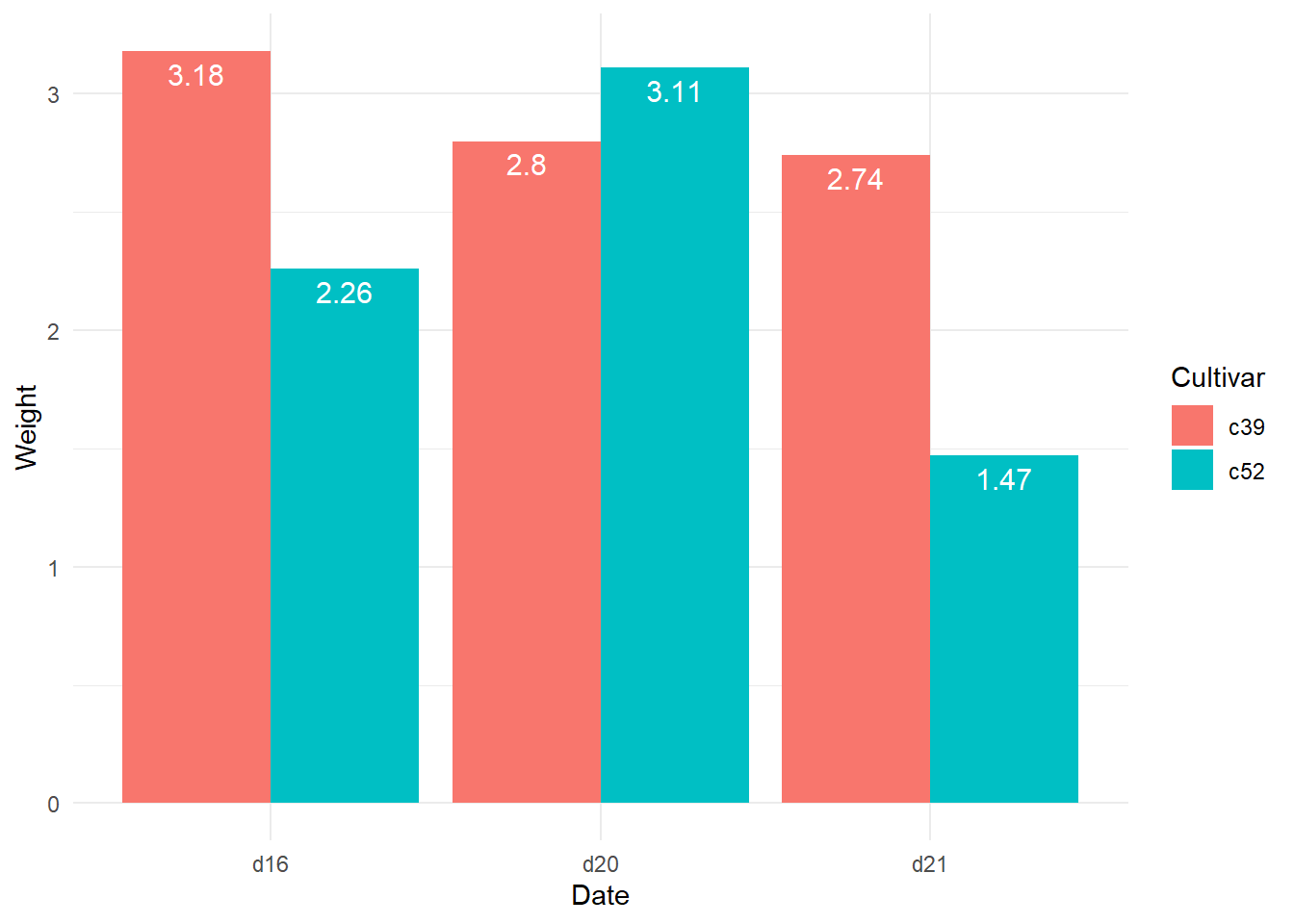# Chapter 5 Data Visualization II

## 5.1 The Review of Key Concepts in ggplot2

• The ggplot2 package is an R package for data visualization.

• The ggplot2 package was developed to create a graphic by combining few graphical components (e.g., data, coordinate systems, geometric objects, aesthetics, facets, themes) based on the grammar of graphics (gg in ggplot2 stands for grammar of graphics).

Graphical components Description
Data Data are what we want to visualize and consist of variables.
Coordinate systems Coordinate systems are the space on which the geometric object are organized. For example, we typically use the cartesian coordinate system with x and y axis.
Geoms Geoms are the geometric objects that are drawn to represent the data. For examples, the points, lines, and bars on a plot are geoms. Each geom function (e.g., geom_points()) returns a layer representing a geometric object.
Aesthetics Aesthetics are the visual properties of geoms. For example, the positions of x and y axis, color of points, shape of points, color of lines are the aesthetics. The variable in the data is mapped to the aesthetic of a geometric object. For example, color = country is a specification that maps the variable country in the data to the color aesthetic. The specific function that maps a variable to an aesthetic is called scales.
Scales The scale function (e.g., scale_fill_brewer()) maps data values to the visual values of an aesthetic. For example, using the scale_fill_brewer() function, we can change the mapping from data values to the colors. That is, we can change the colors of geoms. In this sense, the scales control the aesthetic mapping.
Stats A statistical transformation (stats for short) creates new variables to plots (e.g., counts, prop). A stat function (e.g., stat_count for a bar plot) is an alternative way to build a layer. A stat takes a dataset as input, and returns a dataset as output.
Facets Facets divide a plot into multiple subplots based on the values of one or more discrete variables.
Themes Theme elements are the non-data elements of a graph, such as titles, fonts, ticks, and labels.
• In the ggplot2 package, a graph is the layers of graphical components.

## 5.2 An example of a graph in the ggplot2

• (Data) We will use the mpg dataset in the ggplot2 package. For more details about the mpg data, type ?mpg in the console.
mpg
## # A tibble: 234 x 11
##    manufacturer model    displ  year   cyl trans   drv     cty   hwy fl    class
##    <chr>        <chr>    <dbl> <int> <int> <chr>   <chr> <int> <int> <chr> <chr>
##  1 audi         a4         1.8  1999     4 auto(l~ f        18    29 p     comp~
##  2 audi         a4         1.8  1999     4 manual~ f        21    29 p     comp~
##  3 audi         a4         2    2008     4 manual~ f        20    31 p     comp~
##  4 audi         a4         2    2008     4 auto(a~ f        21    30 p     comp~
##  5 audi         a4         2.8  1999     6 auto(l~ f        16    26 p     comp~
##  6 audi         a4         2.8  1999     6 manual~ f        18    26 p     comp~
##  7 audi         a4         3.1  2008     6 auto(a~ f        18    27 p     comp~
##  8 audi         a4 quat~   1.8  1999     4 manual~ 4        18    26 p     comp~
##  9 audi         a4 quat~   1.8  1999     4 auto(l~ 4        16    25 p     comp~
## 10 audi         a4 quat~   2    2008     4 manual~ 4        20    28 p     comp~
## # ... with 224 more rows
• The ggplot() function initialize the ggplot object.
ggplot(data = mpg)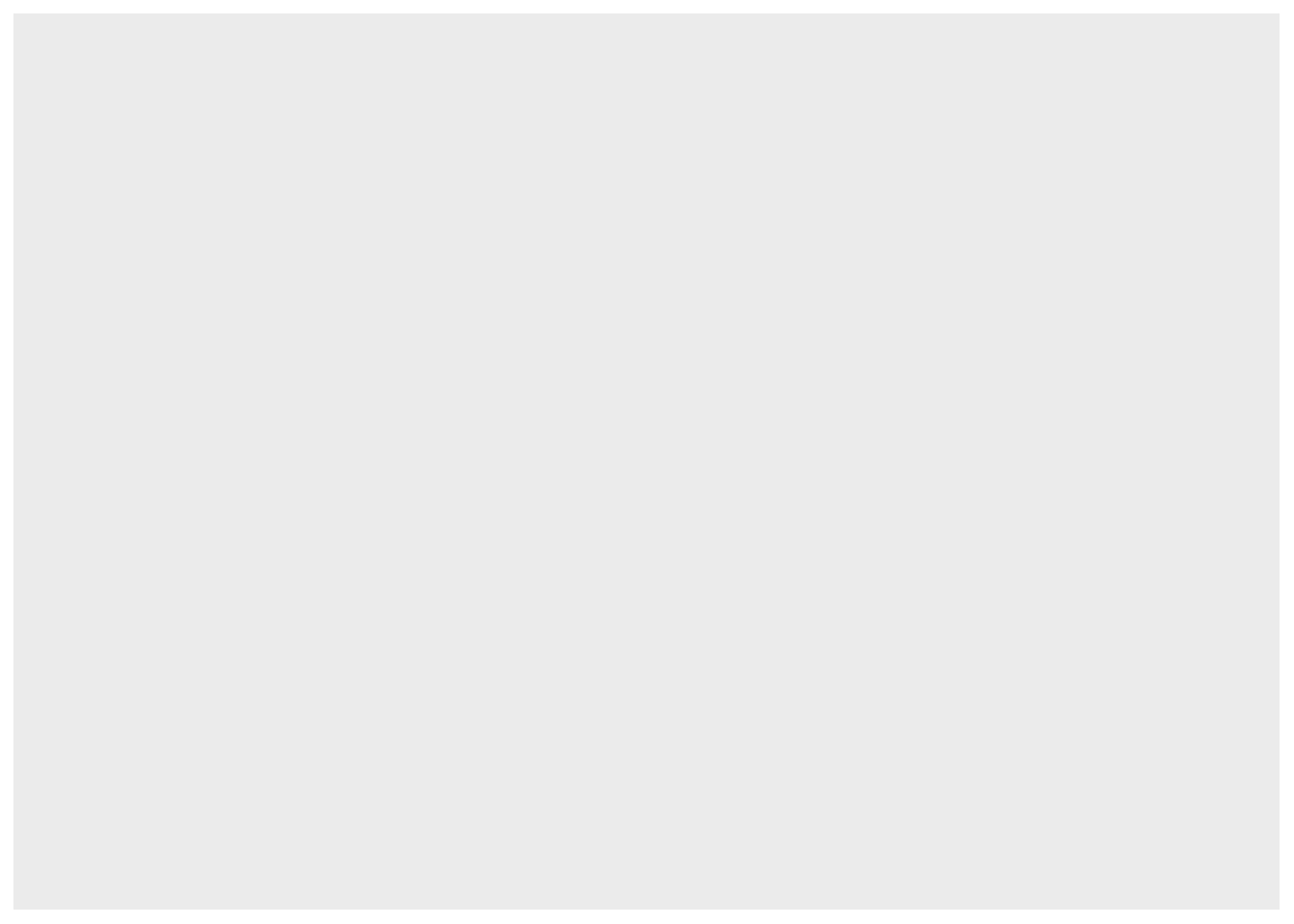• (Aesthetic) The aes() function is used for the aesthetic mapping between variables and aesthetic. In this example, we mapped the class variable to the x position aesthetic of the x axis.
ggplot(data = mpg, aes(x = class))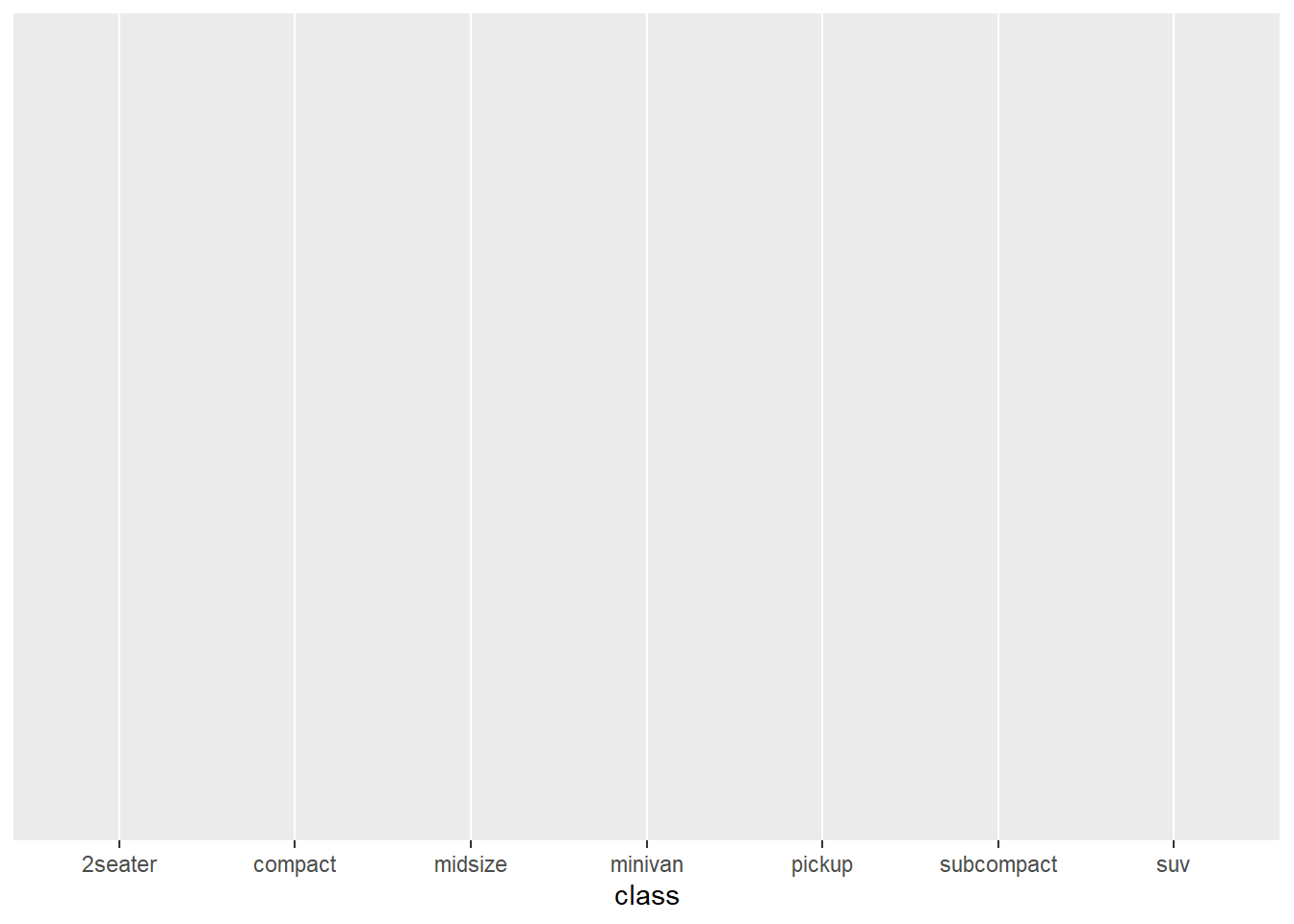• (Geoms) The geom_bar() add a layer representing the bars.
ggplot(data = mpg, aes(x = class)) +
geom_bar()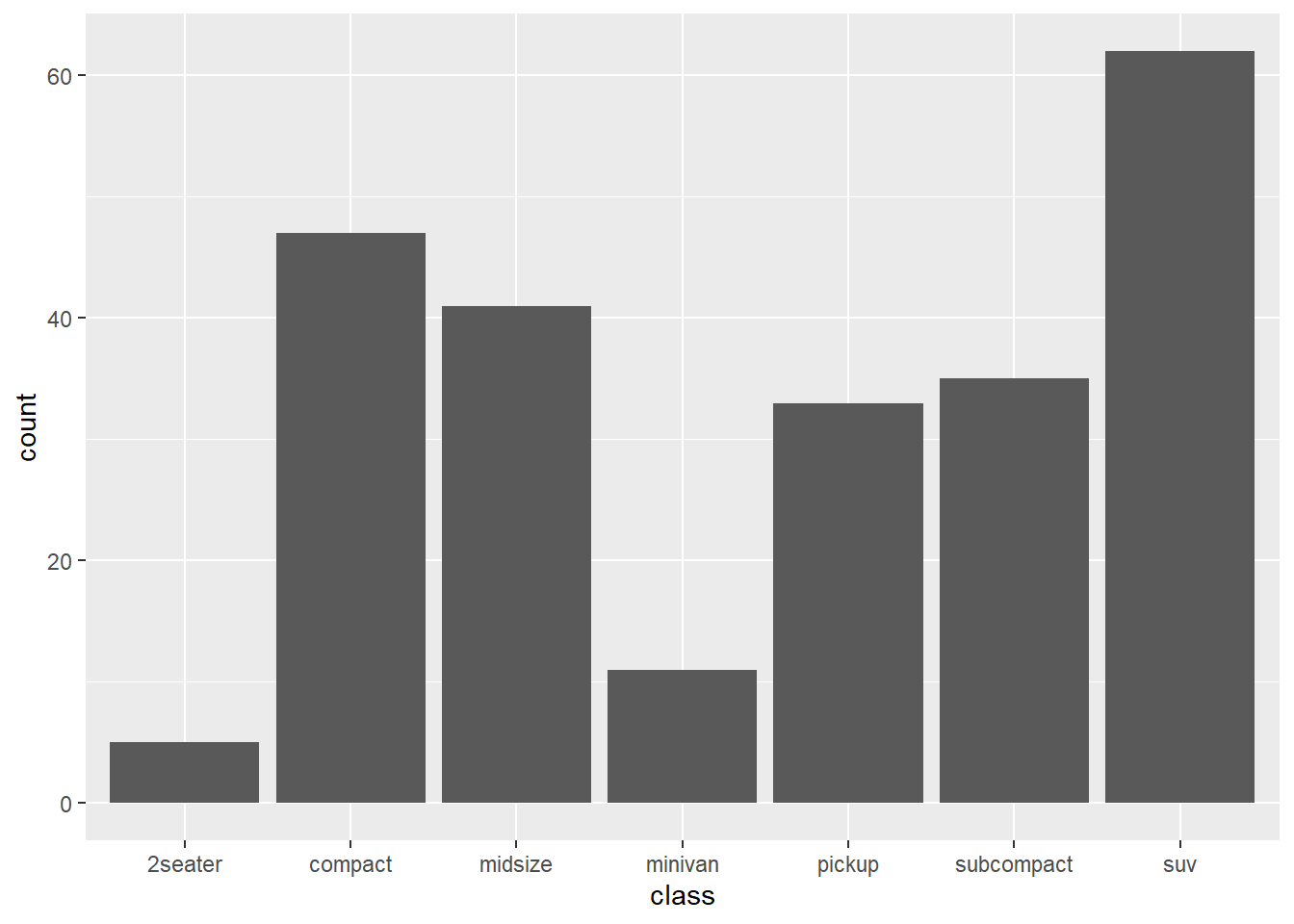• (Aesthetic) The fill = manufacturer maps the manufacturer variable in the mpg to the fill aesthetic (i.e., color inside bars)
ggplot(data = mpg, aes(x = class, fill = manufacturer)) +
geom_bar()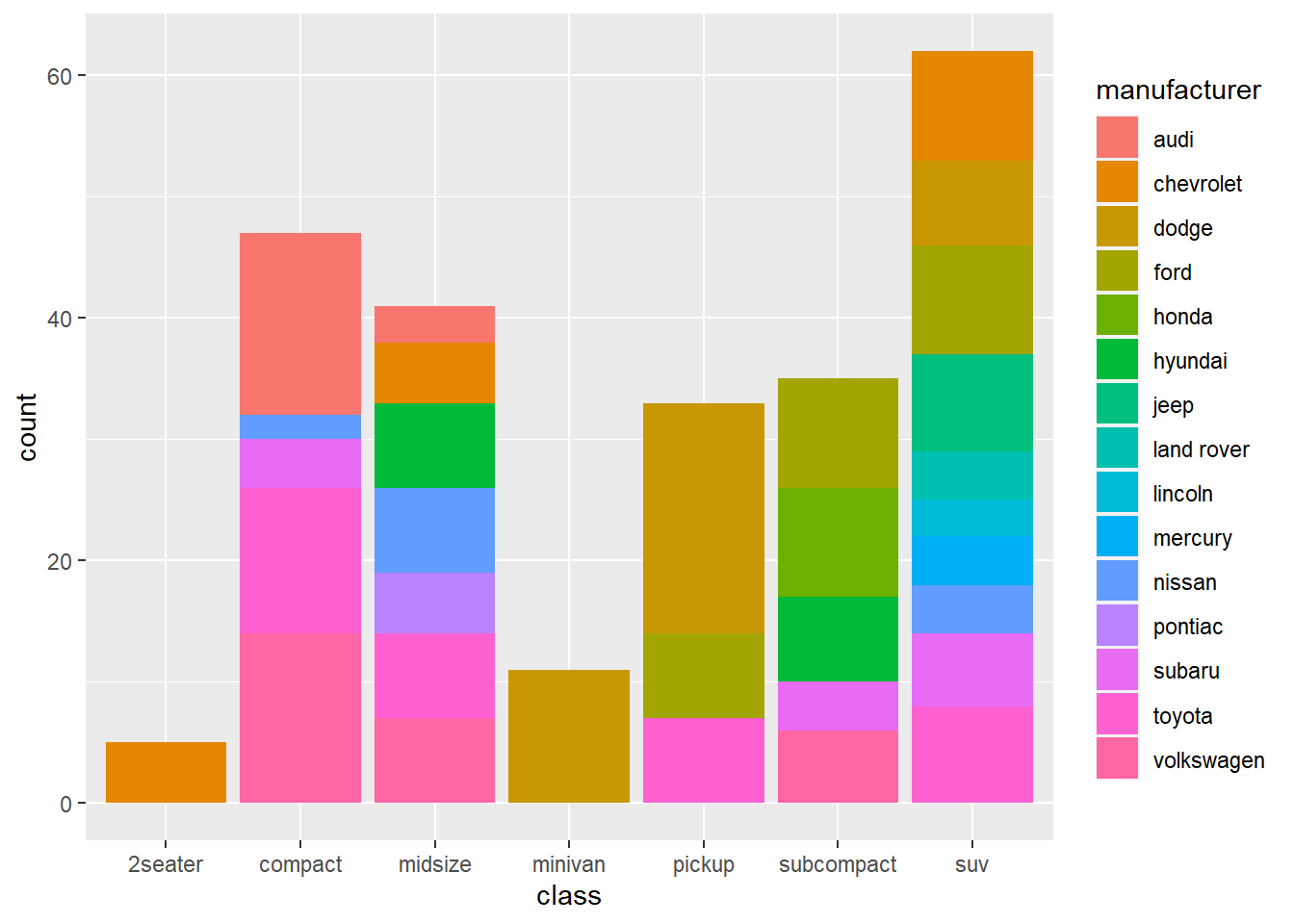• (Stats) The geom_bar() makes the height of the bar proportional to the number of cases in each group. That is, the geom_bar() needs to calculate a new variable, the number of cases in each group, to create a bar plot. By default, the geom_bar() uses stat_count() which counts the number of cases at each x position. So stat_count() creates the same graph.
ggplot(data = mpg, aes(x = class, fill = manufacturer)) +
stat_count()• (facets) There are two types of faceting provided by ggplot2: facet_grid() and facet_wrap(). facet_grid() produces a 2d grid of panels defined by variables which form the rows and columns, while facet_wrap() produces a 1d ribbon of panels that is wrapped into 2d. In this example, facet_wrap() is used to creates multiple subplots based on the values of the manufacturer variable.
ggplot(data = mpg, aes(x = class)) +
geom_bar() +
facet_wrap(vars(manufacturer))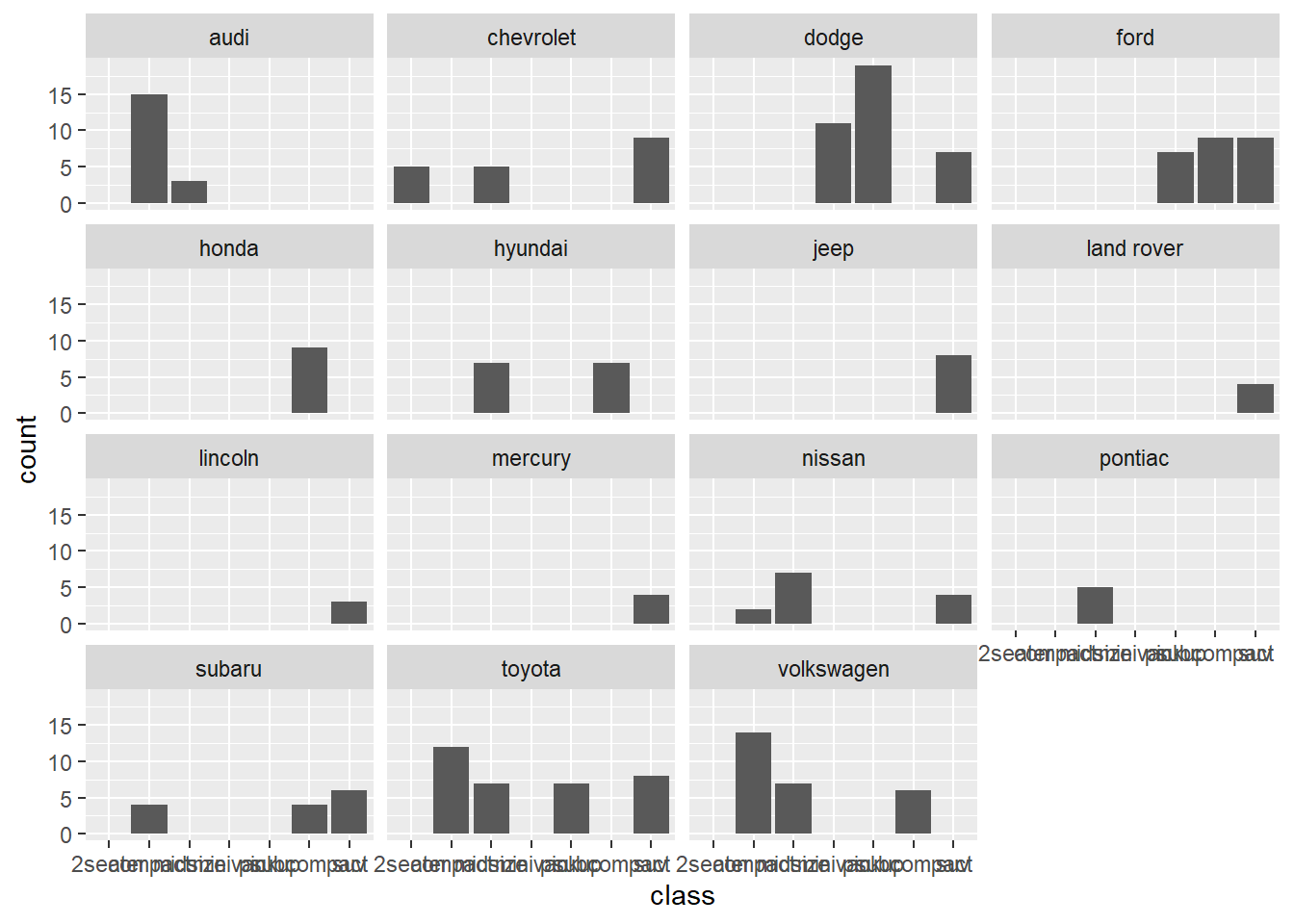# x = class has been changed to y = class for the horizontal bar plot
ggplot(data = mpg, aes(y = class)) +
geom_bar() +
facet_wrap(vars(manufacturer))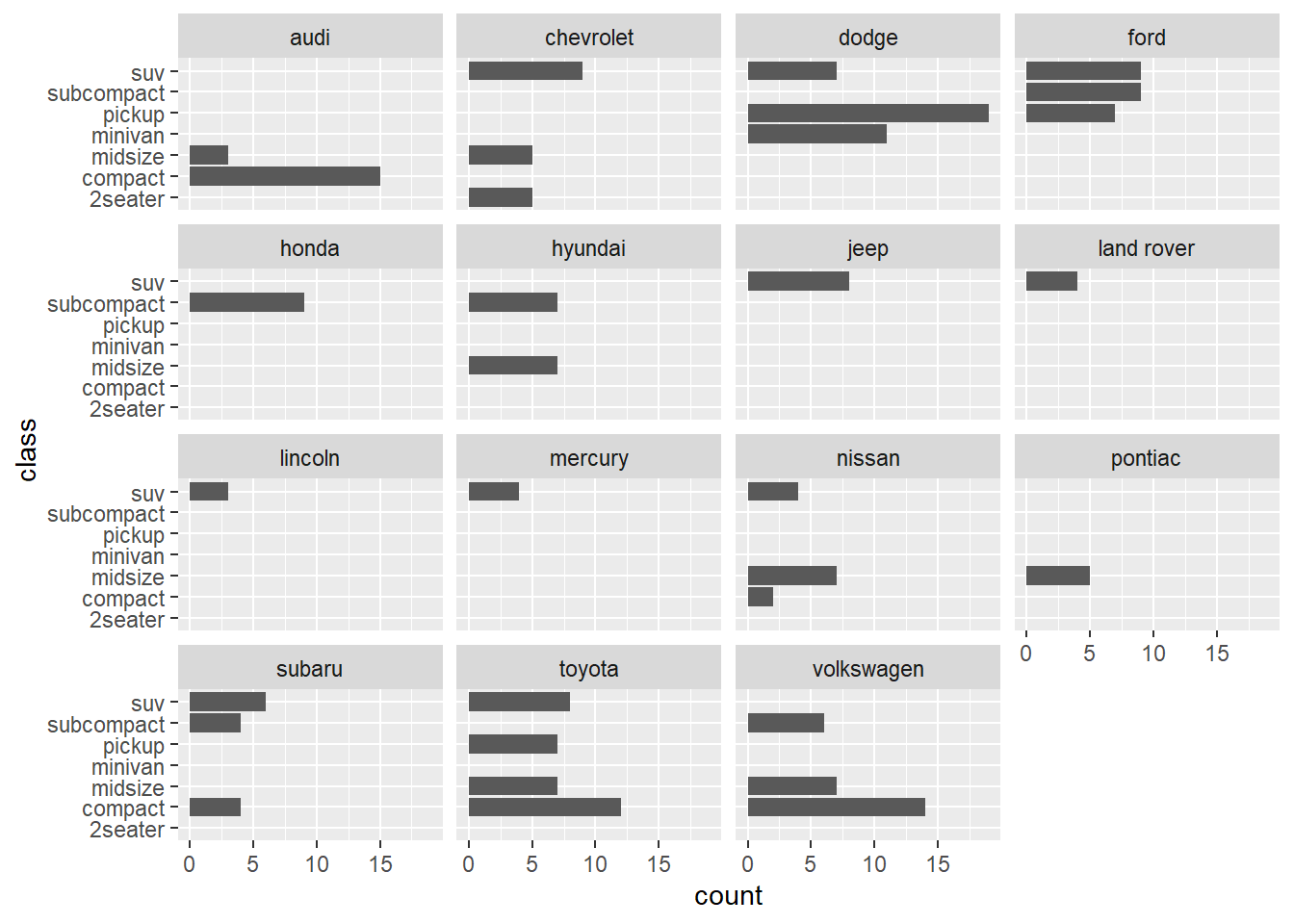## 5.3 Themes

• You can create a graph by combining graphical components of the ggplot2 package based on your data. Now, it’s time to make your graph more pretty and informative.

“Theme elements specify the non-data elements that you can control. For example, the plot.title element controls the appearance of the plot title; axis.ticks.x, the ticks on the x axis; legend.key.height, the height of the keys in the legend.”

“Each element is associated with an element function, which describes the visual properties of the element. For example, element_text() sets the font size, colour and face of text elements like plot.title.”

“The theme() function which allows you to override the default theme elements by calling element functions, like theme(plot.title = element_text(colour = "red")).”

“Complete themes, like theme_grey() set all of the theme elements to values designed to work together harmoniously”

• Here is the theme elements of a graph in the ggplot2 package.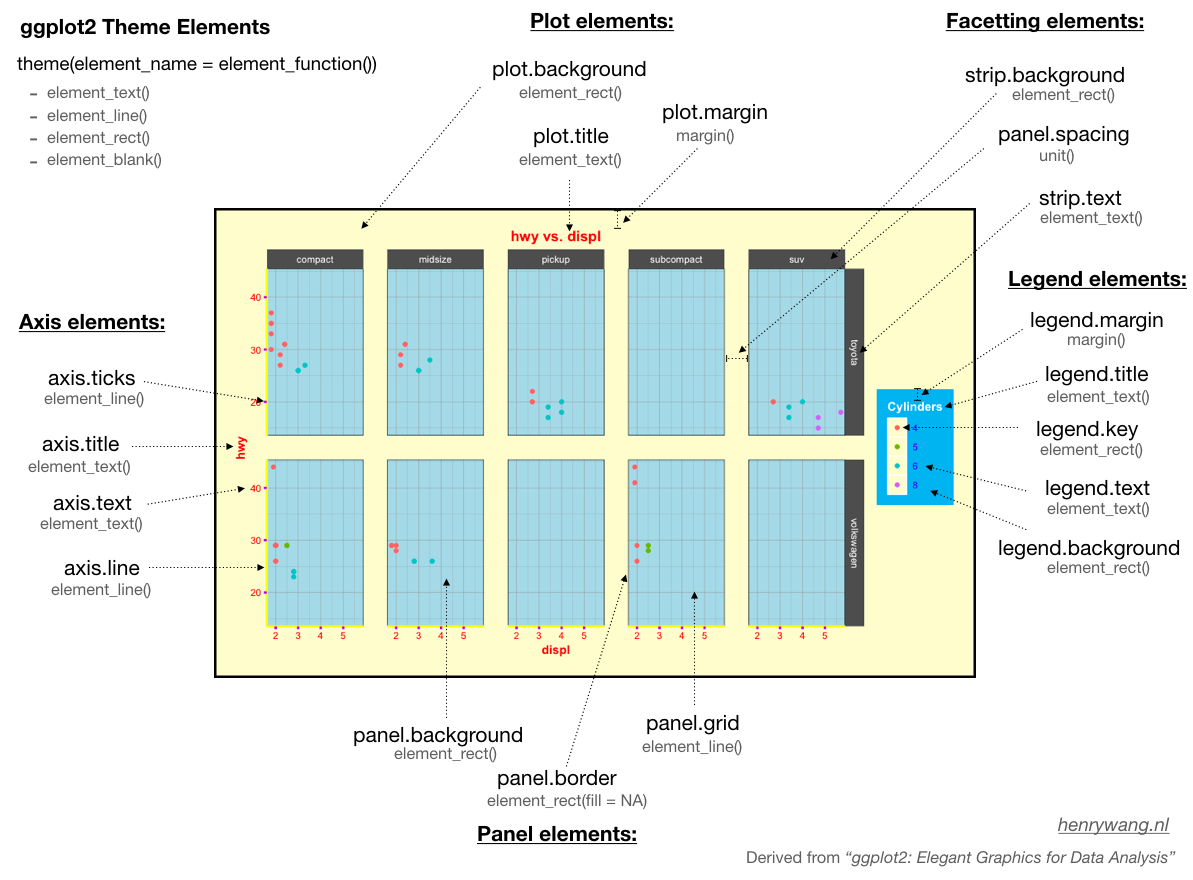from henrywang.nl

• When you make your graph more pretty and informative, it is almost impossible to memorize all the functions and options for each of specific modifications of your graph. Again, Goolgling is the best way to achieve your goal of making your graph pretty and informative. In order to find the solutions to your problems quickly, you need to know the terminologies for the elements of a graph. Then, you can google using the right keywords (e.g., “increase the size of font on axis label”, “change the position of legend”).

## 5.4 Modify axis, legend, and plot labels

• labs() is used to modify axis, legend, and plot labels. Please compare the graph below with the one above to check which labels have been changed by labs(). Check here for more details about labs().
ggplot(data = mpg, aes(x = class, fill = manufacturer)) +
geom_bar() +
labs(title = "Counts of Car Class",
subtitle = "By manufacturer",
caption = "source: mpg data from ggplot2",
fill = "Car Company",
x = "Class of Cars",
y = "Count")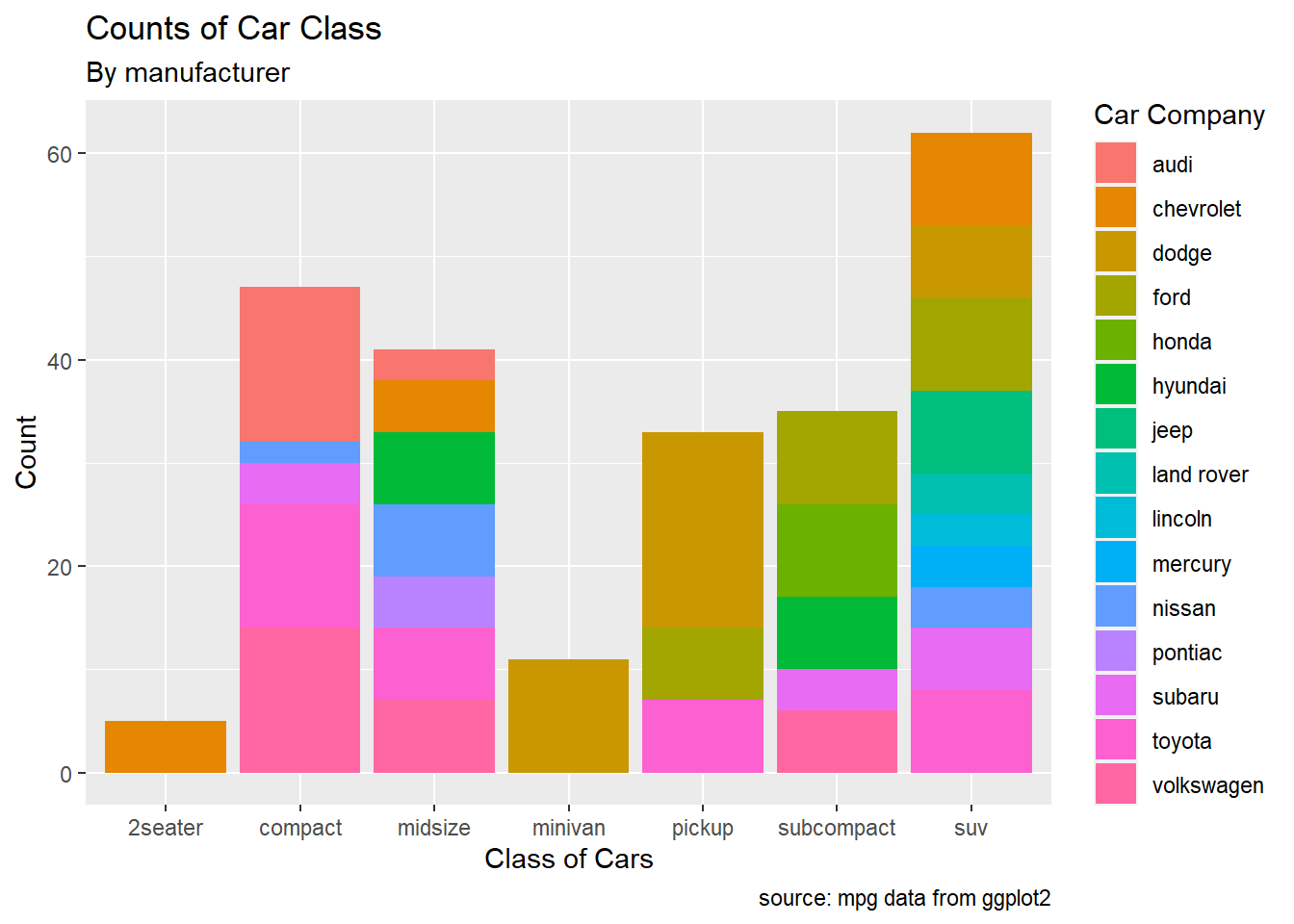## 5.5 Axes

• ggplot will display the axes with defaults that look good in most cases, but you might want to control, for example, the axis labels, the number and placement of tick marks, the tick mark labels, and so on.

### 5.5.1 Setting the Position of Tick Marks

• Often, we want to set the tick marks on the axis
ggplot(mpg, aes(x = displ, y = hwy)) +
geom_point()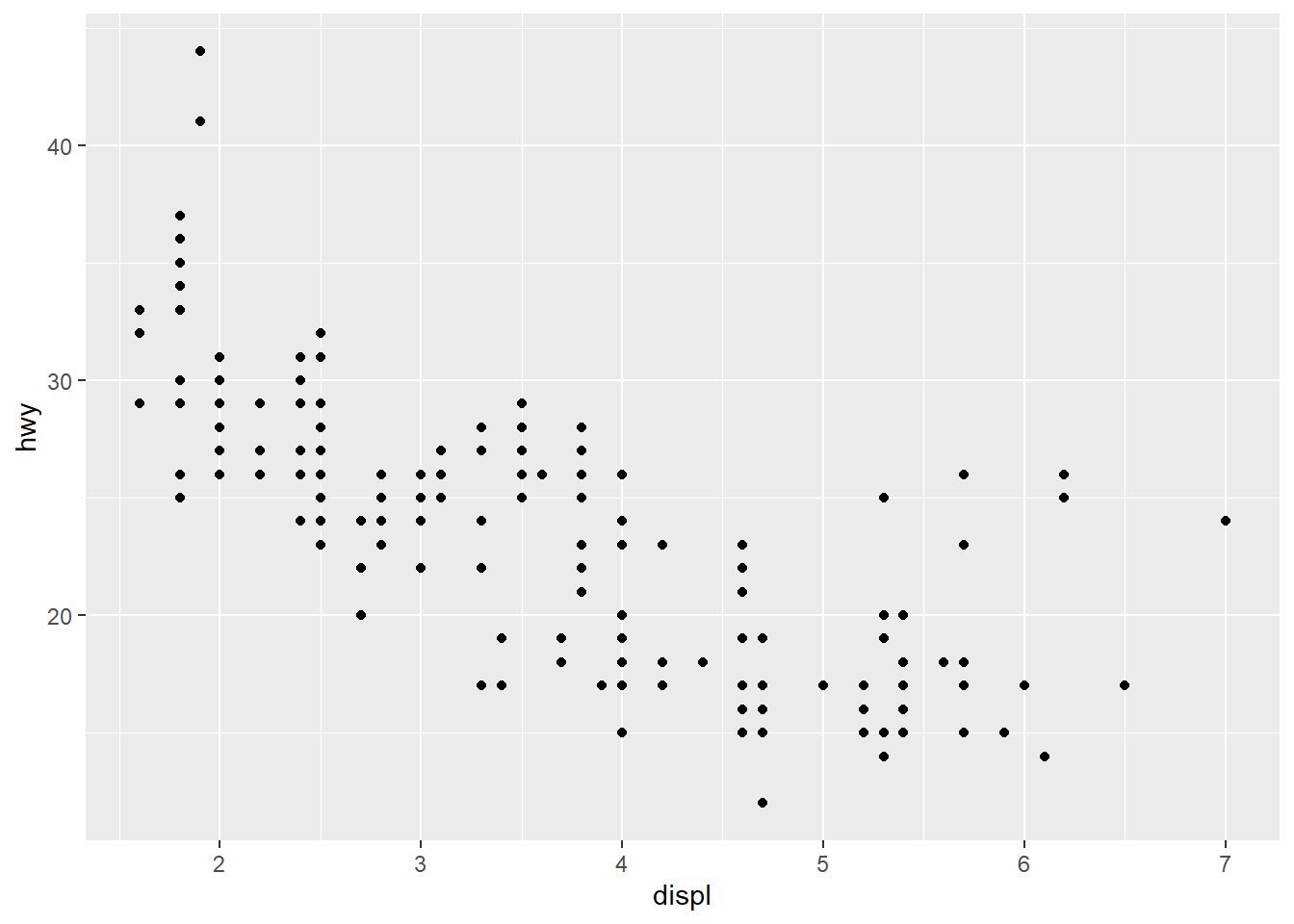• breaks sets the tick marks.
ggplot(mpg, aes(x = displ, y = hwy)) +
geom_point() +
scale_x_continuous(breaks = c(2,4,6)) +
scale_y_continuous(breaks = c(15, 25, 35, 45))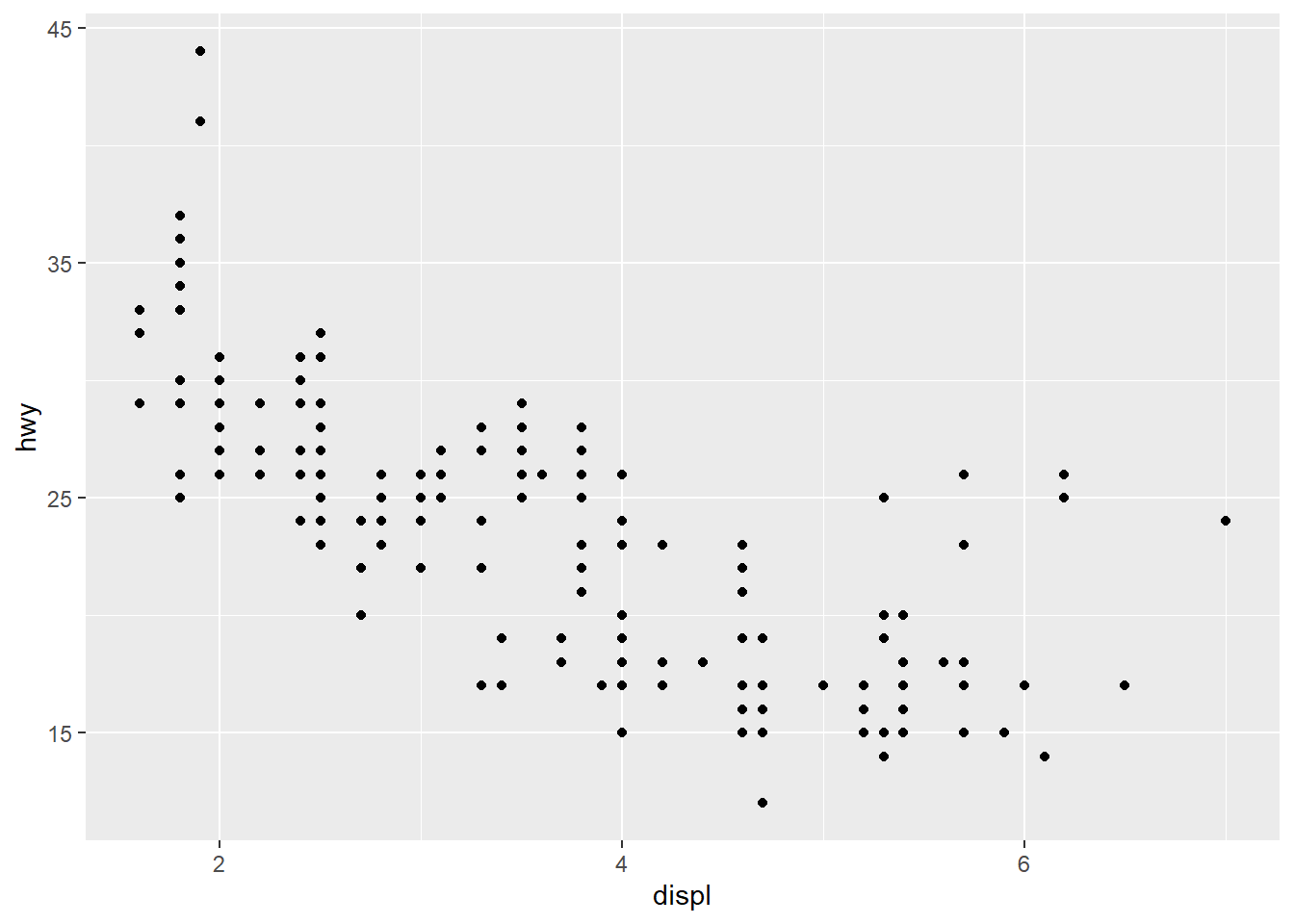### 5.5.2 Changing the Text of Tick Labels

• labels sets the tick labels.
ggplot(mpg, aes(x = displ, y = hwy)) +
geom_point() +
scale_x_continuous(breaks = c(2,4,6), labels = c("2 cylinders", "4 cylinders", "6 cylinders")) +
scale_y_continuous(breaks = c(15, 25, 35, 45))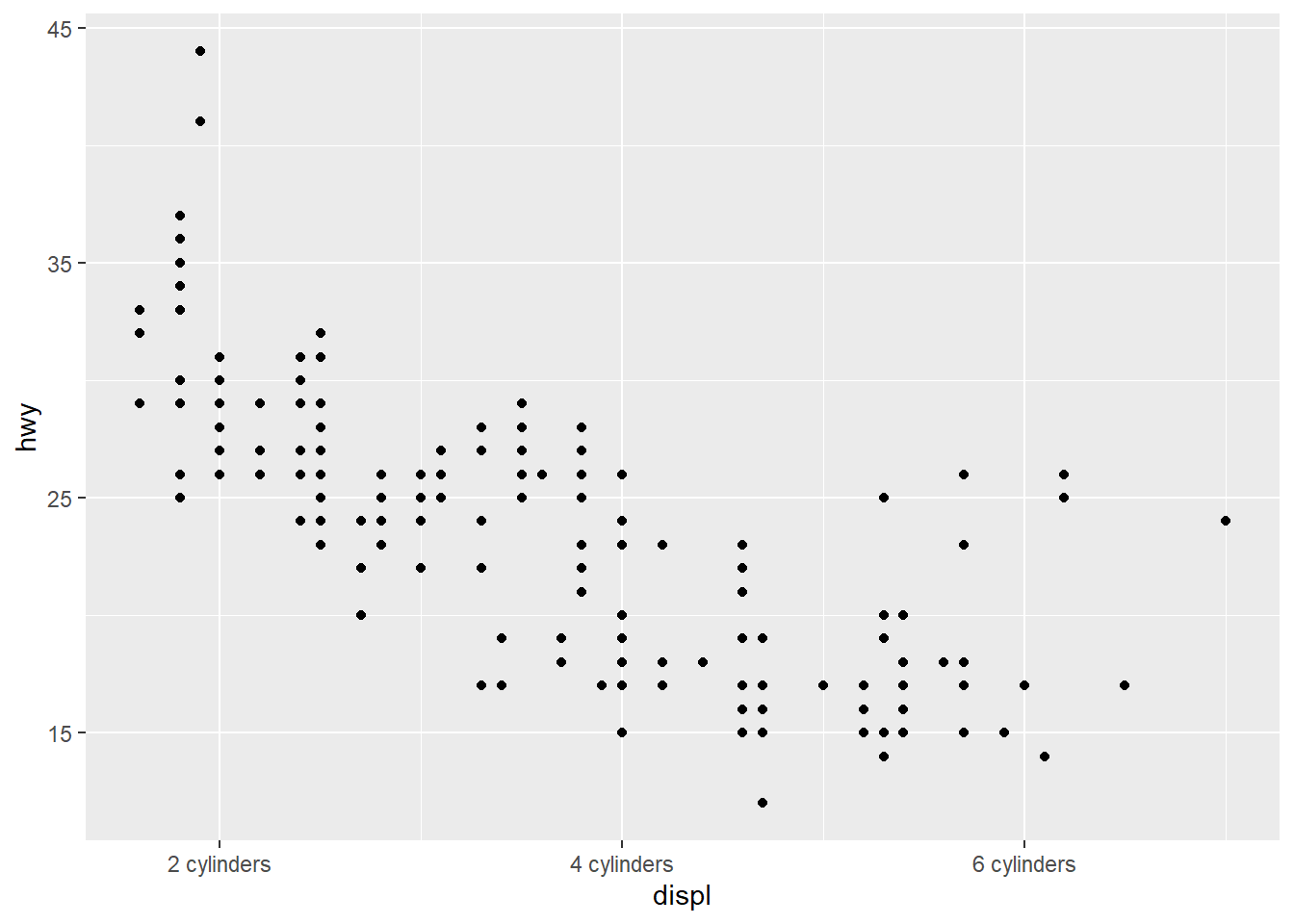### 5.5.3 Rotating your tick labels

• You can use theme().
ggplot(data = mpg, aes(x = class, fill = manufacturer)) +
geom_bar()# rotate 30 degrees
ggplot(mpg, aes(x = model, y = hwy)) +
geom_boxplot() +
theme(axis.text.x = element_text(angle = 30))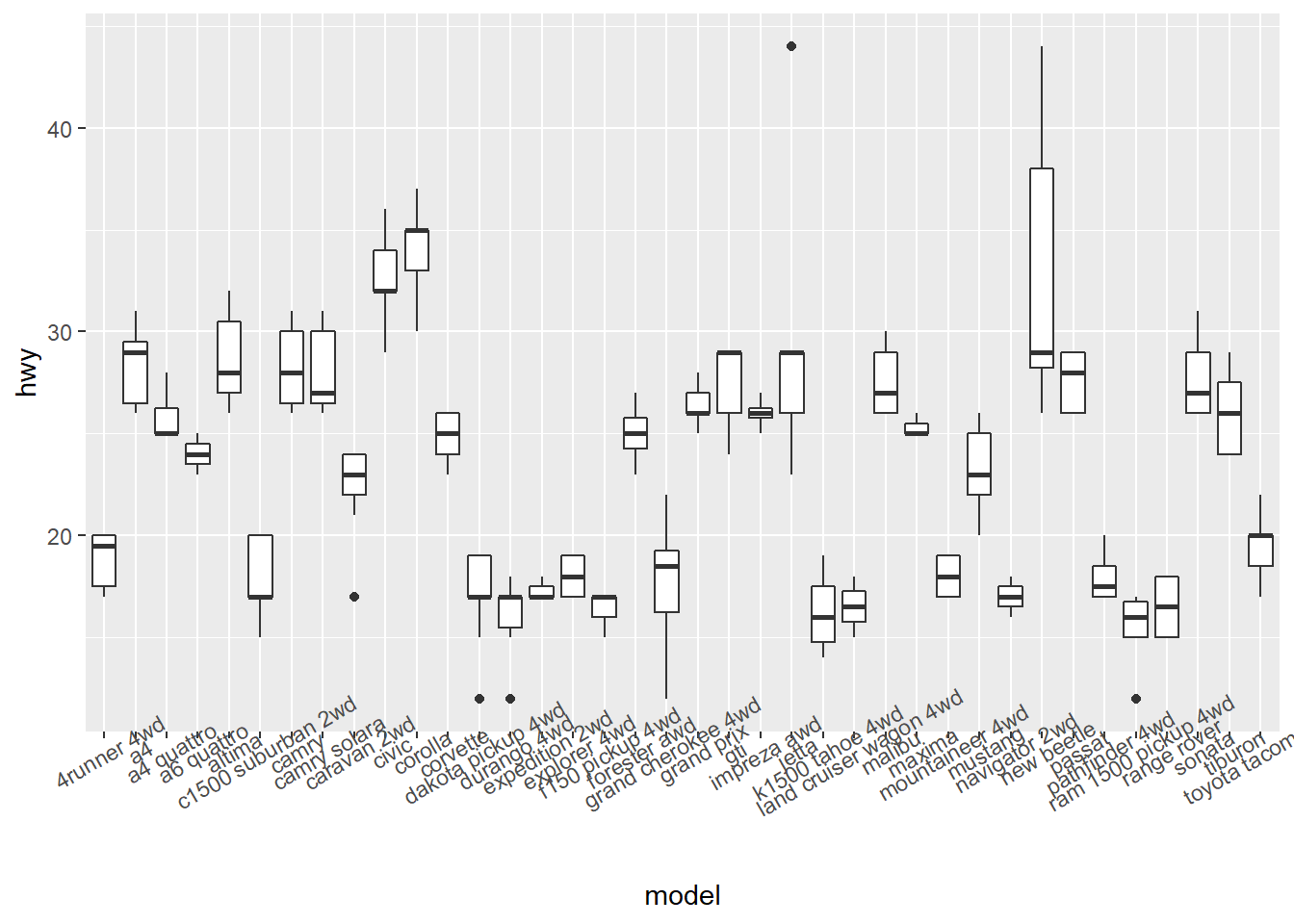# rotate 90 degrees
ggplot(mpg, aes(x = model, y = hwy)) +
geom_boxplot() +
theme(axis.text.x = element_text(angle = 90))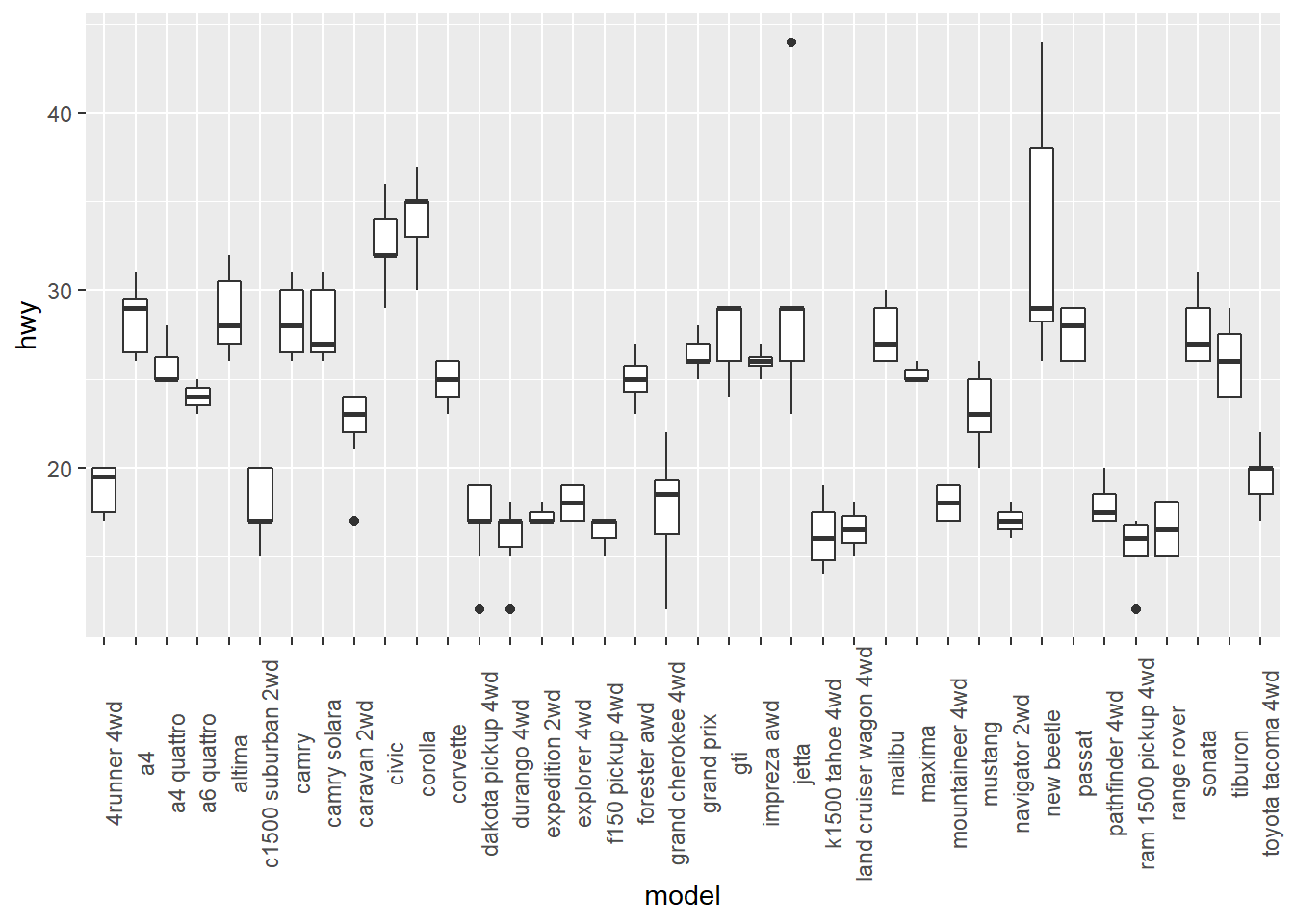### 5.5.4 Swapping X- and Y-Axes

• You can’t read the tick labels
ggplot(mpg, aes(x = model, y = hwy)) +
geom_boxplot()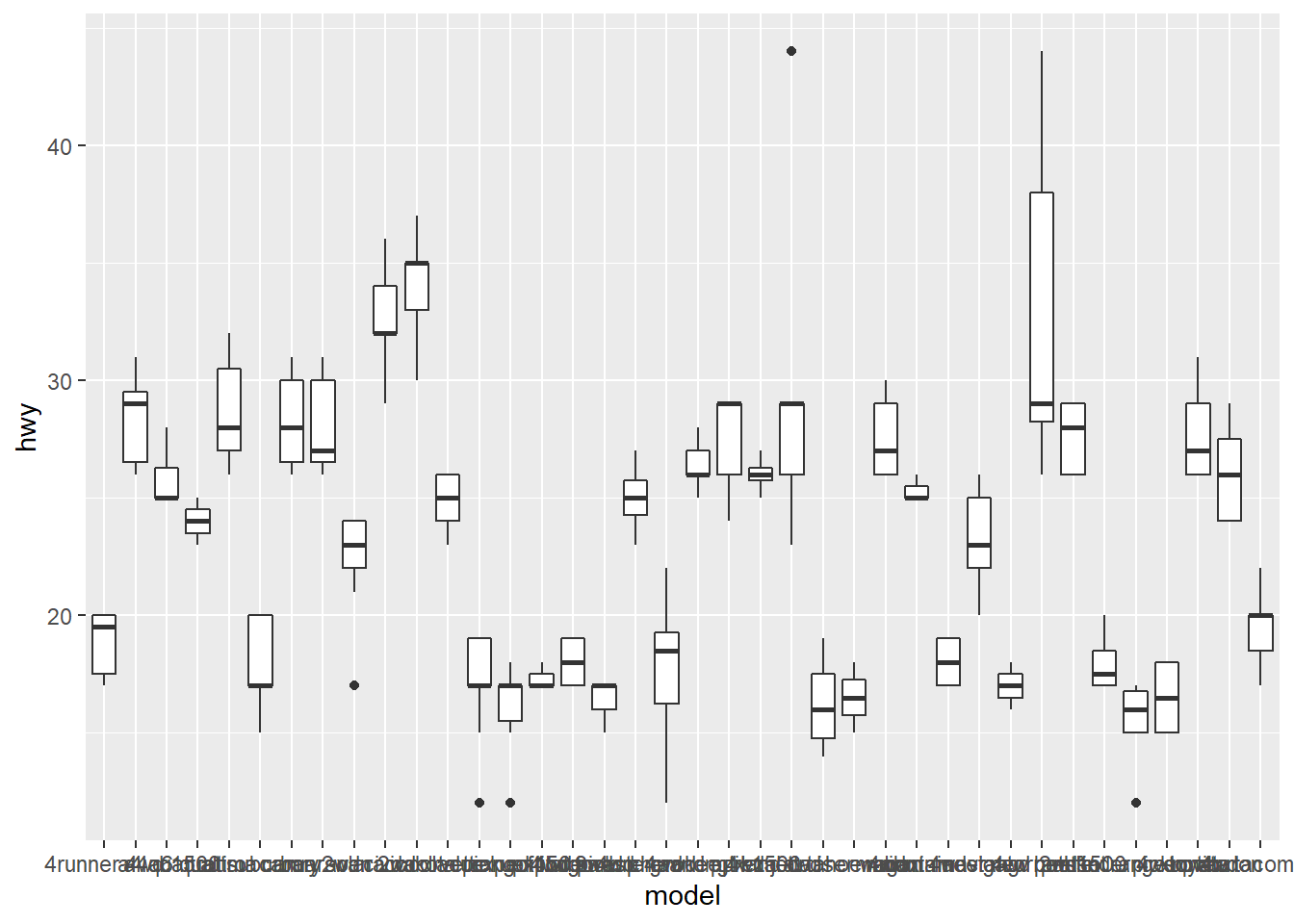• coord_flip() flips the axes
ggplot(mpg, aes(x = model, y = hwy)) +
geom_boxplot() +
coord_flip()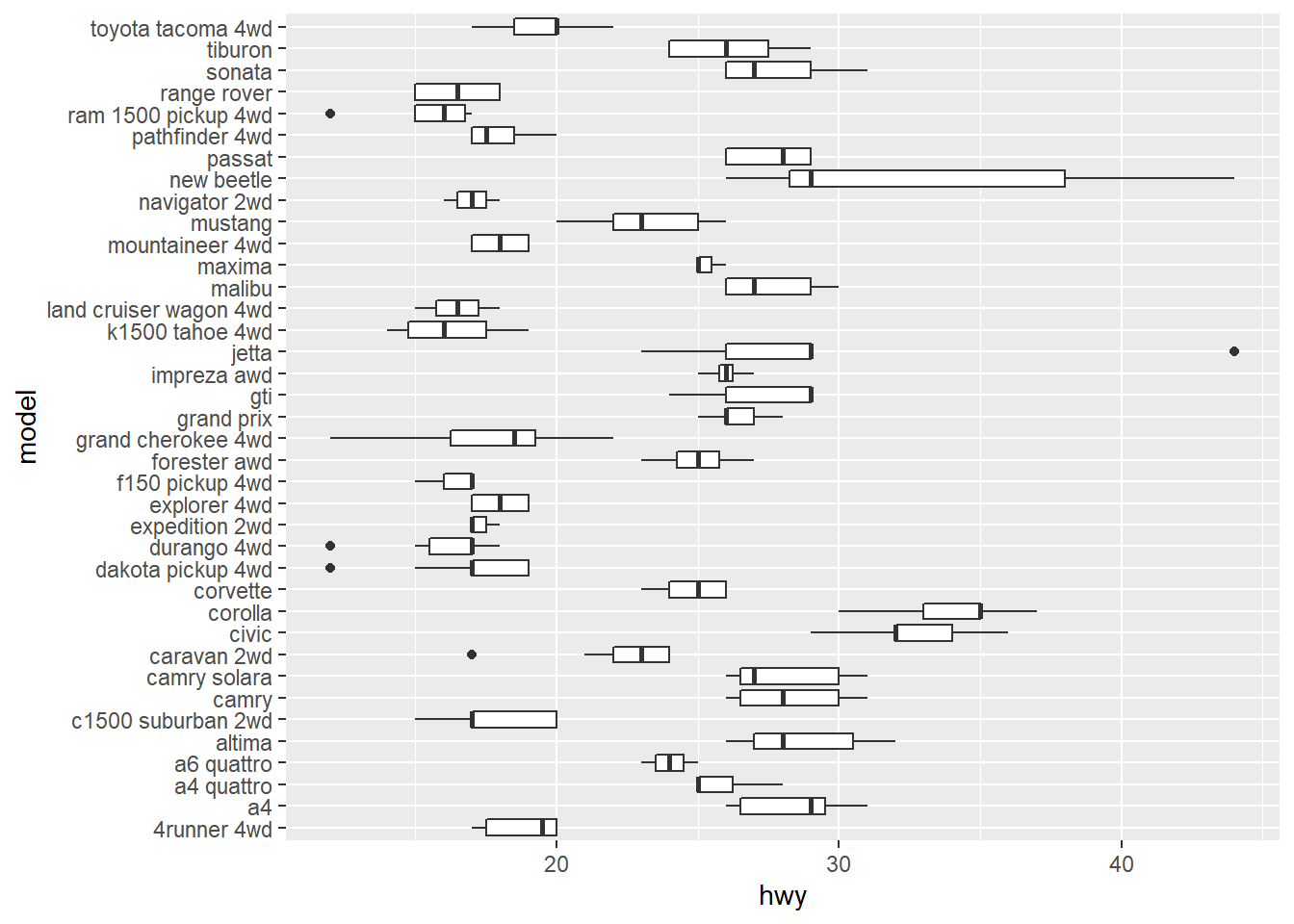ggplot(mpg, aes(x = hwy, y = model)) +
geom_boxplot()## 5.6 Legends

• The PlantGrowth data are the results from an experiment to compare yields (as measured by dried weight of plants) obtained under a control and two different treatment conditions.
pg_plot <- ggplot(PlantGrowth, aes(x = group, y = weight, fill = group)) +
geom_boxplot()
pg_plot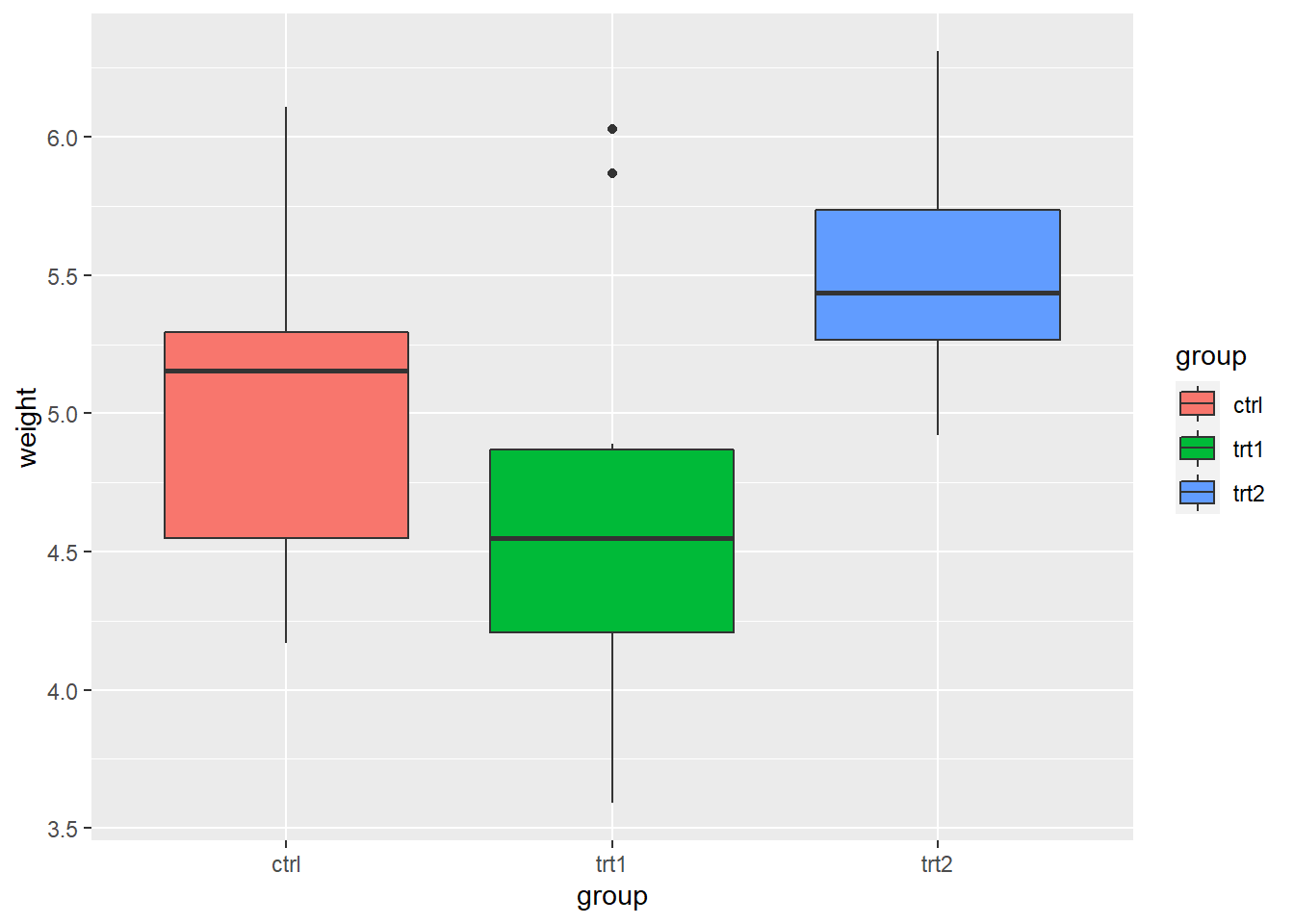• Use labs() and set the value of fill, colour, shape, or whatever aesthetic is appropriate for the legend
pg_plot +
labs(fill = "Condition")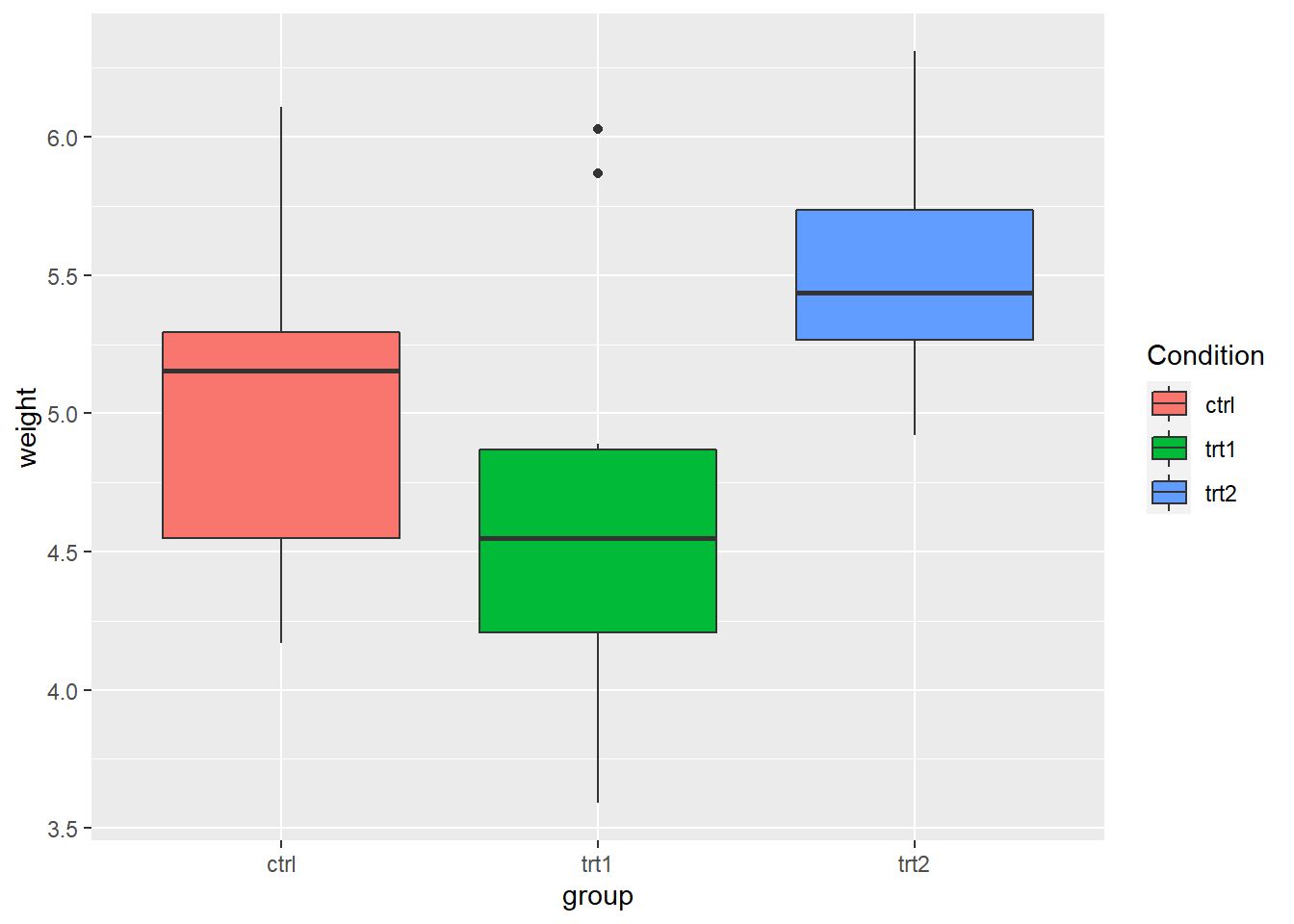• In fact, labs() sets the title, subtitle, caption, x-axis label, y-axis label, and the title of the legend.
pg_plot +
labs(title = "Weight of Plants",
subtitle = "By Experimental Conditions",
caption = "source: PlantGrowth",
x = "Experimental Conditions",
y = "Weight (pounds)",
fill = "Condition")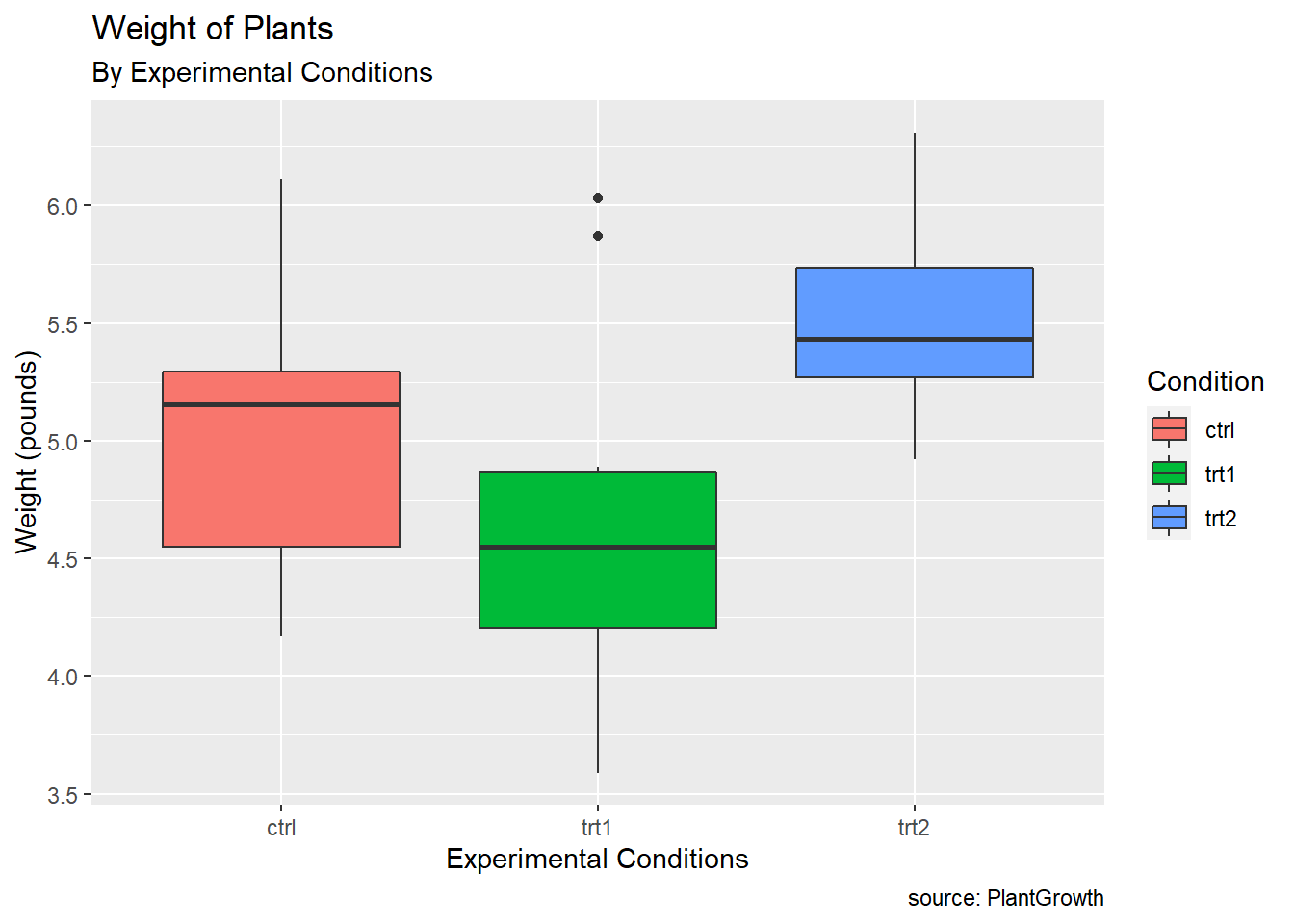• Changing the position of the legend
# removing legend
pg_plot +
theme(legend.position = "none")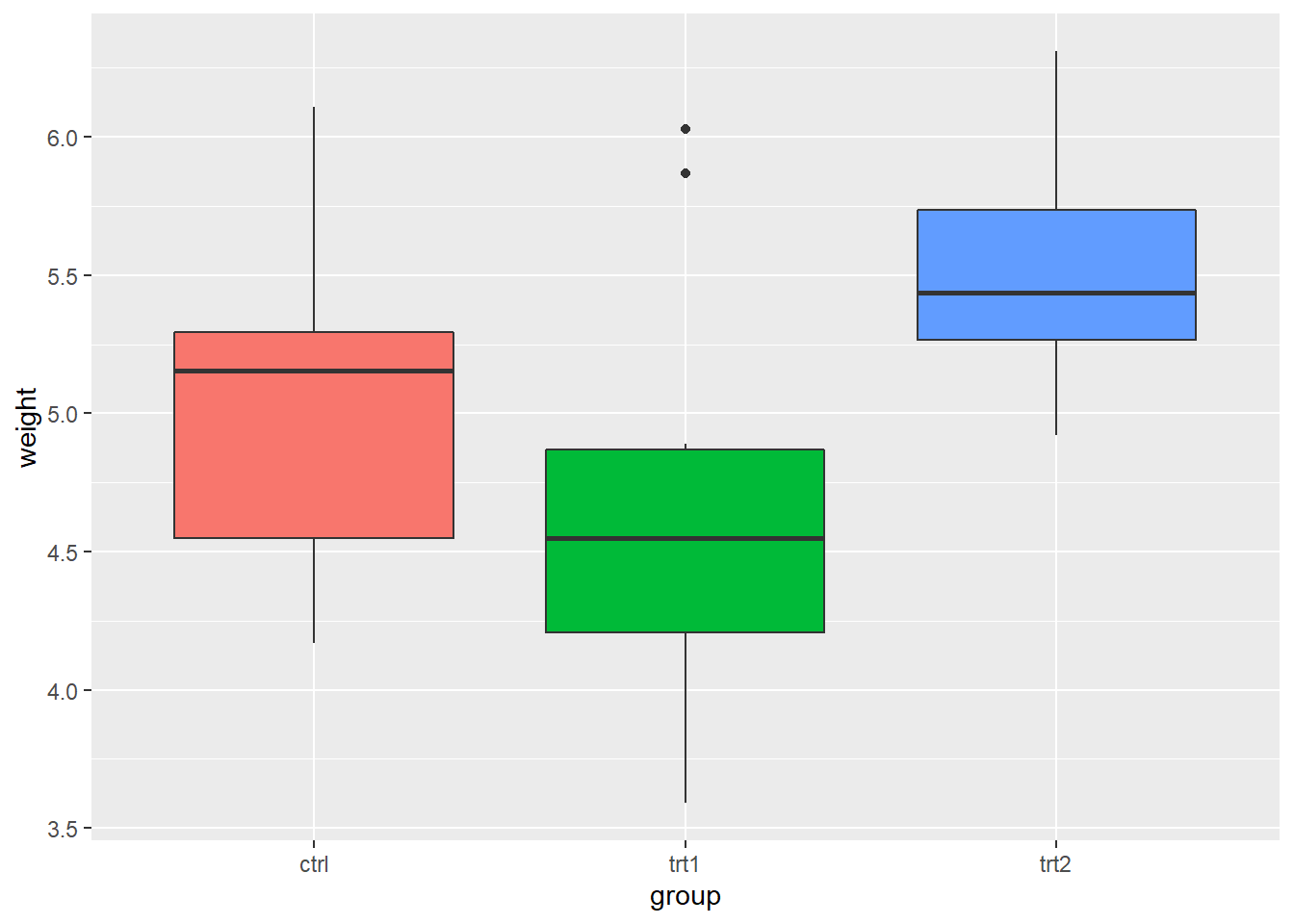# place the legend on the bottom
pg_plot +
theme(legend.position = "bottom")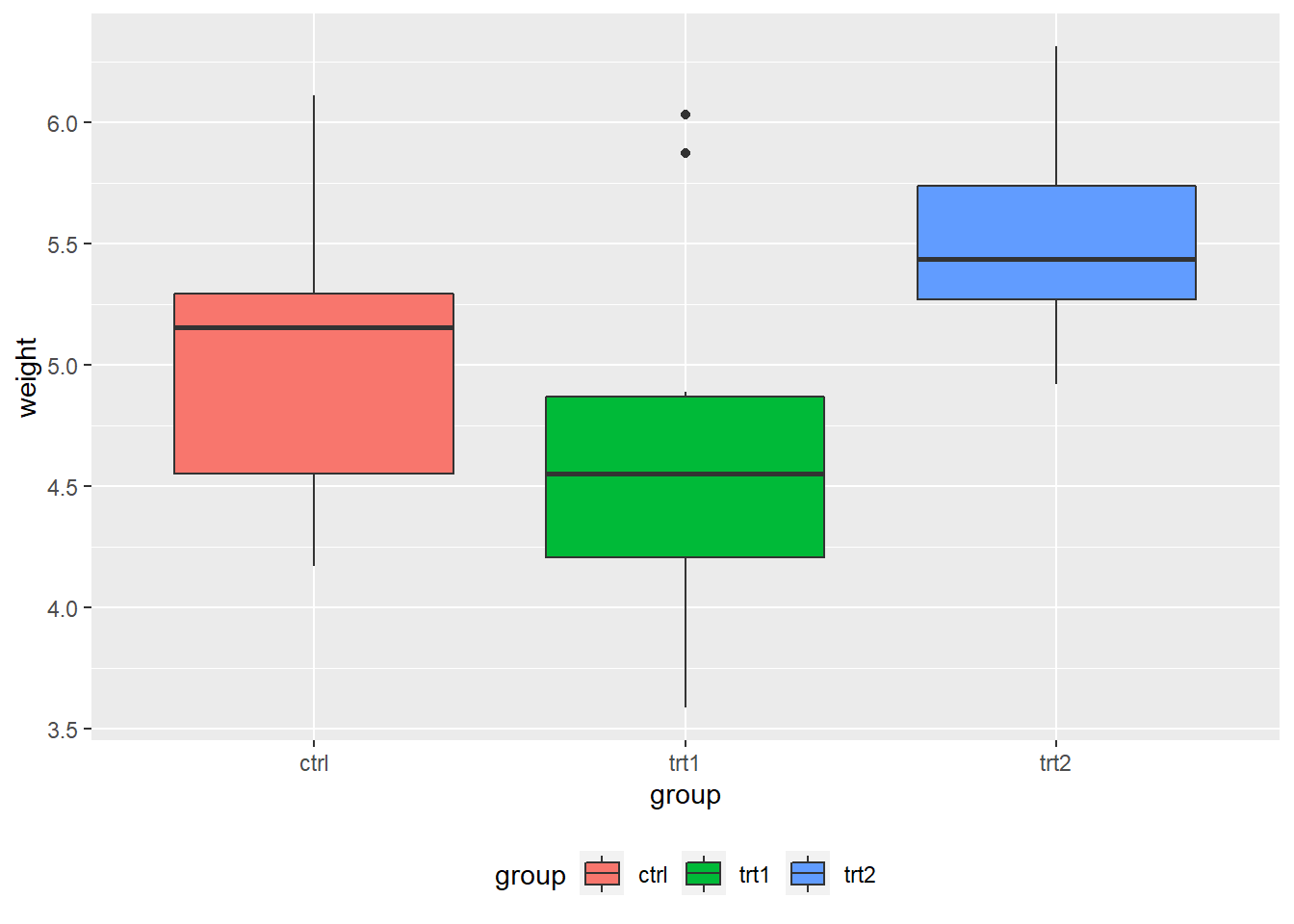# place the legend on the left
pg_plot +
theme(legend.position = "left")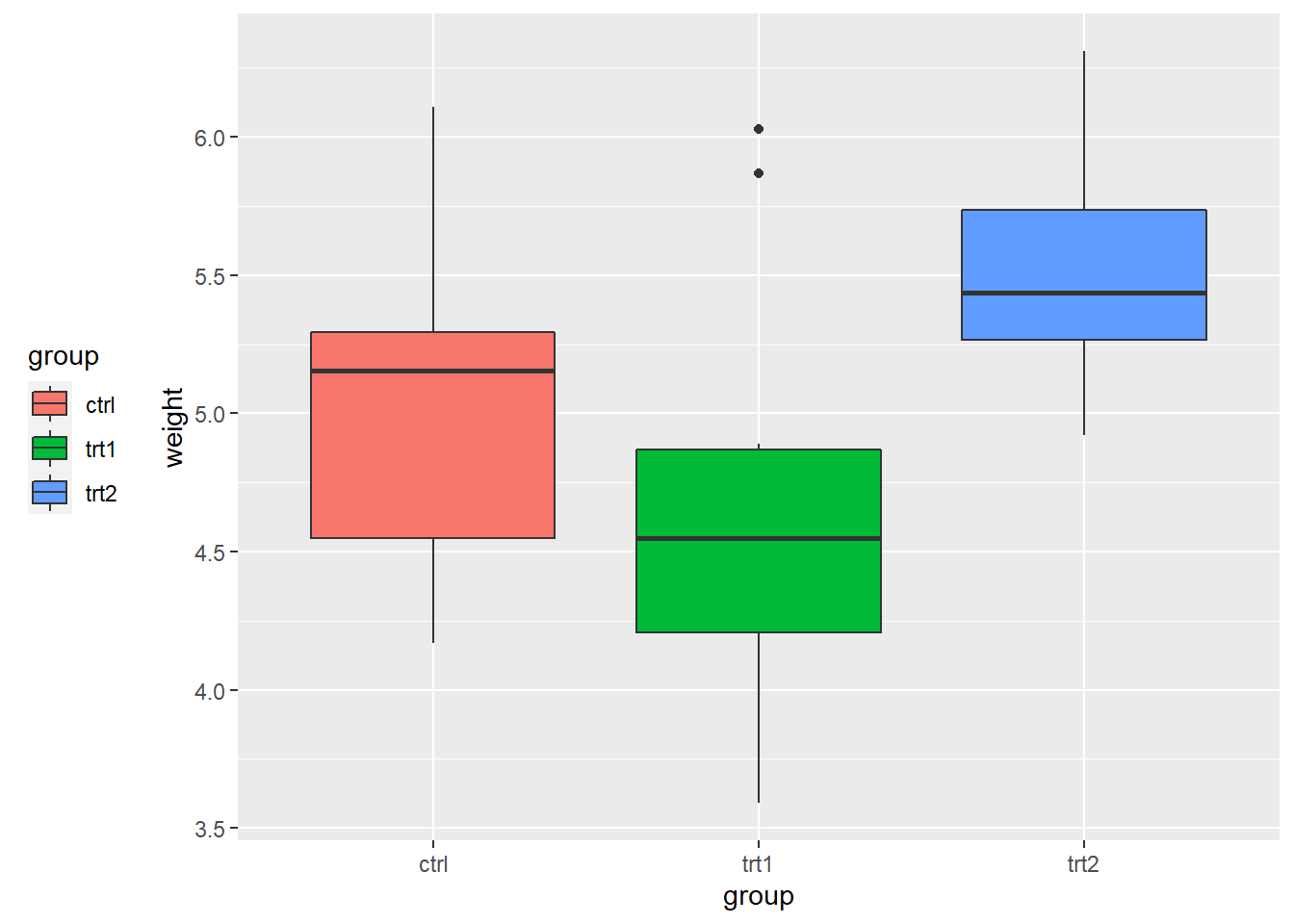# place the legend inside the plot
# The coordinate space starts at (0, 0) in the bottom left and goes to (1, 1) in the top right.
pg_plot +
theme(legend.position = c(.8, .3))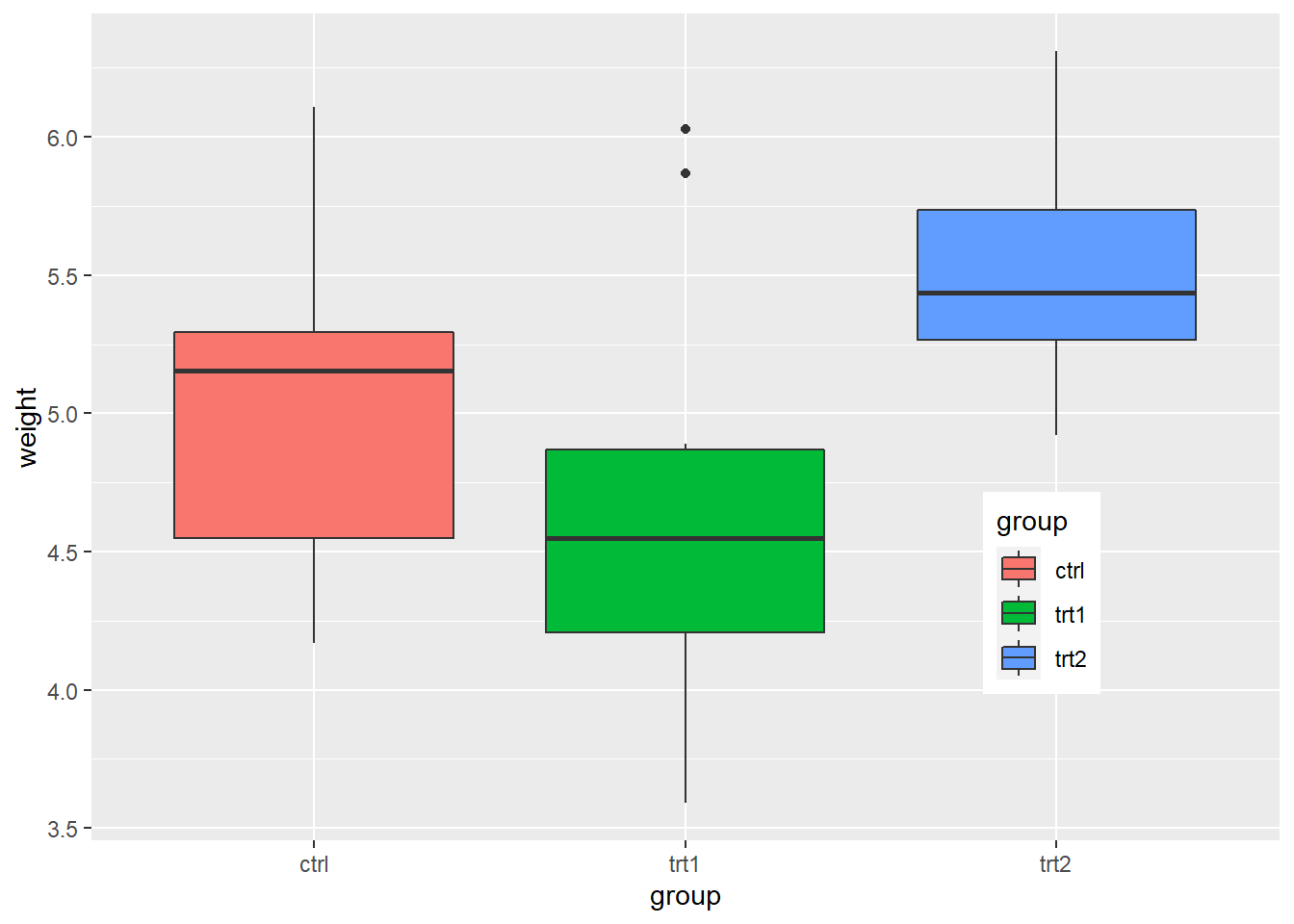## 5.7 Annotations

• Once you create your plot using data, you can add extra contextual information (e.g., text, lines).

• faithful data
# faithful is a built-in data in R
# ?faithful in your console will display the help documentation for the data
# faithful contains waiting time between eruptions and the duration of the eruption for the Old Faithful geyser in Yellowstone National Park, Wyoming, USA.
head(faithful)
##   eruptions waiting
## 1     3.600      79
## 2     1.800      54
## 3     3.333      74
## 4     2.283      62
## 5     4.533      85
## 6     2.883      55
• Let’s create the scatter plot between eruptions (x-axis) and waiting (y-axis)
# A variable name p points to (or binds or references) the ggplot object
# Simply, we just give a name p to the ggplot object
# <- is an assignment operator in R
# e.g., a <- 10  # a variable name a points to the value 10
p <- ggplot(faithful, aes(x = eruptions, y = waiting)) +
geom_point()
p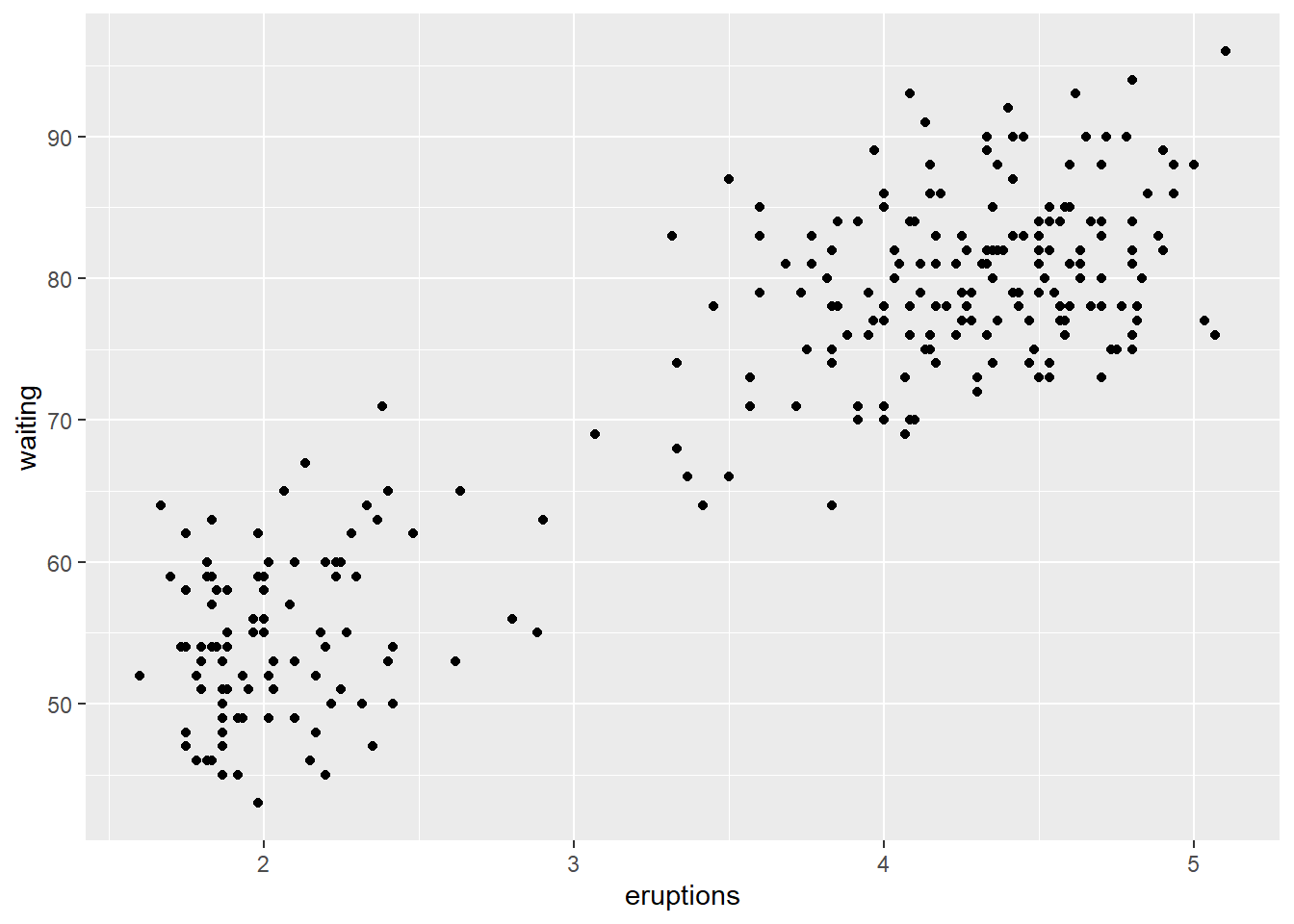• The annotate() function can be used to add any type of geometric object. In the example below, we add text to a plot.
# we are adding another layers to the p object
# Where do the values 3, 48, 4.5, and 66 come from?
p +
annotate("text", x = 3, y = 48, label = "Group 1") +
annotate("text", x = 4.5, y = 66, label = "Group 2")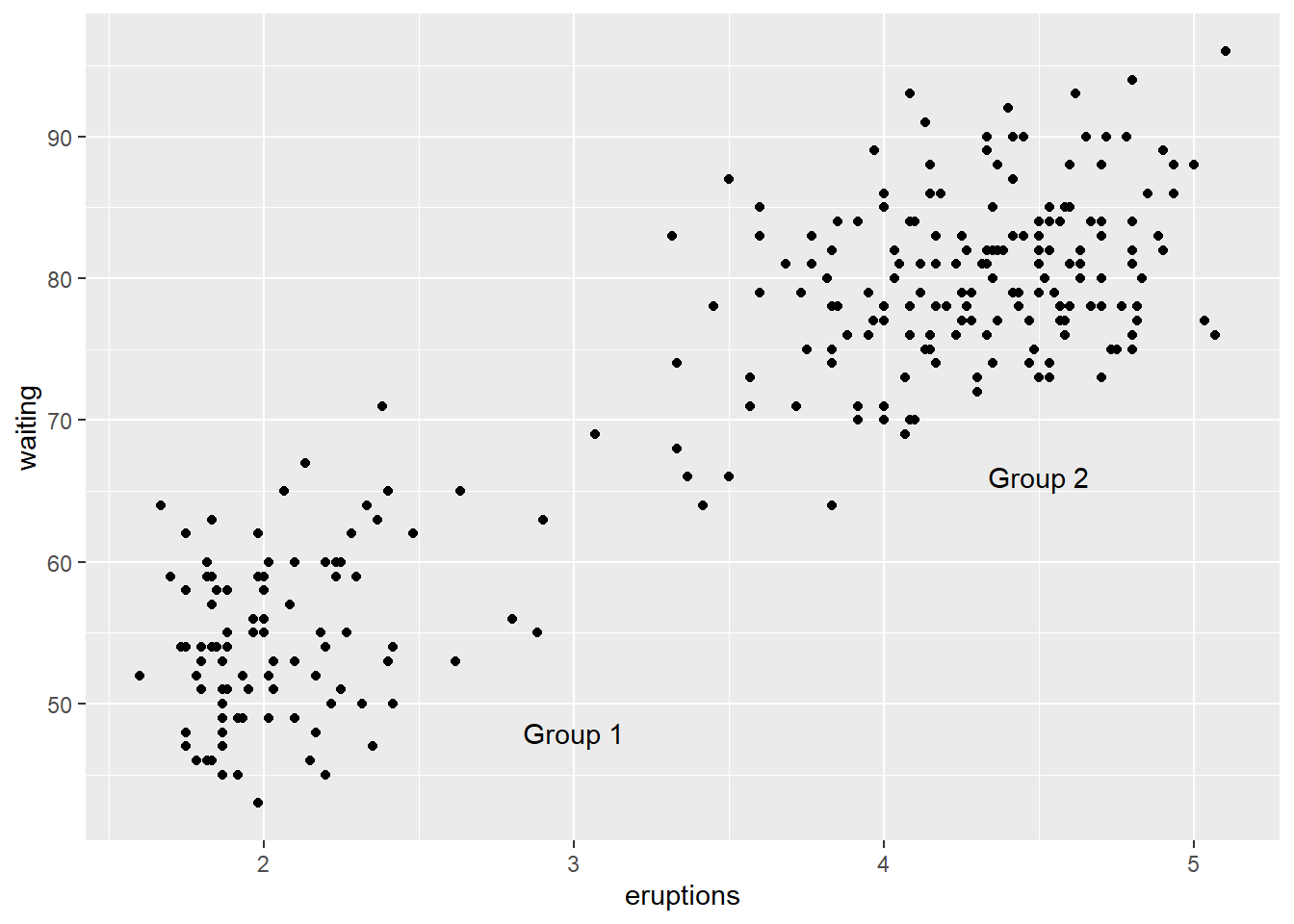# load the gcookbook package onto the memory
library(gcookbook)
## Warning: package 'gcookbook' was built under R version 4.0.3
• heightweight data
# How do you get the information of the heighweight data?
head(heightweight)
##   sex ageYear ageMonth heightIn weightLb
## 1   f   11.92      143     56.3     85.0
## 2   f   12.92      155     62.3    105.0
## 3   f   12.75      153     63.3    108.0
## 4   f   13.42      161     59.0     92.0
## 5   f   15.92      191     62.5    112.5
## 6   f   14.25      171     62.5    112.0
# How do you read colour = sex in the code below?
# Explain the role of colour = sex
hw_plot <- ggplot(heightweight, aes(x = ageYear, y = heightIn, colour = sex)) +
geom_point()
hw_plot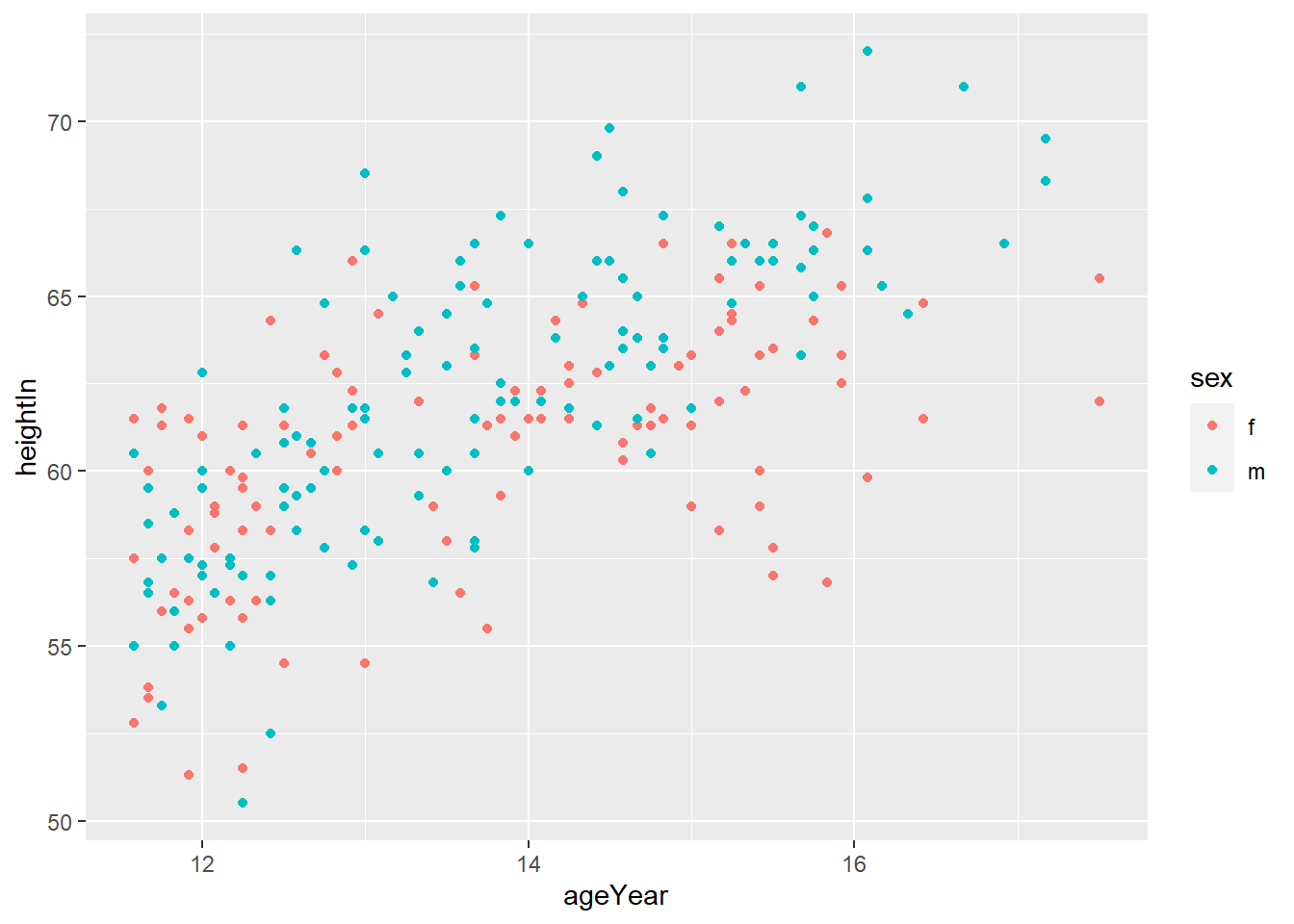• geom_hline(yintercept = y) adds horizontal line at y
# Add horizontal lines
# how do you get more detailed information about the geom_hline() function?
hw_plot +
geom_hline(yintercept = 60)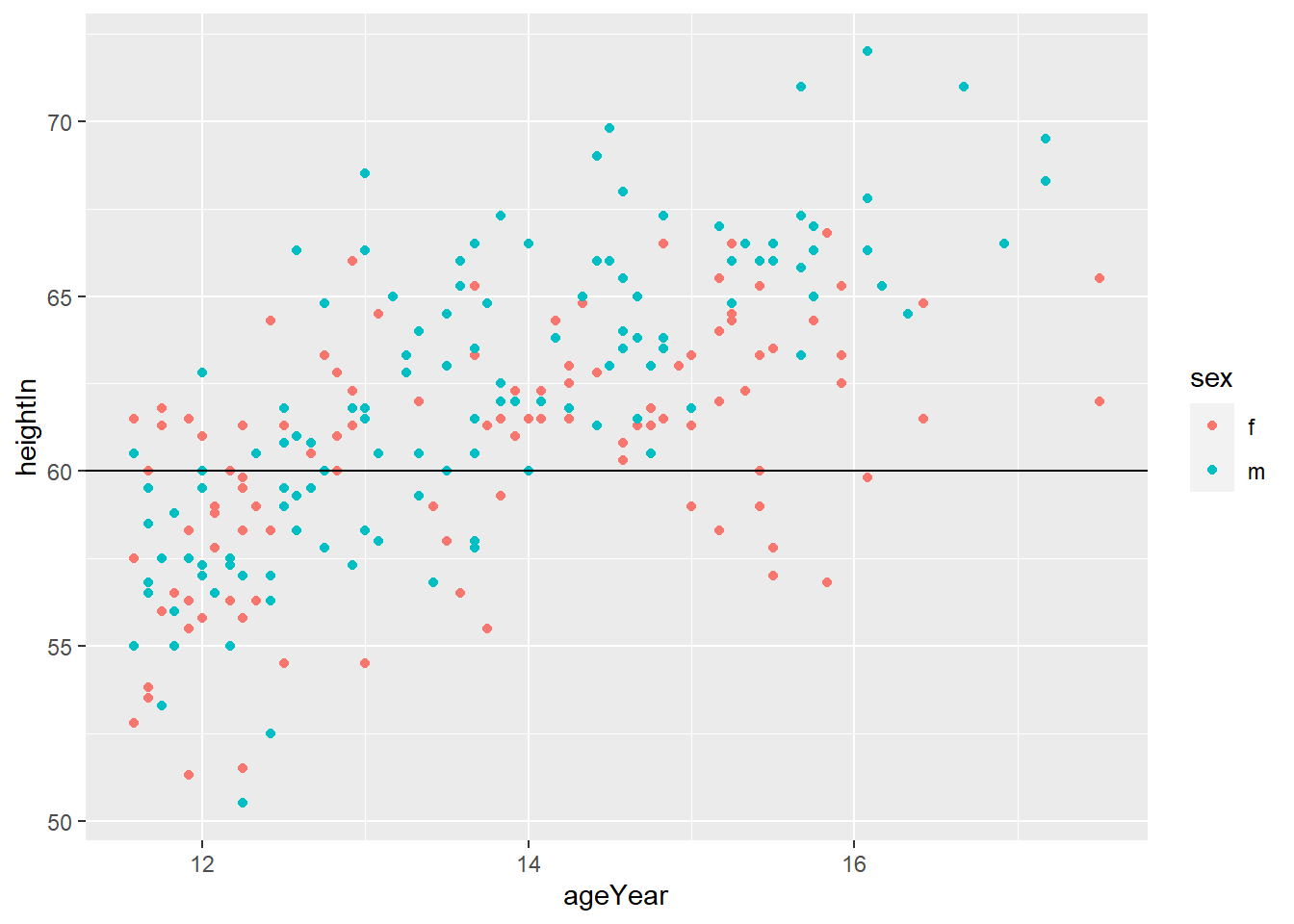• geom_vline(xintercept = x) adds horizontal line at x
# Add vertical lines
hw_plot +
geom_vline(xintercept = 14)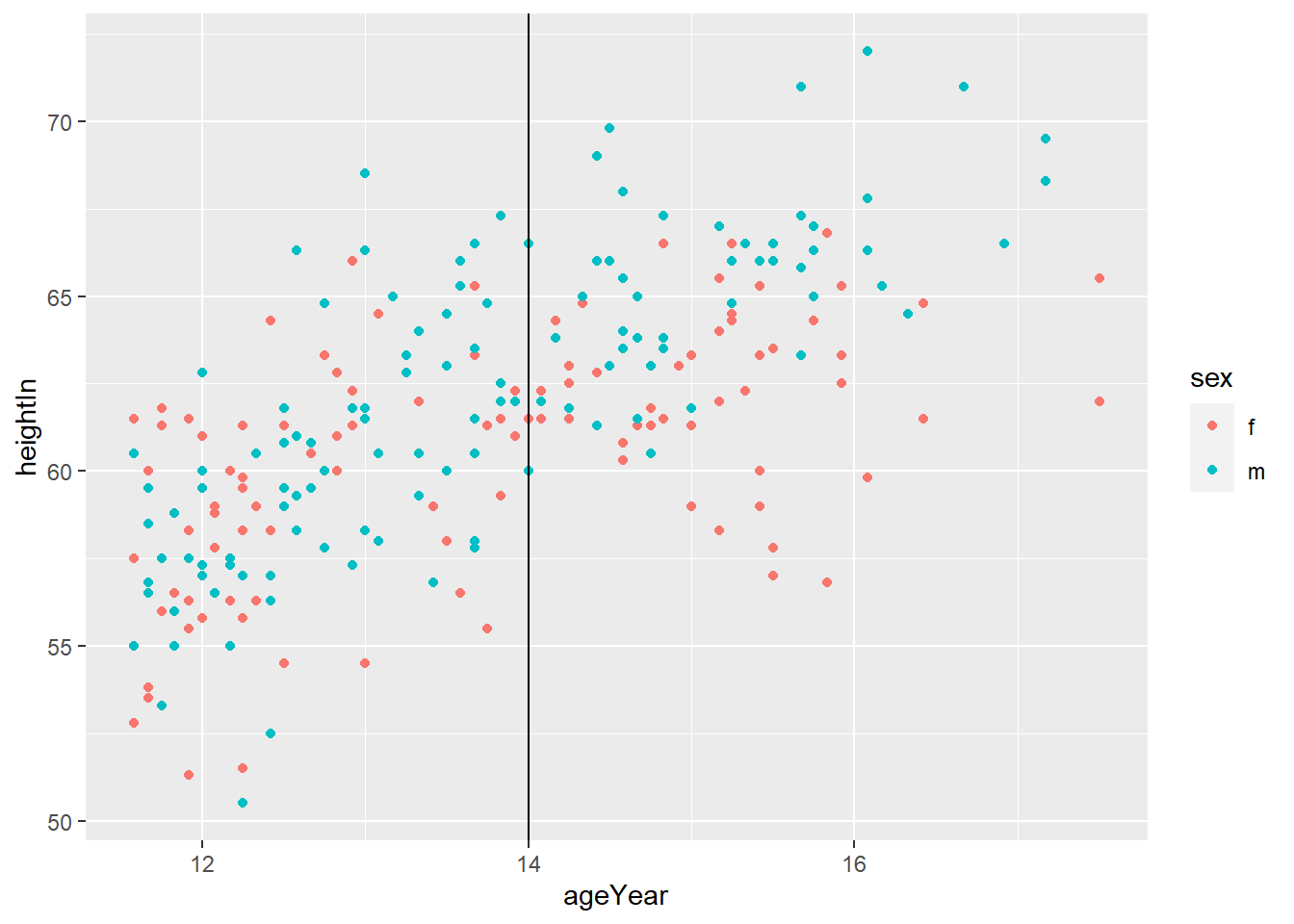• You can do both
# Add horizontal and vertical lines
hw_plot +
geom_hline(yintercept = 60) +
geom_vline(xintercept = 14)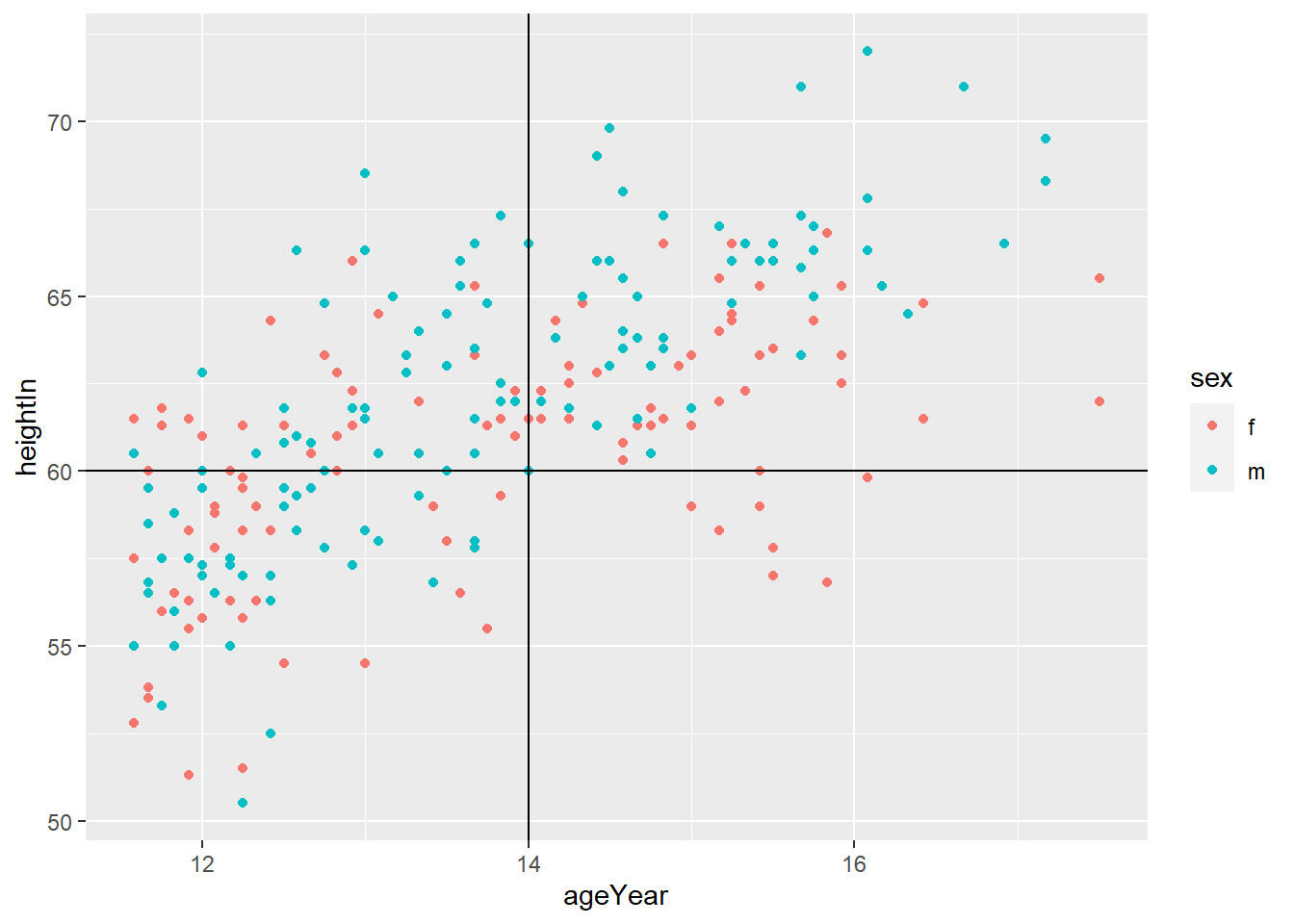• geom_abline(intercept = i, slope = s) adds horizontal line with y = i + s*x
hw_plot +
geom_abline(intercept = 37.4, slope = 1.75)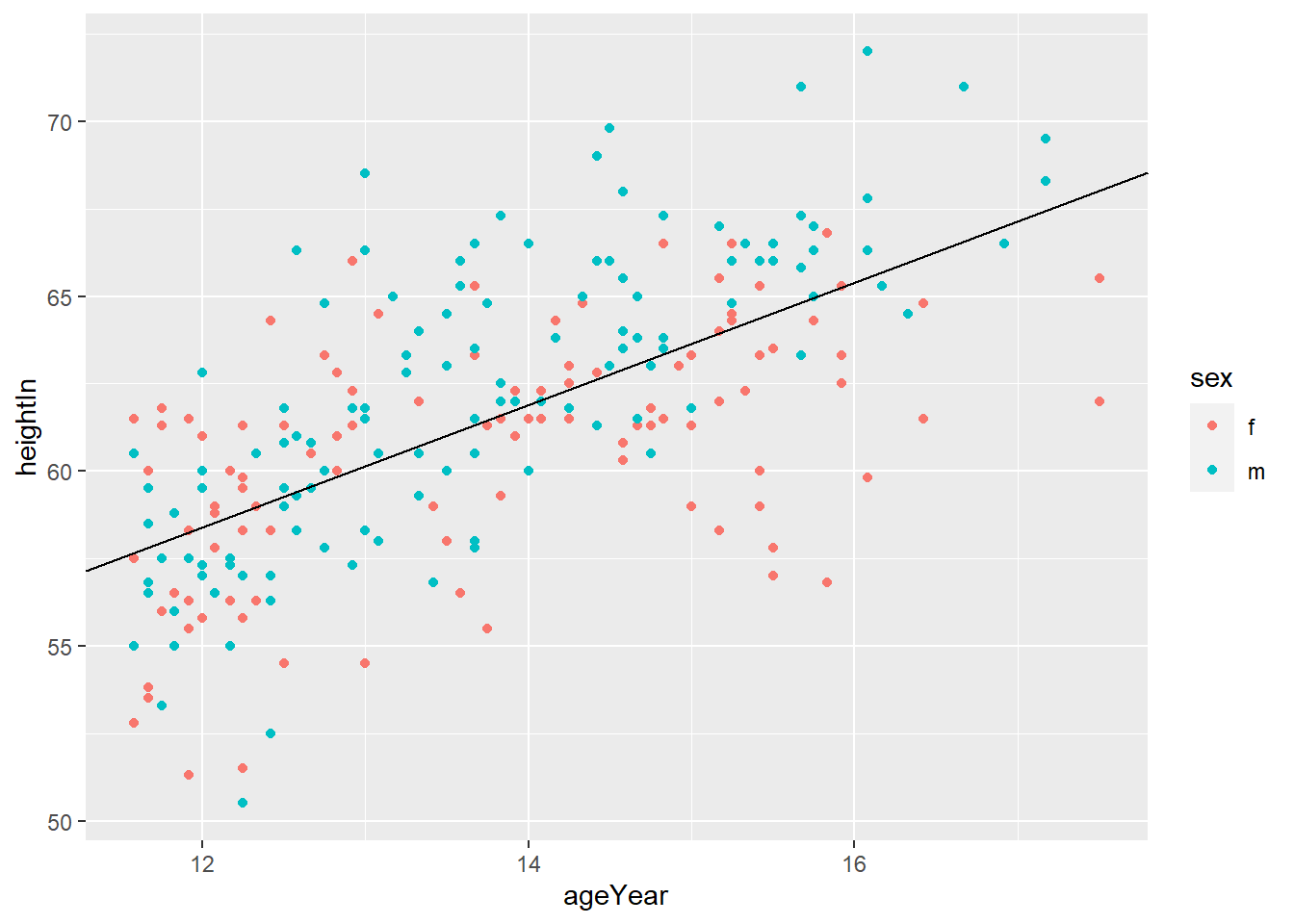## 5.8 Using Colors in Plots

### 5.8.1 Setting and Mapping the Colors of Objects

• It is important to distinguish
• setting aesthetics to a constant
• mapping aesthetics to a variable
• Setting aesthetics to a constant means you fix the value of aesthetics to a constant value.
# set the value of the color aesthetics to "blue"
ggplot(mpg, aes(x = displ, y = hwy)) +
geom_point(color = "blue")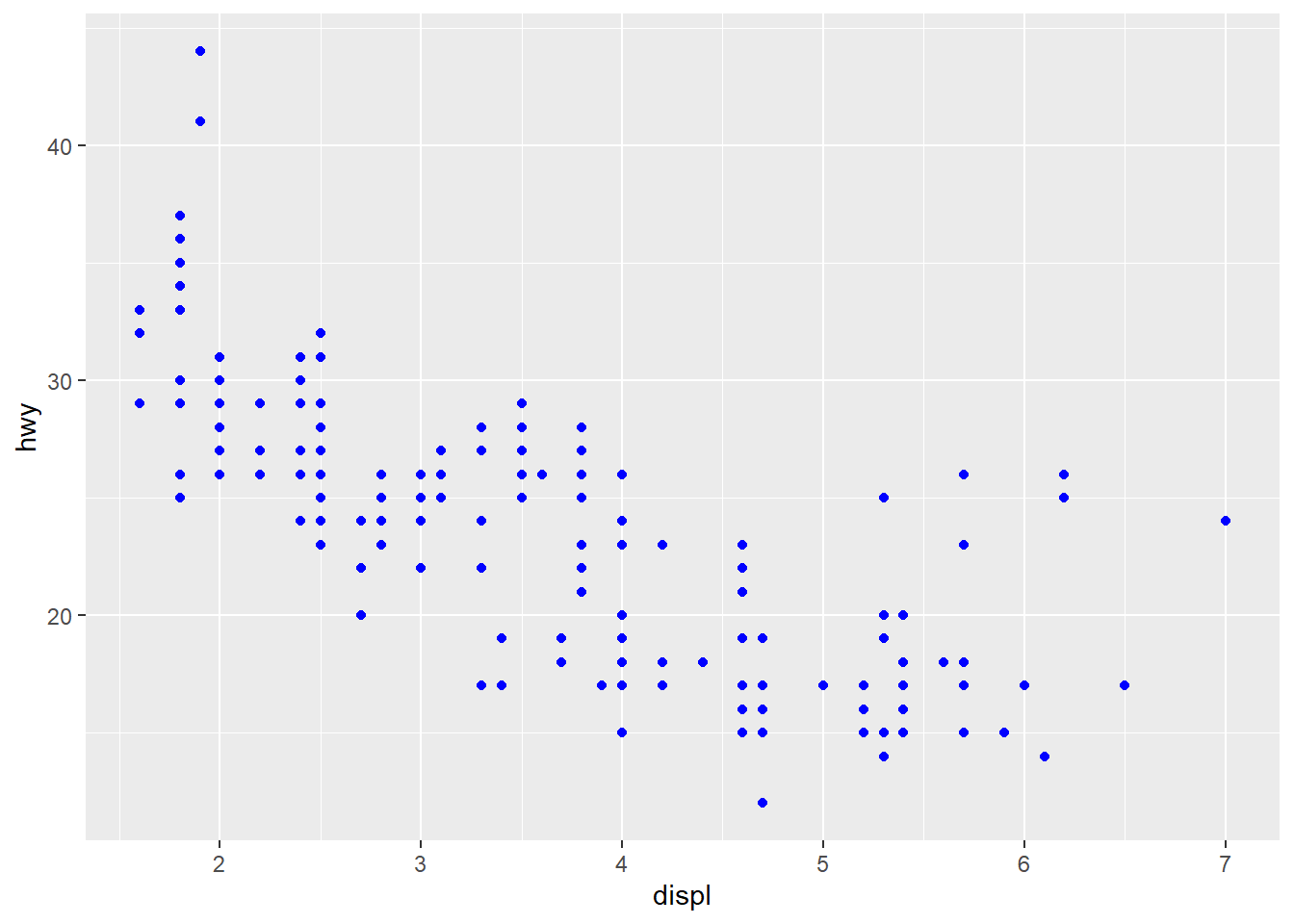# set the value of the color aesthetics to "red"
ggplot(mpg, aes(x = displ, y = hwy)) +
geom_point(color = "red")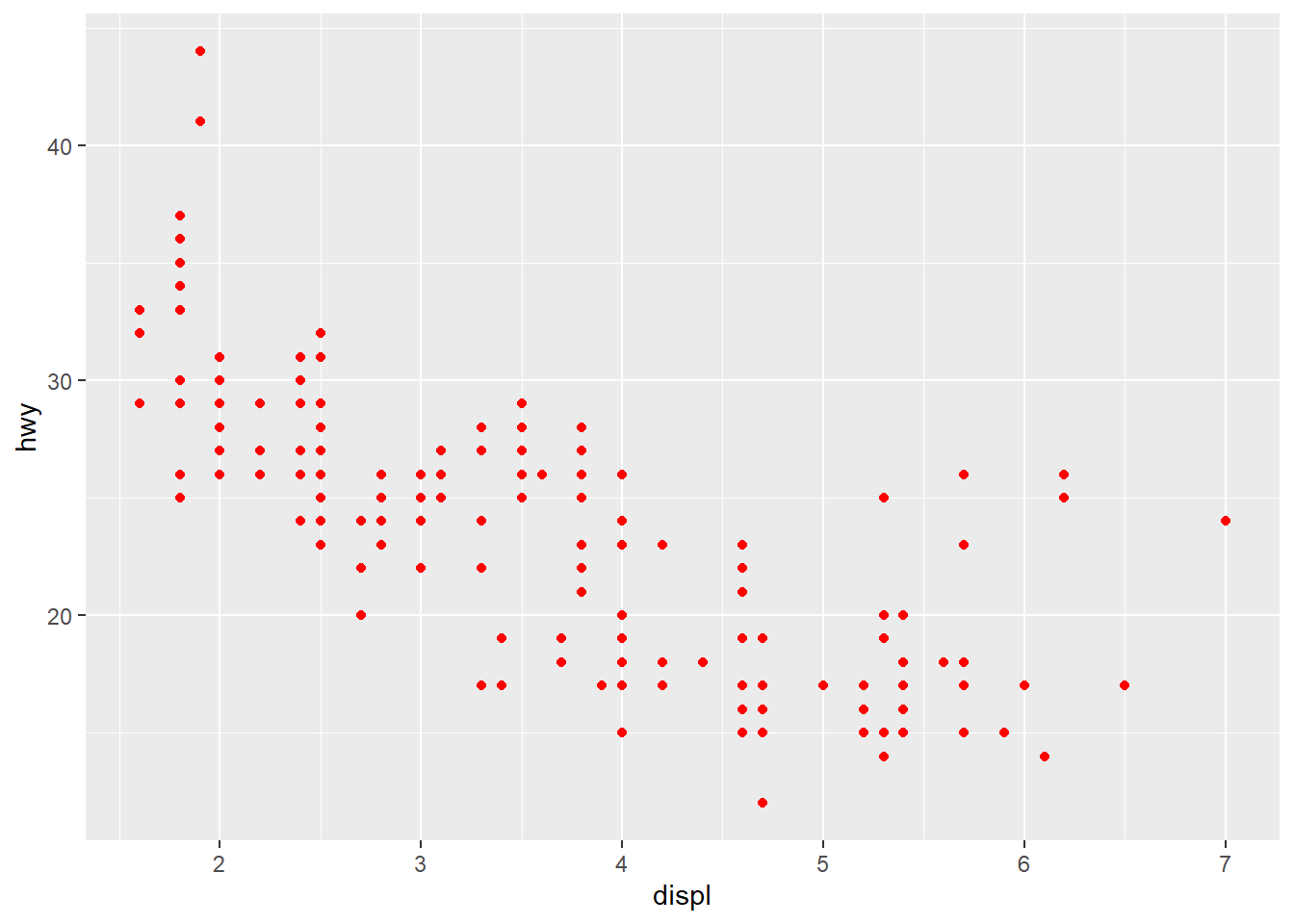• You can find many resources on the name of the color in R by typing “R color” in Google. Here is an example.

• Mapping aesthetics to a variable means you want to use different colors depending on the value of the variable.

# map the value of the color aesthetics to the variable drv
# This is called an aesthetic mapping, and you need aes()
ggplot(mpg, aes(x = displ, y = hwy)) +
geom_point(aes(color = drv))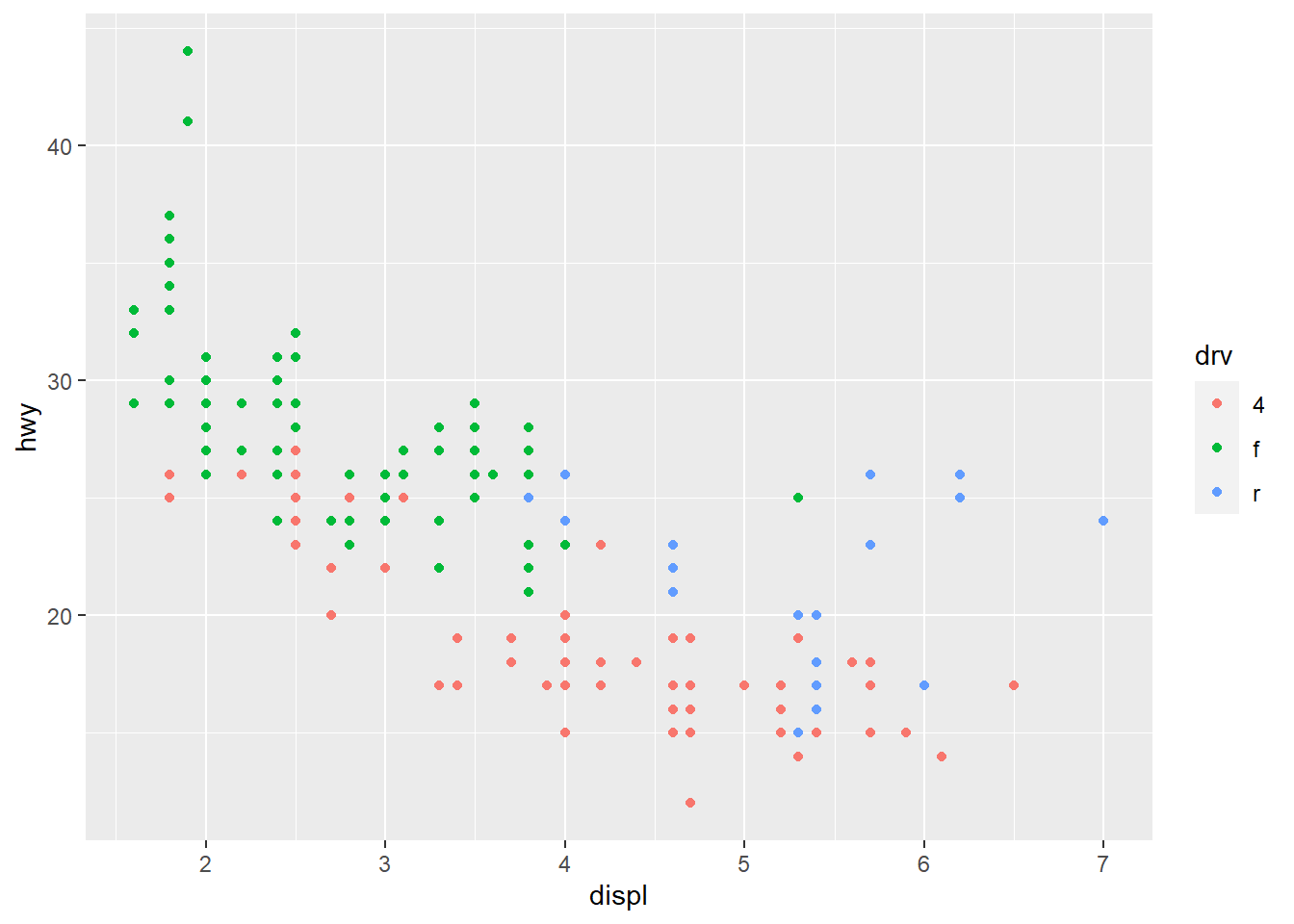### 5.8.2 Using a Different Palette for a Discrete Variable

• To use different color scheme, color palettes are available from the RColorBrewer package.
# load RColorBrewer package to a memory
library(RColorBrewer)
# display.brewer.all() generates available palette
display.brewer.all()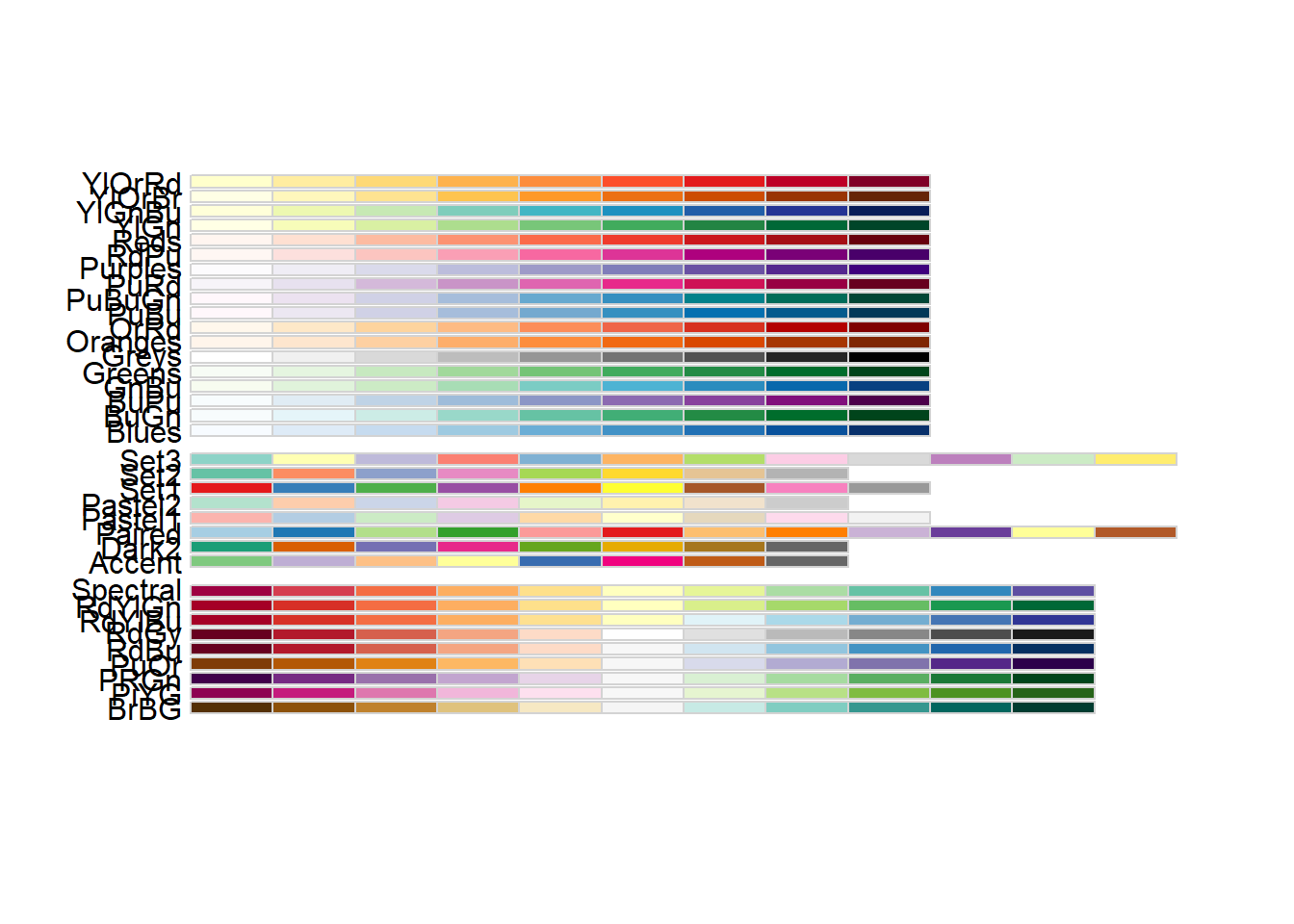library(gcookbook)
hw_splot <- ggplot(heightweight, aes(x = ageYear, y = heightIn, colour = sex)) +
geom_point()
hw_splot# "Oranges" palette
hw_splot +
scale_colour_brewer(palette = "Oranges")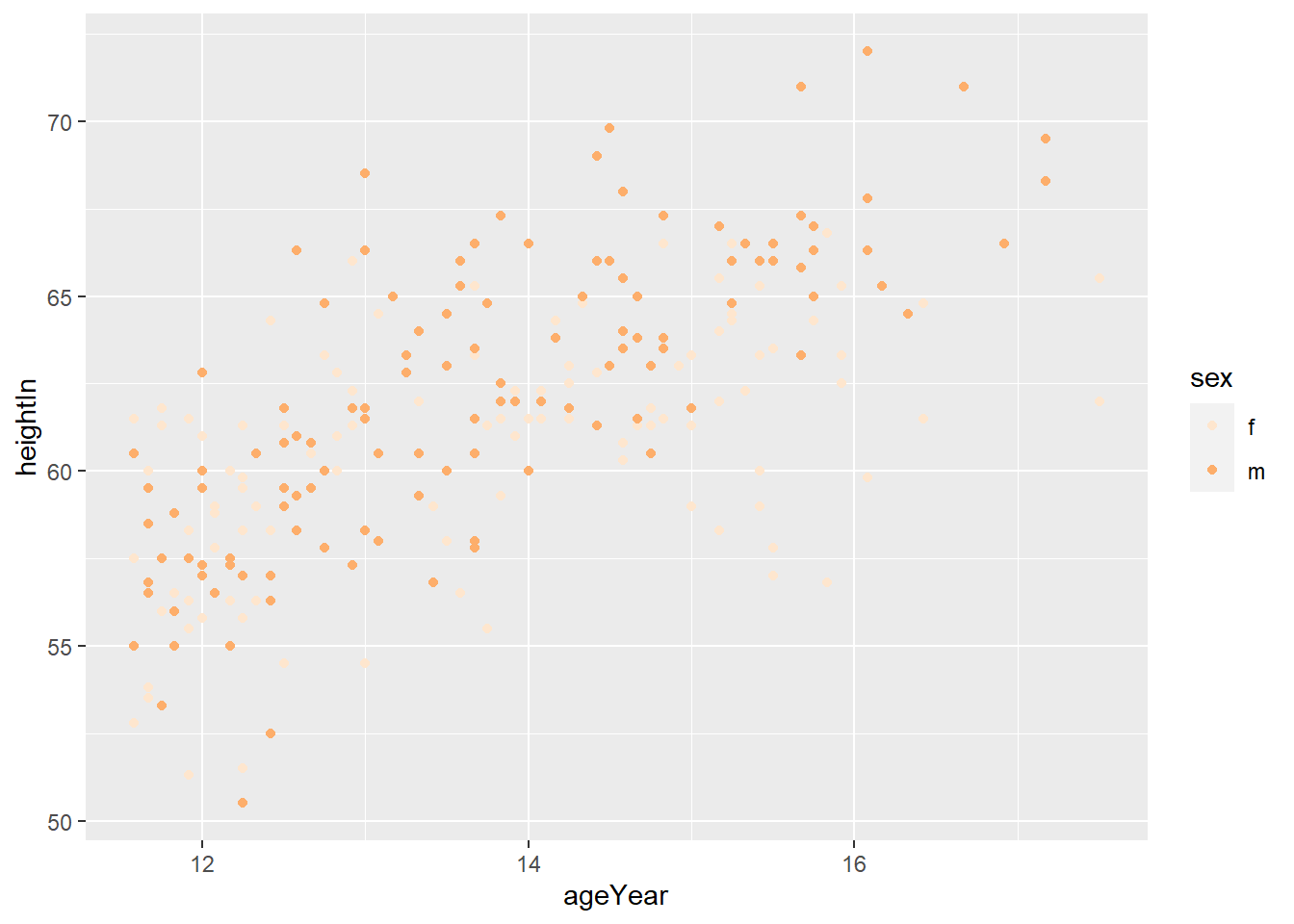# "Set3" palette
hw_splot +
scale_colour_brewer(palette = "Set3")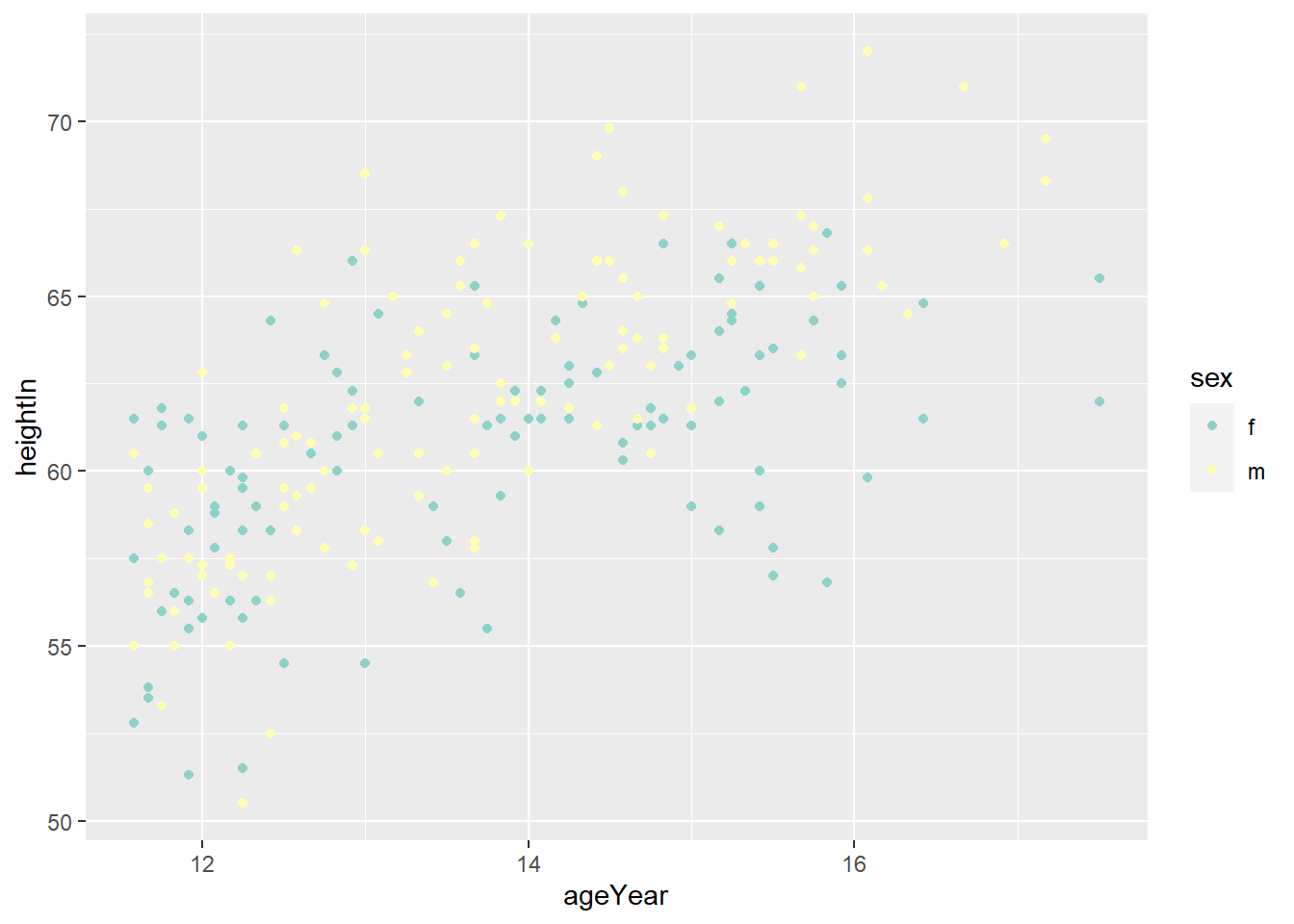### 5.8.3 Using a Manually Defined Palette for a Discrete Variable

• scale_colour_manual() sets the values of color
hw_splot +
scale_colour_manual(values = c("red", "blue"))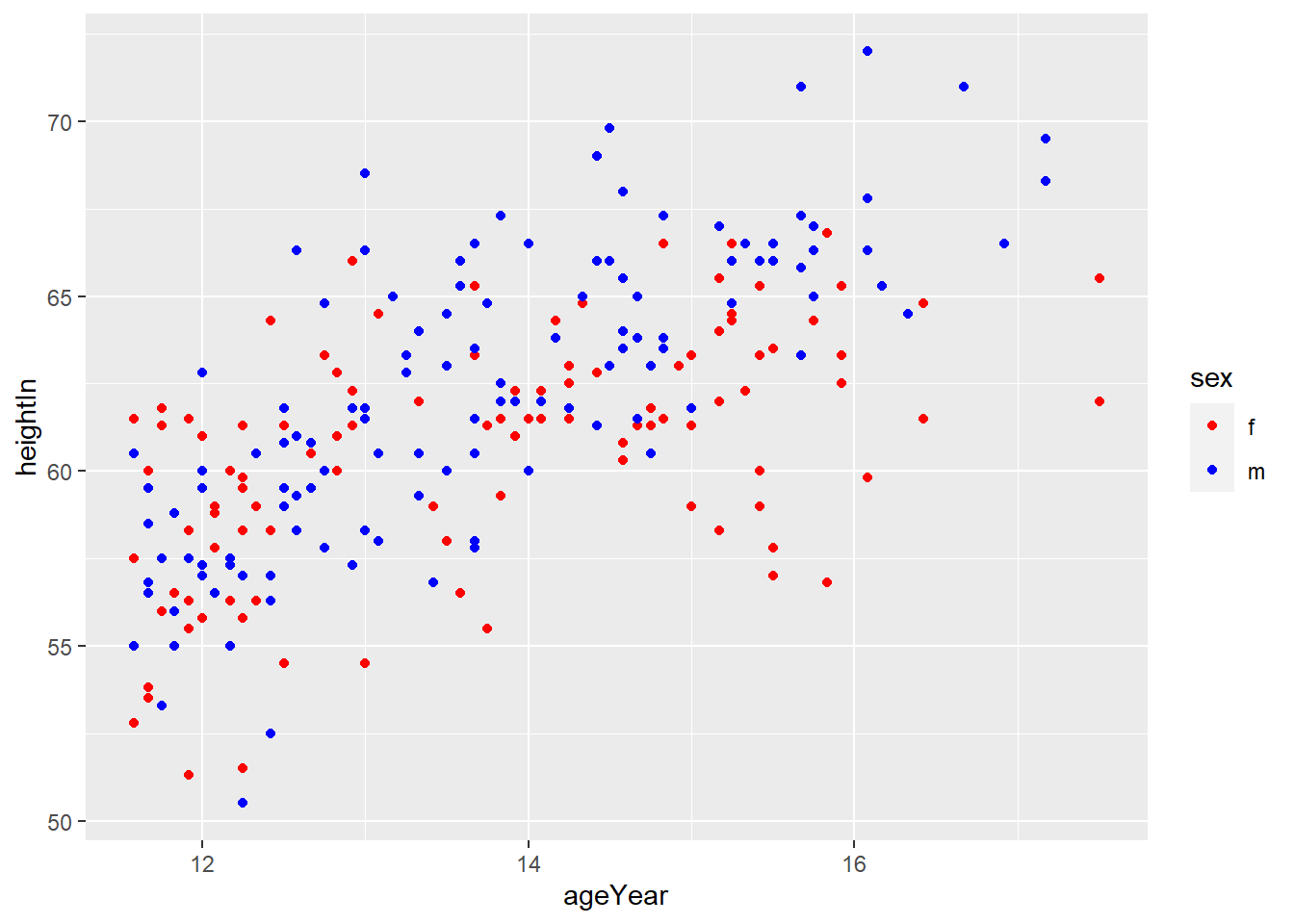### 5.8.4 Using a Manuallly Defined Palette for a Continuous Variable

hw_plot <- ggplot(heightweight, aes(x = ageYear, y = heightIn, colour = weightLb)) +
geom_point(size = 3)
hw_plot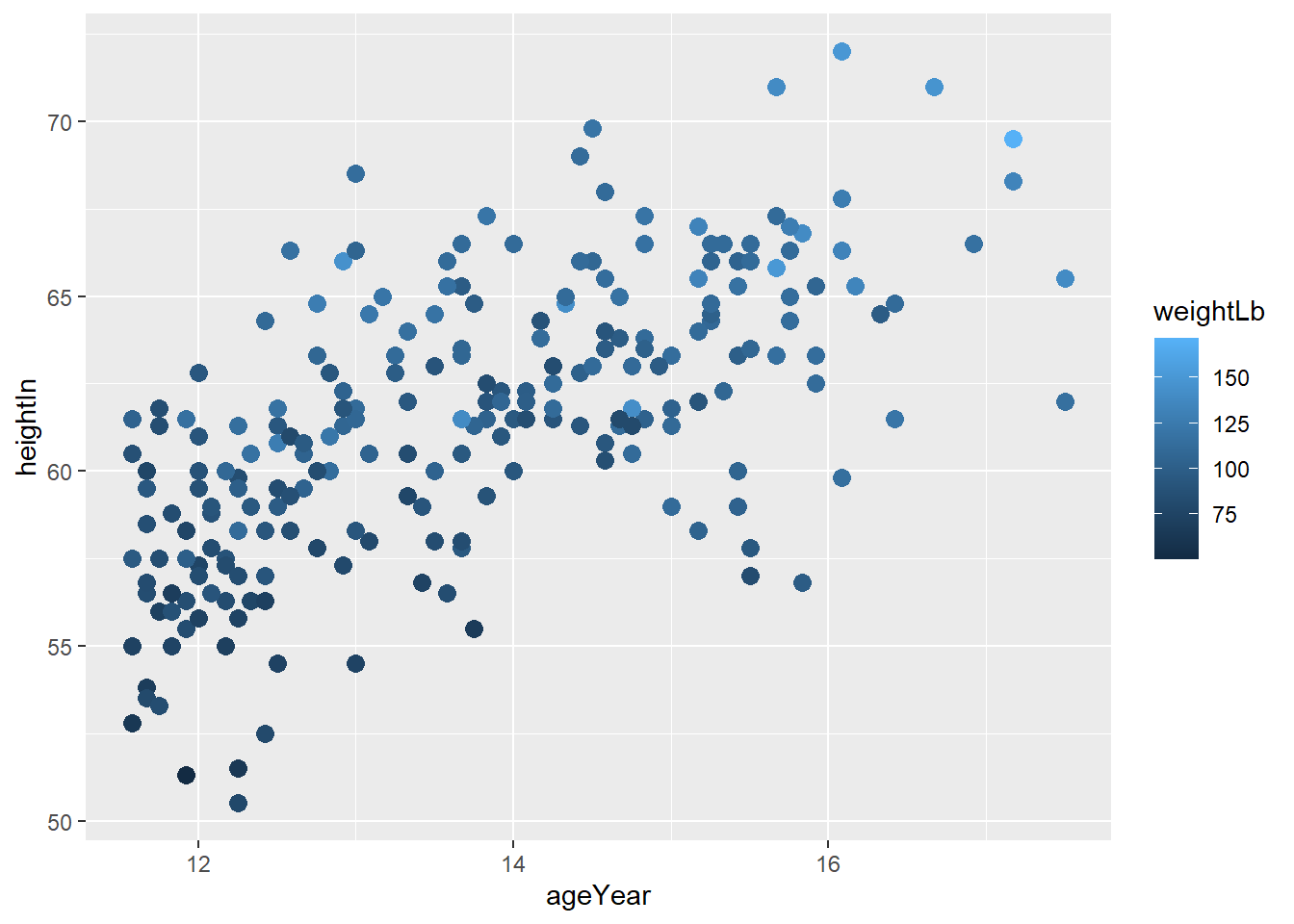• scale_colour_gradient() sets the low and high values of a color gradient
hw_plot +
scale_colour_gradient(low = "yellow", high = "orange")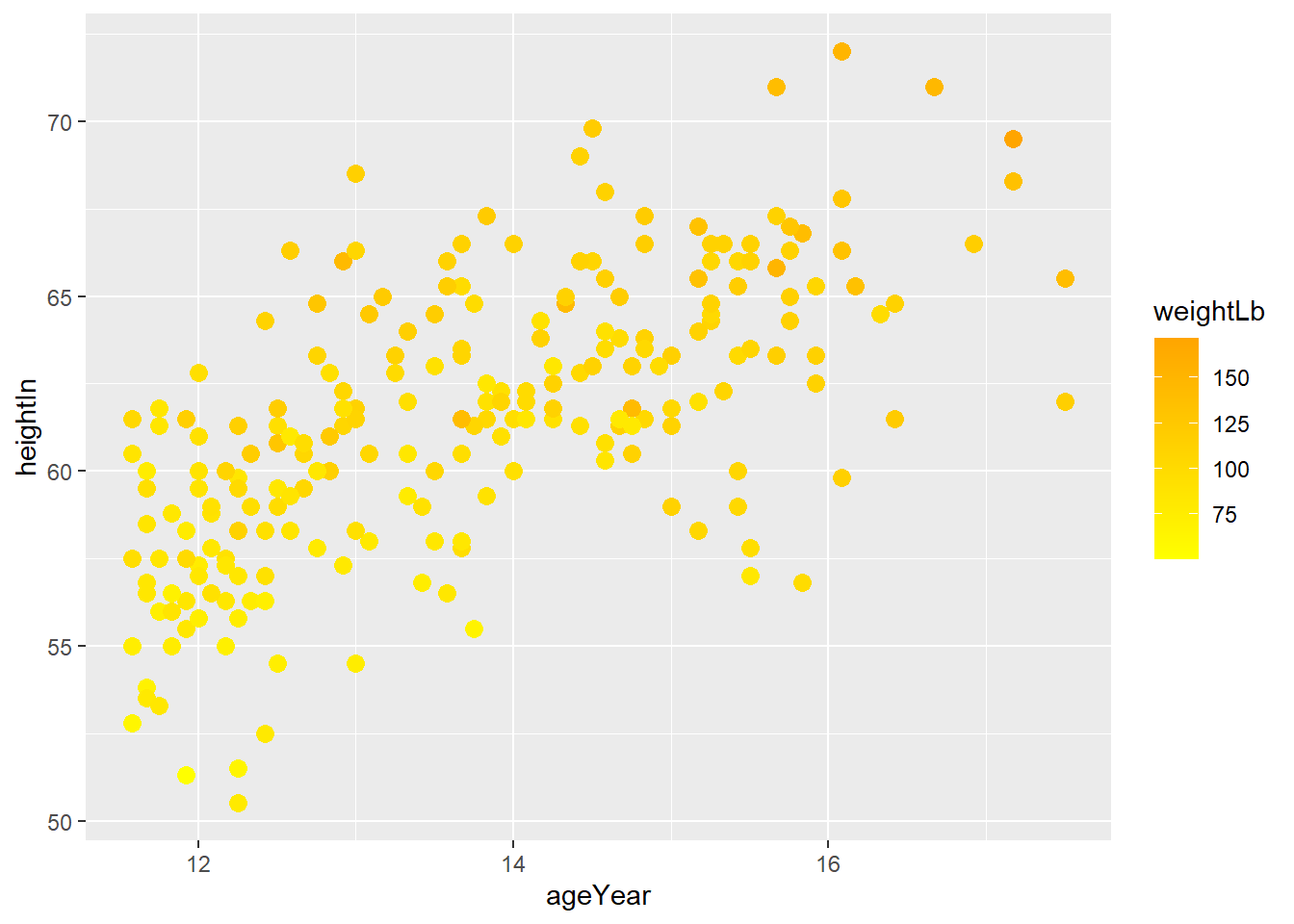## 5.9 Complete themes

• There are complete themes which control all non-data display at once.
ggplot(data = mpg, aes(x = class, fill = manufacturer)) +
geom_bar() +
labs(title = "Counts of Car Class",
subtitle = "By manufacturer",
caption = "source: mpg data from ggplot2",
fill = "Car Company",
x = "Class of Cars",
y = "Count")# theme_grey() is the defualt theme
ggplot(data = mpg, aes(x = class, fill = manufacturer)) +
geom_bar() +
labs(title = "Counts of Car Class",
subtitle = "By manufacturer",
caption = "source: mpg data from ggplot2",
fill = "Car Company",
x = "Class of Cars",
y = "Count") +
theme_grey()# theme_grey() is the defualt theme
ggplot(data = mpg, aes(x = class, fill = manufacturer)) +
geom_bar() +
labs(title = "Counts of Car Class",
subtitle = "By manufacturer",
caption = "source: mpg data from ggplot2",
fill = "Car Company",
x = "Class of Cars",
y = "Count") +
theme_classic()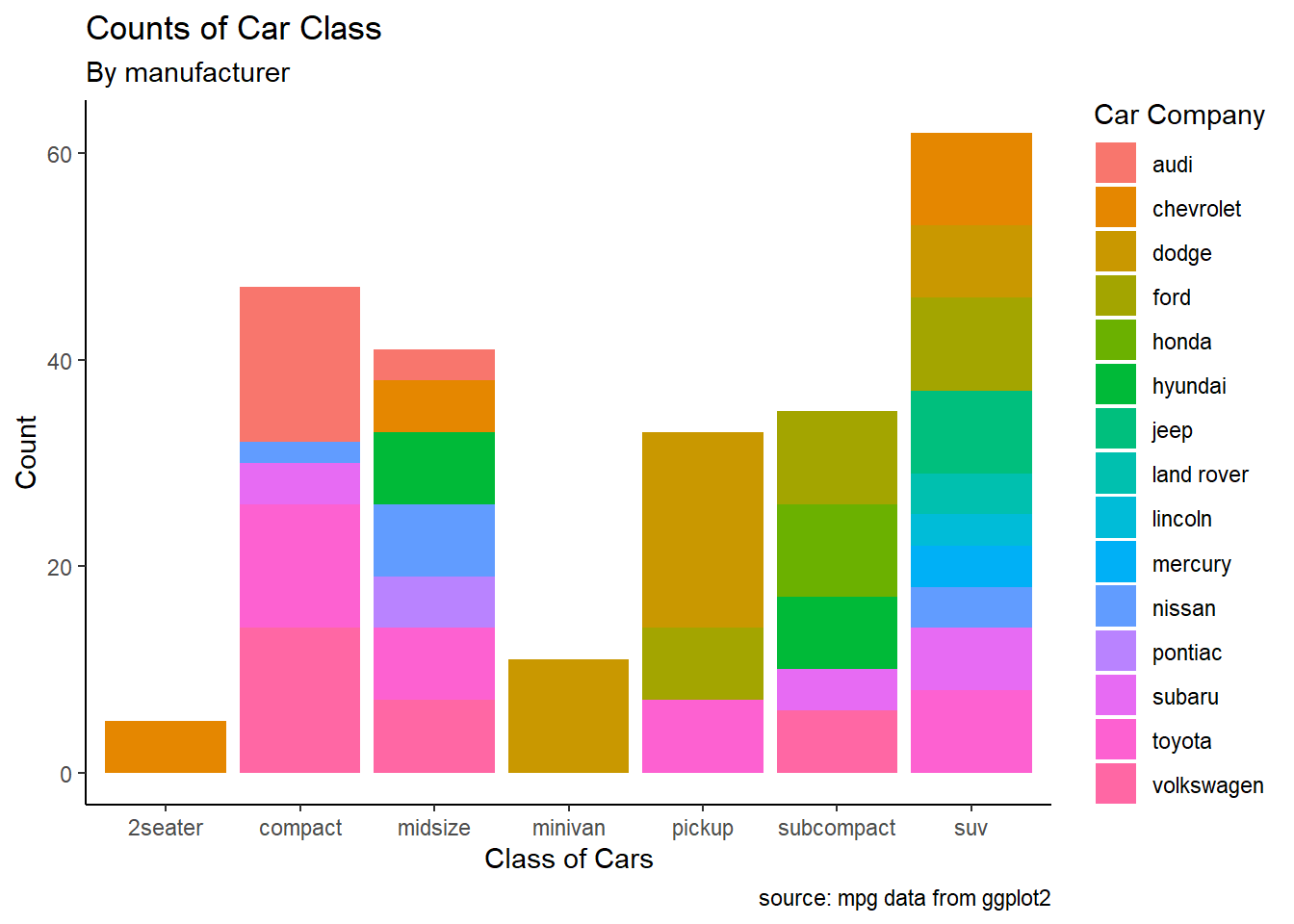# theme_grey() is the defualt theme
ggplot(data = mpg, aes(x = class, fill = manufacturer)) +
geom_bar() +
labs(title = "Counts of Car Class",
subtitle = "By manufacturer",
caption = "source: mpg data from ggplot2",
fill = "Car Company",
x = "Class of Cars",
y = "Count") +
theme_light()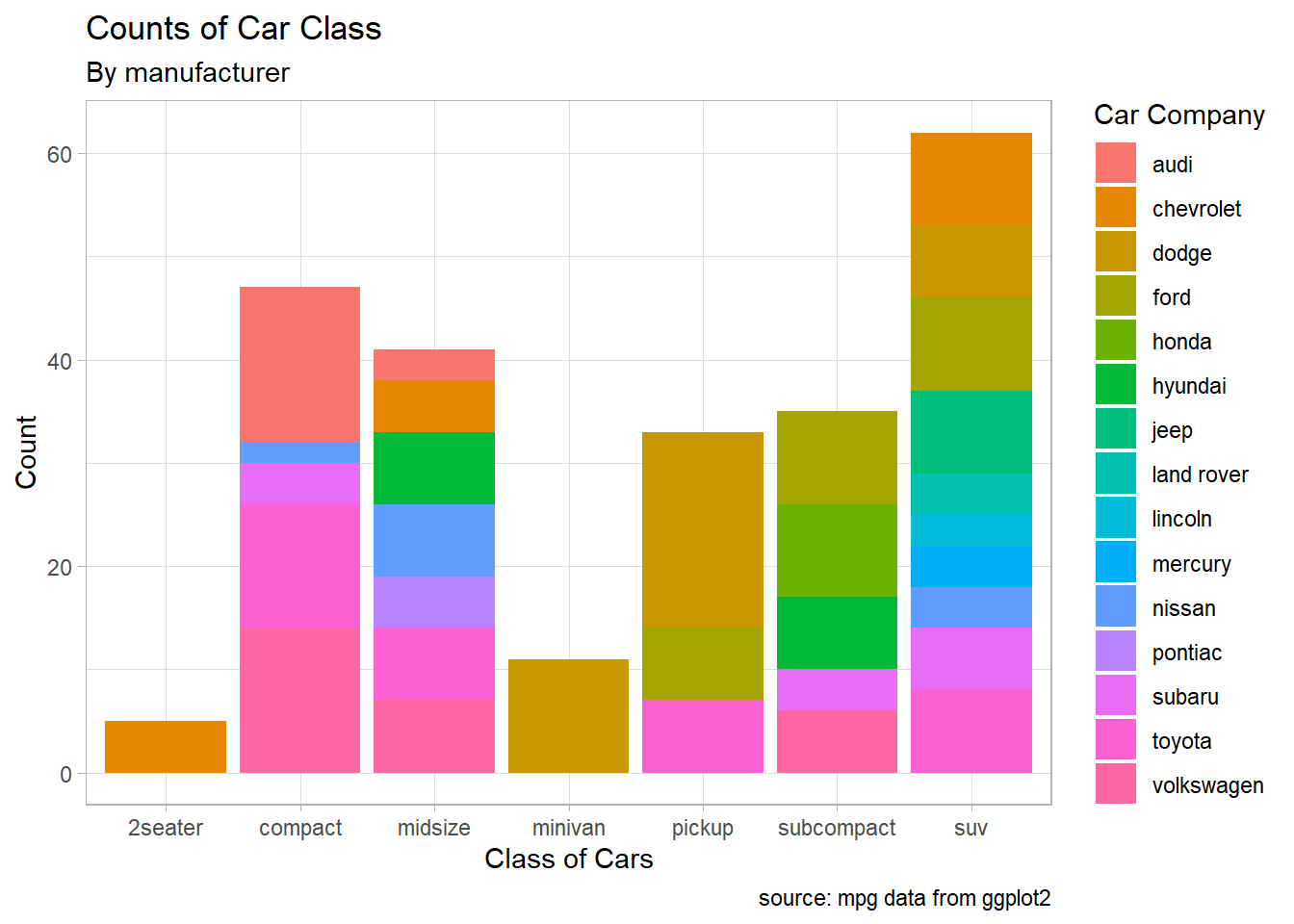• Check here for more details about the complete themes.

## 5.10 Exercise

### 5.10.1 Exercise 1 (with answers)

• Using the heightweight dataset in the gcookbook package, replicate the following plots
# Height and weight of school children
# head() displays the first six observations
head(heightweight)
##   sex ageYear ageMonth heightIn weightLb
## 1   f   11.92      143     56.3     85.0
## 2   f   12.92      155     62.3    105.0
## 3   f   12.75      153     63.3    108.0
## 4   f   13.42      161     59.0     92.0
## 5   f   15.92      191     62.5    112.5
## 6   f   14.25      171     62.5    112.0
ggplot(heightweight, aes(x = ageYear, y = heightIn, size = weightLb, color = sex)) +
geom_point()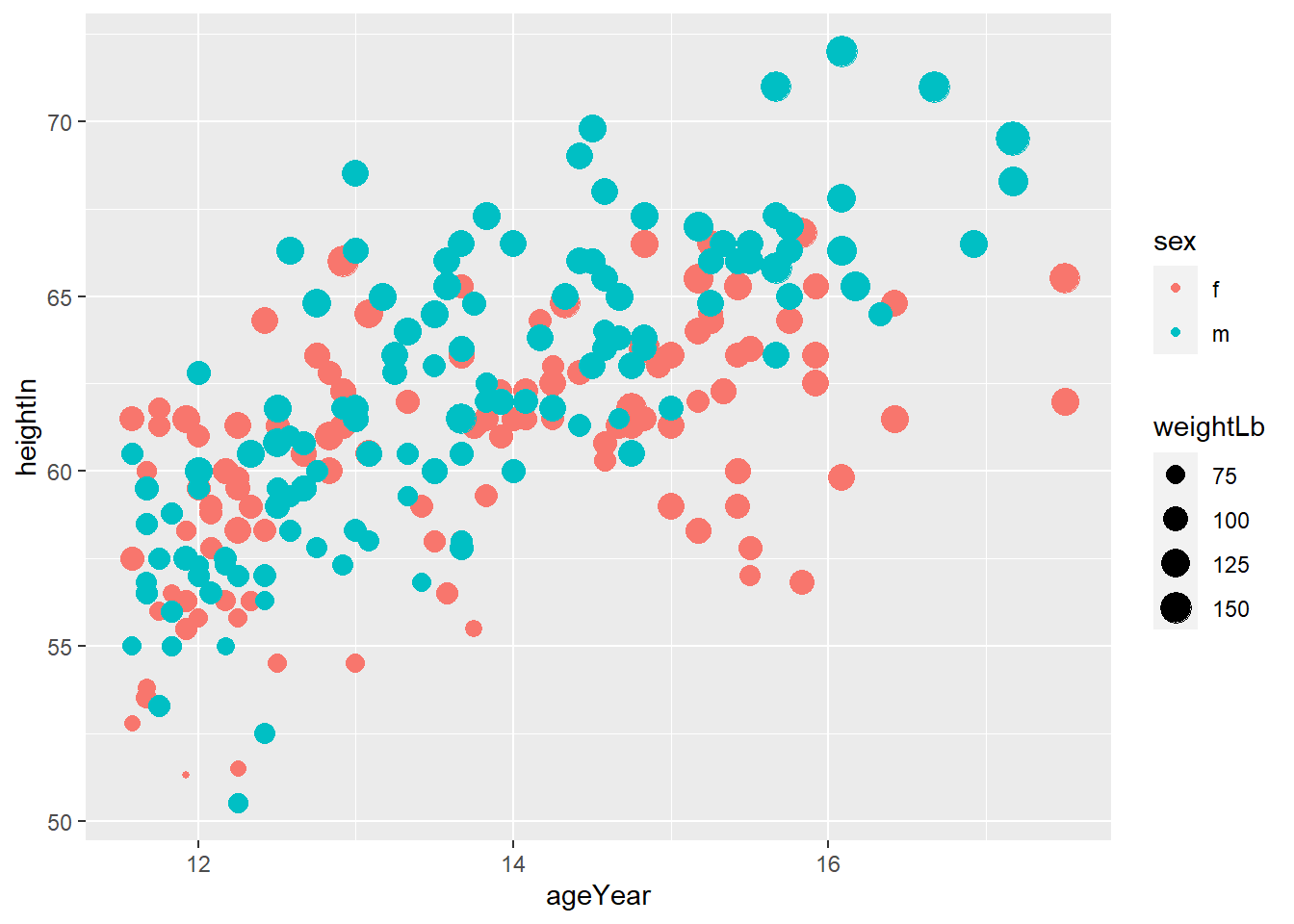• Often we want to set (or map) the transparency of points, especially when points overlaps. In that case, alpha controls the transparency of points. In this exercise, use alpha = 0.3.
ggplot(heightweight, aes(x = ageYear, y = heightIn, size = weightLb, color = sex)) +
geom_point(alpha = 0.3)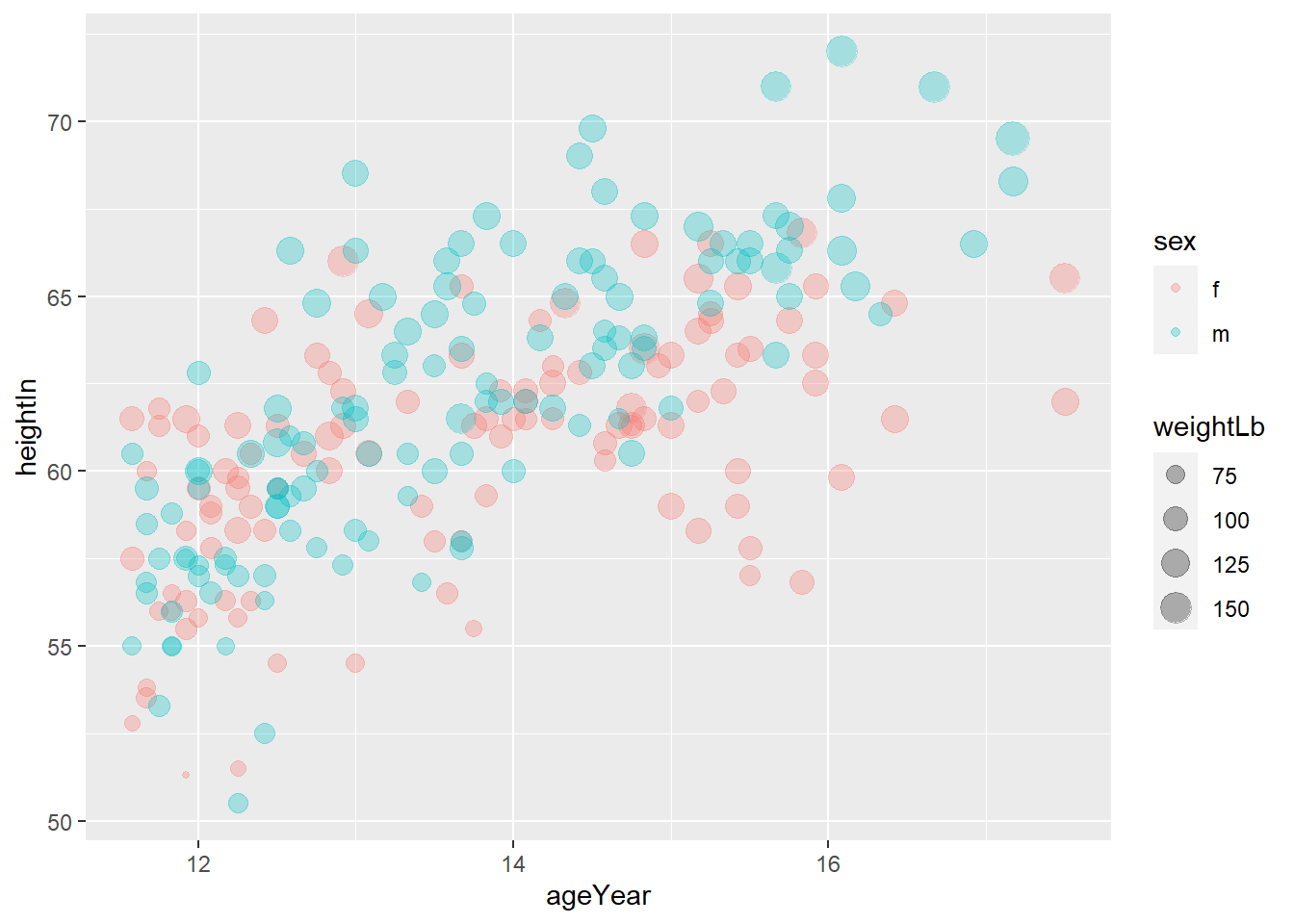• you can set the title, subtitle, x-axis label, y-axis label, and legend title using labs()
ggplot(heightweight, aes(x = ageYear, y = heightIn, size = weightLb, color = sex)) +
geom_point(alpha = 0.3) +
labs(title = "Height and weight of school children",
subtitle = "Height vs Weight",
caption = "Source: heightweight",
x = "Age (year)",
y = "Height (inches)",
size = "Weight (Lb)",
color = "Gender"
)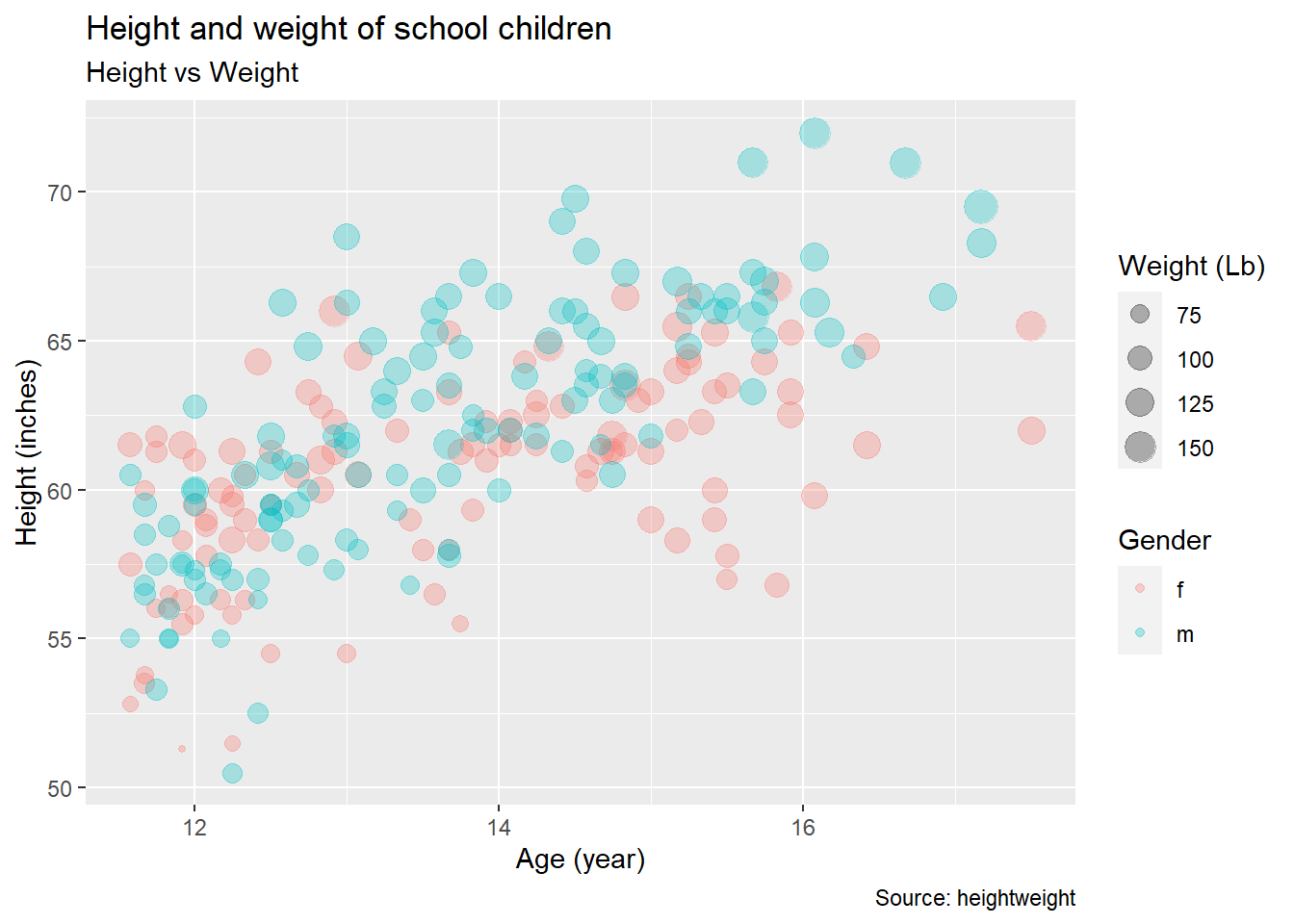• You may want to use themes. Use theme_classic().
ggplot(heightweight, aes(x = ageYear, y = heightIn, size = weightLb, color = sex)) +
geom_point(alpha = 0.3) +
labs(title = "Height and weight of school children",
subtitle = "Height vs Weight",
x = "Age (year)",
y = "Height (inches)",
size = "Weight (Lb)",
color = "Gender"
) +
theme_classic()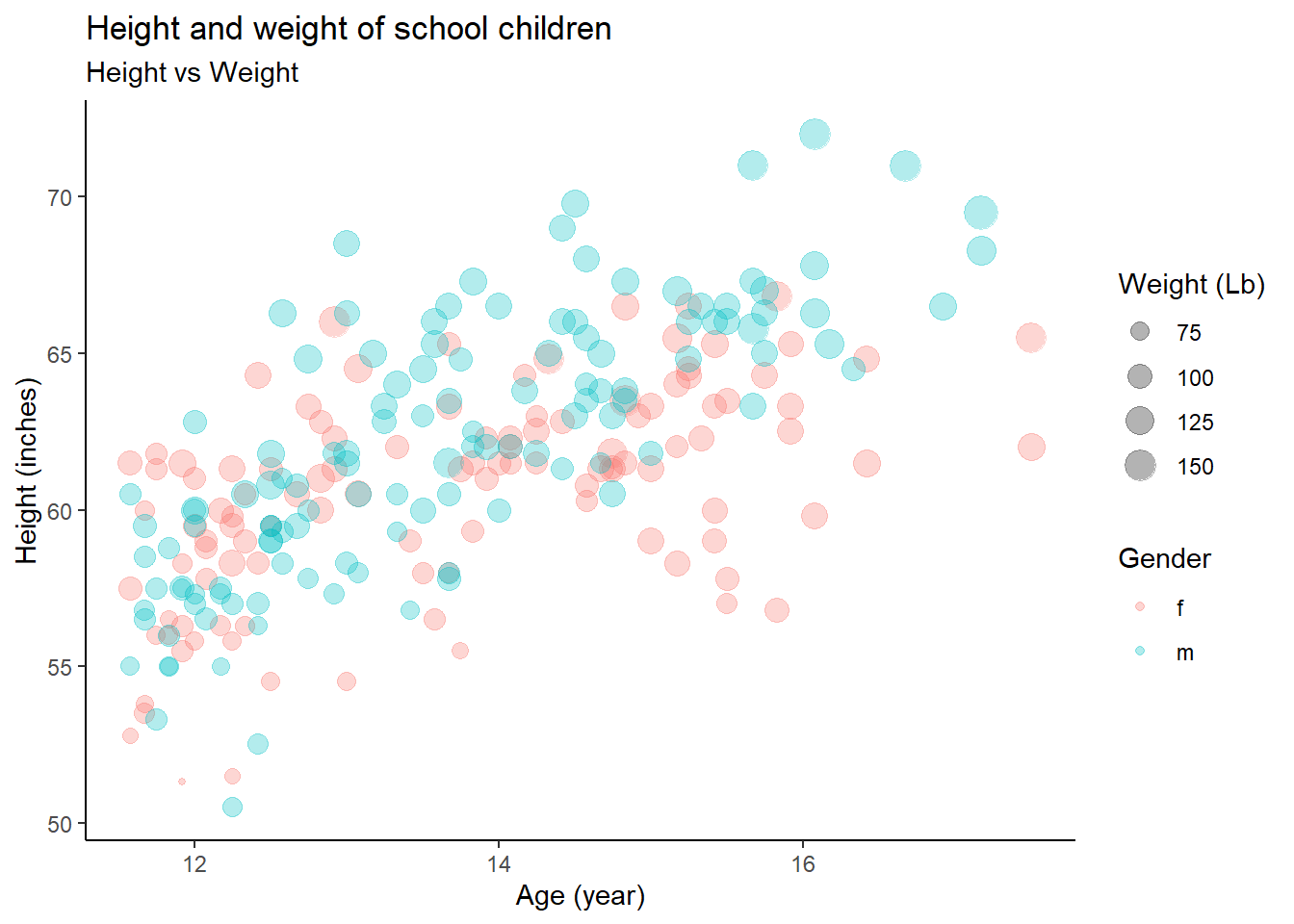### 5.10.2 Exercise 2 (with answers)

• Using the heightweight dataset in the gcookbook package, replicate the following plots
# Height and weight of school children
# head() displays the first six observations
head(heightweight)
##   sex ageYear ageMonth heightIn weightLb
## 1   f   11.92      143     56.3     85.0
## 2   f   12.92      155     62.3    105.0
## 3   f   12.75      153     63.3    108.0
## 4   f   13.42      161     59.0     92.0
## 5   f   15.92      191     62.5    112.5
## 6   f   14.25      171     62.5    112.0
• geom_histogram() displays a histogram to display the distribution of a variable.
ggplot(heightweight, aes(x = heightIn)) +
geom_histogram()
## stat_bin() using bins = 30. Pick better value with binwidth.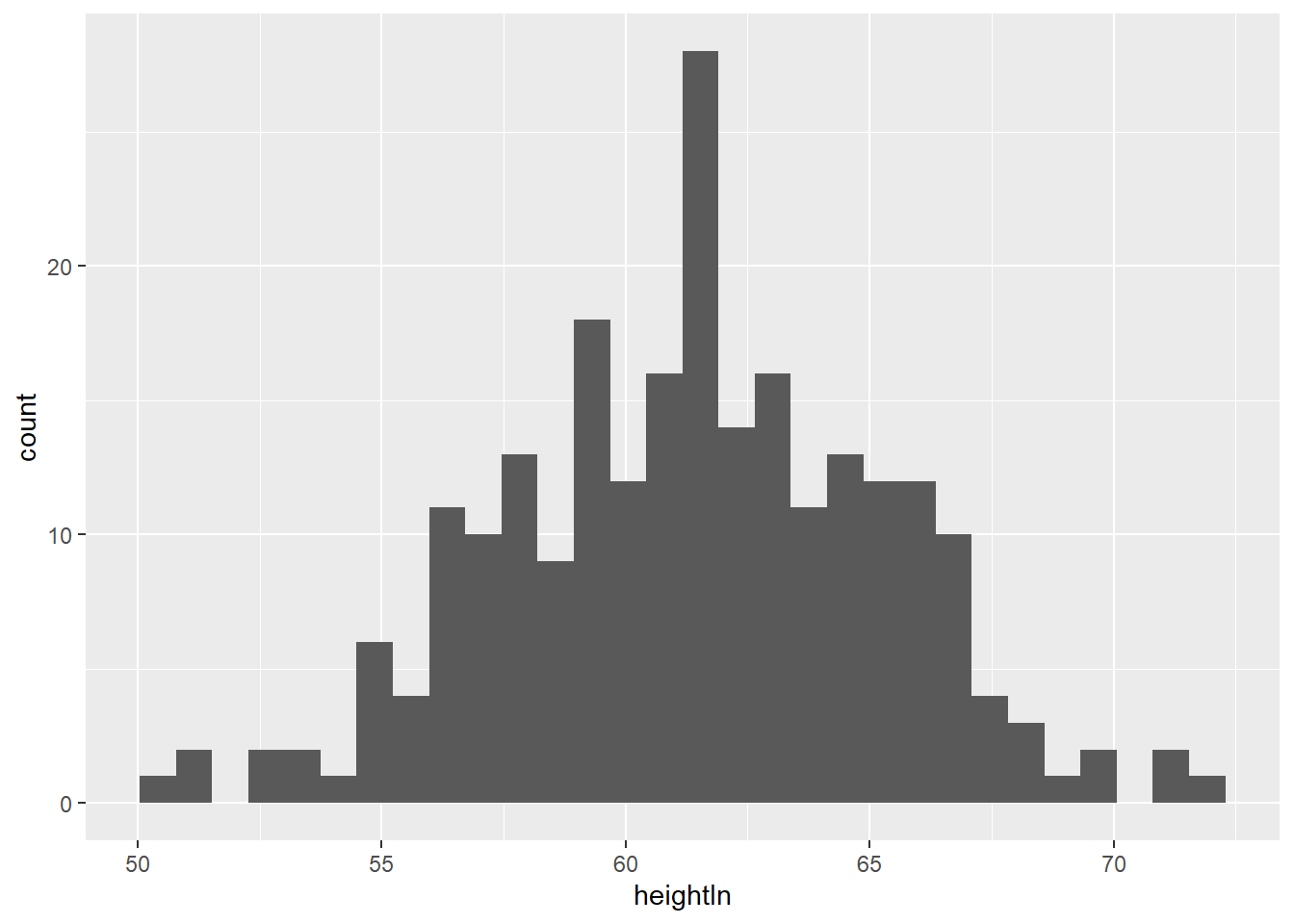• The fill aesthetics control the inside color of a geometric object.
ggplot(heightweight, aes(x = weightLb, fill = sex)) +
geom_histogram() 
## stat_bin() using bins = 30. Pick better value with binwidth.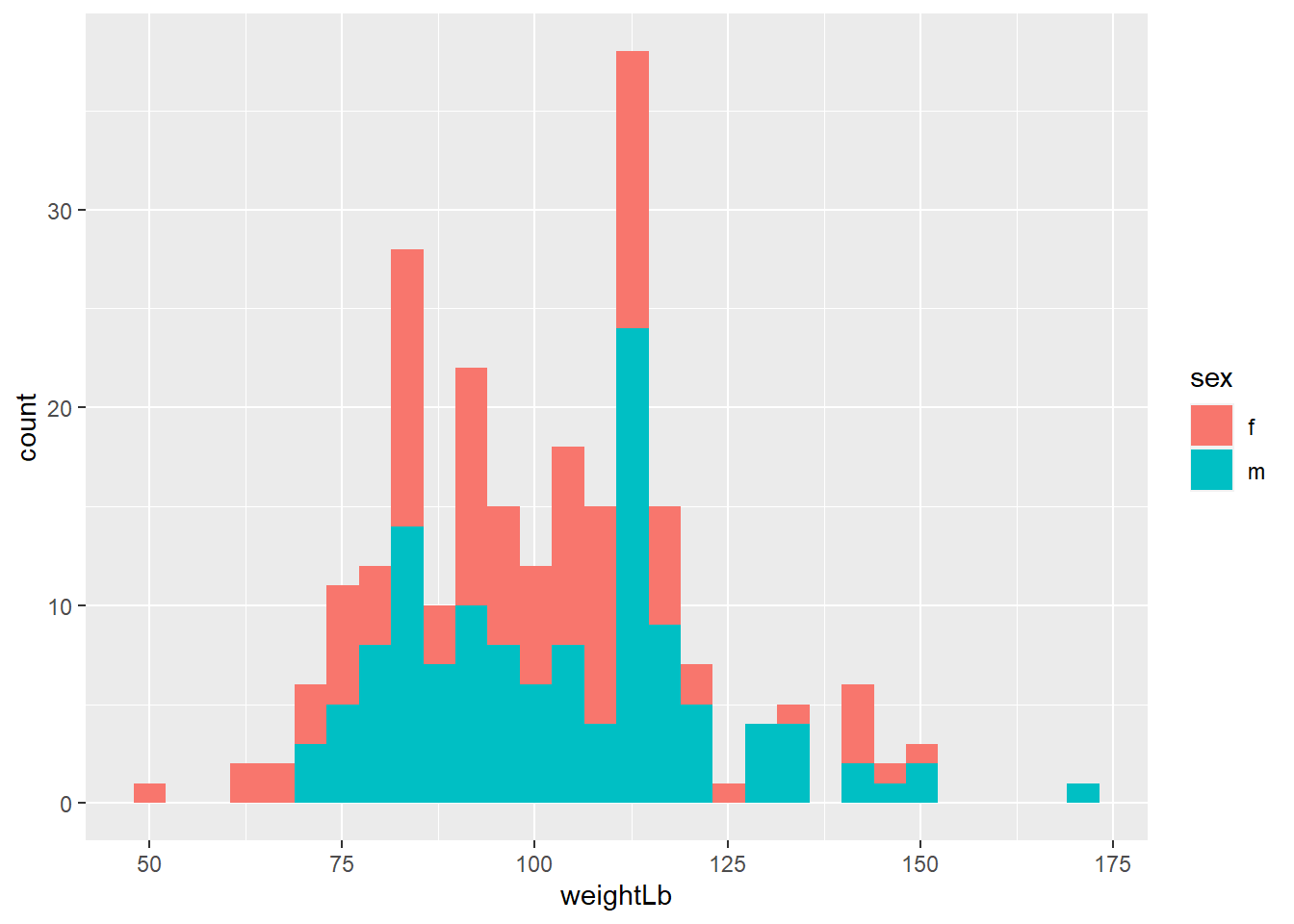• If you use color and fill aesthetics, you need scale_color_manual() and scale_fill_manual() to manually control the color and fill aesthetics. Depending on the aesthetic you used in 3-2-a, manually change the color of the female to orange and male to yellow.
ggplot(heightweight, aes(x = weightLb, fill = sex)) +
geom_histogram(alpha = 0.4) +
scale_fill_manual(values = c("orange", "yellow"))
## stat_bin() using bins = 30. Pick better value with binwidth.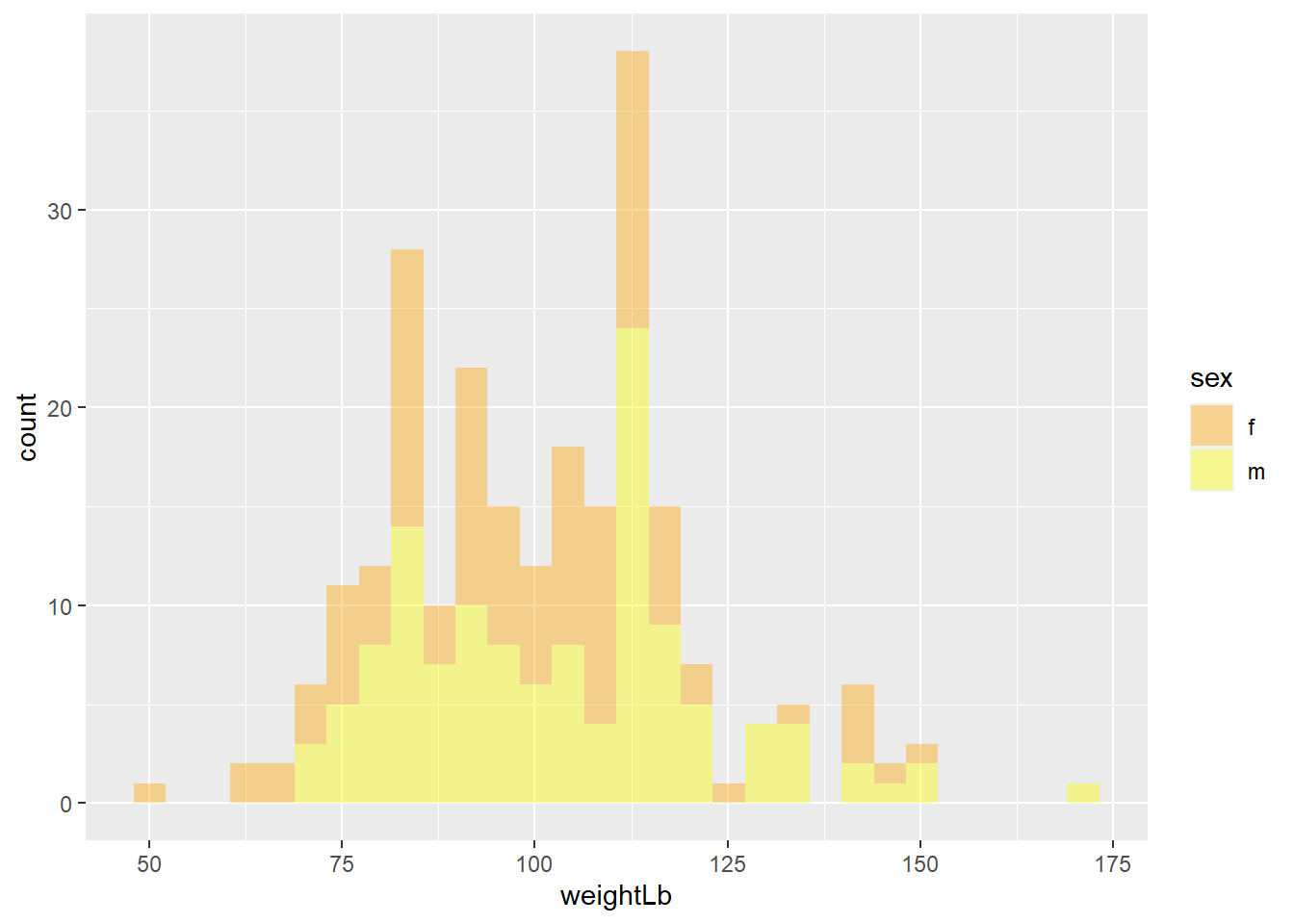• Again, add titles and apply theme_minimal()
ggplot(heightweight, aes(x = weightLb, fill = sex)) +
geom_histogram(alpha = 0.4) +
scale_fill_manual(values = c("orange", "yellow")) +
labs(title = "A histogram of the weight of school children",
subtitle = "By gender",
x = "Weight (Lb)",
y = "Count",
fill = "Gender"
) +
theme_minimal()
## stat_bin() using bins = 30. Pick better value with binwidth.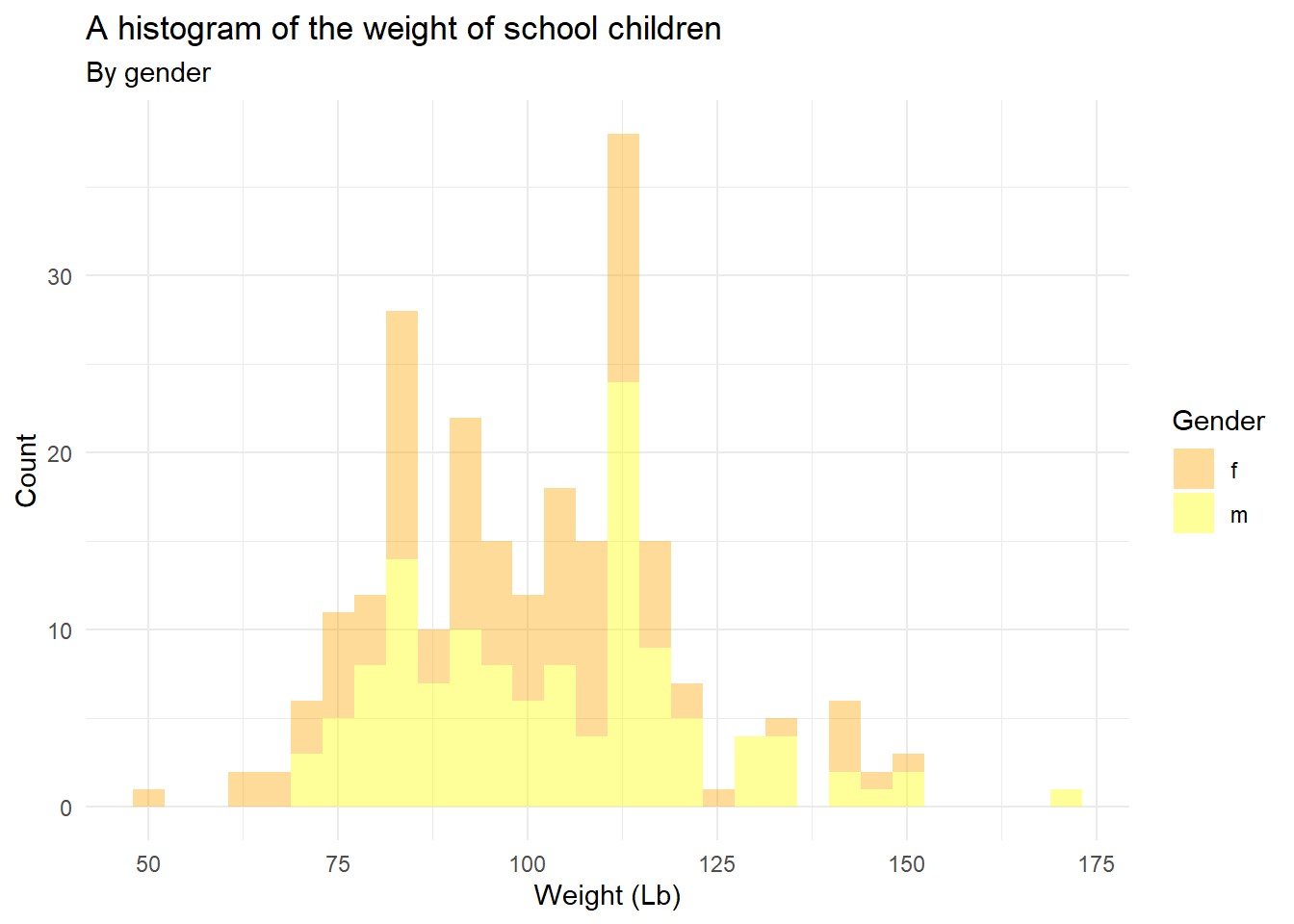### 5.10.3 Exercise 3 (without answers, I will post the answer next week, you don’t need to submit any of exercises. We don’t have any Quiz this week.)

Using the mpg dataset in the ggplot2 package, replicate the plot below using the following settings:

• Set alpha = 0.5 for the width of bars in histogram
• Use facet_grid()
• Use theme_minimal()
# you need ggplot2 package to use mpg dataset
library(ggplot2)
ggplot(mpg, aes(hwy)) +
geom_histogram(aes(fill=drv), alpha = 0.5) +
facet_grid(rows = vars(drv)) +
theme_minimal() +
labs(title = "Histogram using facet_grid()",
subtitle="Histogram of Highway Mile Per Gallon",
caption = "Source: mpg")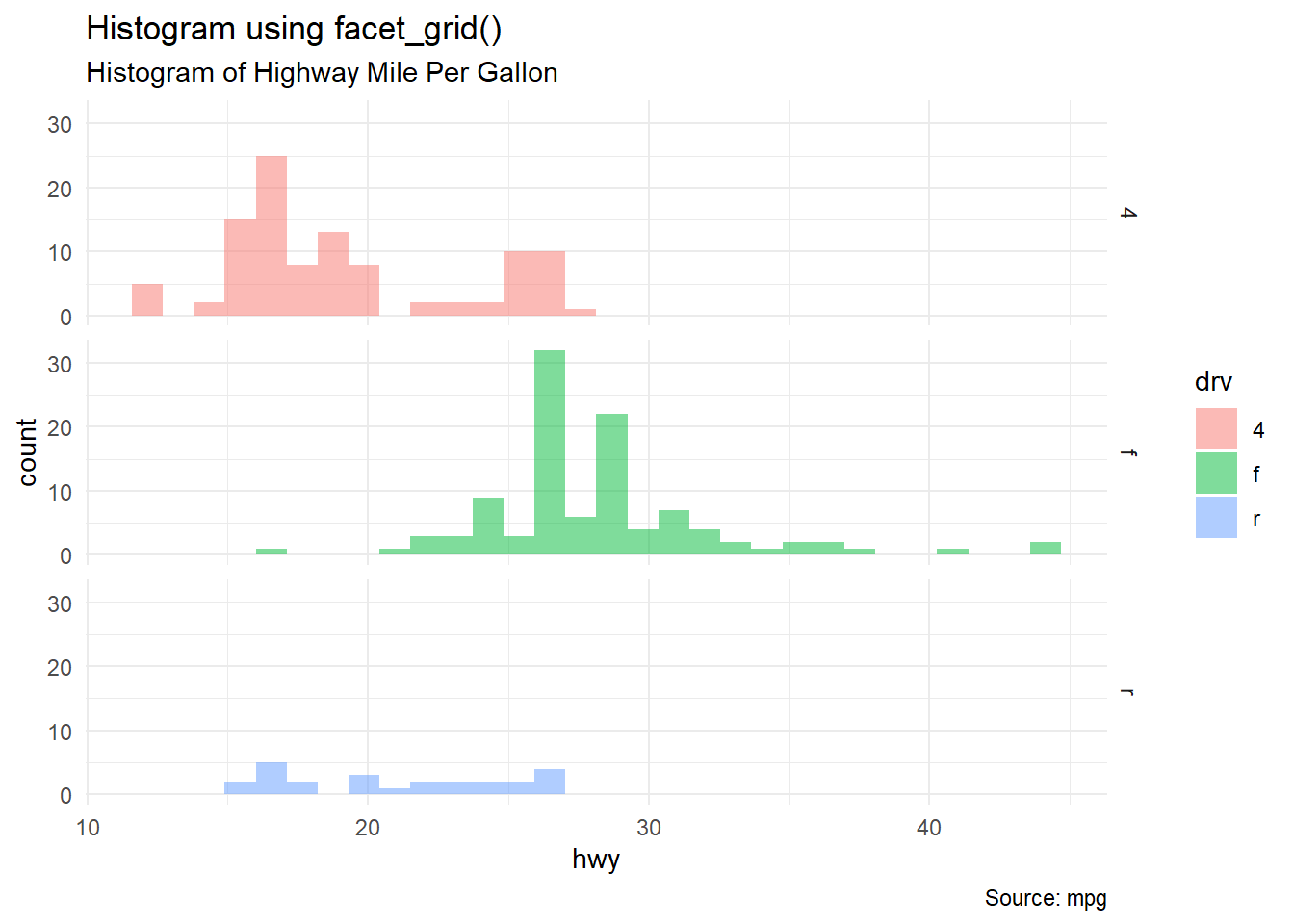### 5.10.4 Exercise 4 (without answers, I will post the answer next week, you don’t need to submit any of exercises. We don’t have any Quiz this week.)

Using the midwest dataset in the ggplot2 package, replicate the plot below using the following settings:

• Map x=area and y=poptotal
• Set alpha = 0.4
• Set the limit of x-axis is c(0, 0.1)
• Set the limit of y-axis is c(0, 500000)
• Use se=FALSE option within geom_smooth() to remove confidence bands
• Use theme_classic()
library(ggplot2) # you need ggplot2 to use midwest data
options(scipen=999)  # turn-off scientific notation like 1e+48
ggplot(midwest, aes(x=area, y=poptotal)) +
geom_point(aes(col=state, size=popdensity), alpha = 0.4) +
geom_smooth(se=FALSE) +
xlim(c(0, 0.1)) +
ylim(c(0, 500000)) +
labs(subtitle="Area Vs Population",
y="Population",
x="Area",
title="Scatterplot",
caption = "Source: midwest") +
theme_classic()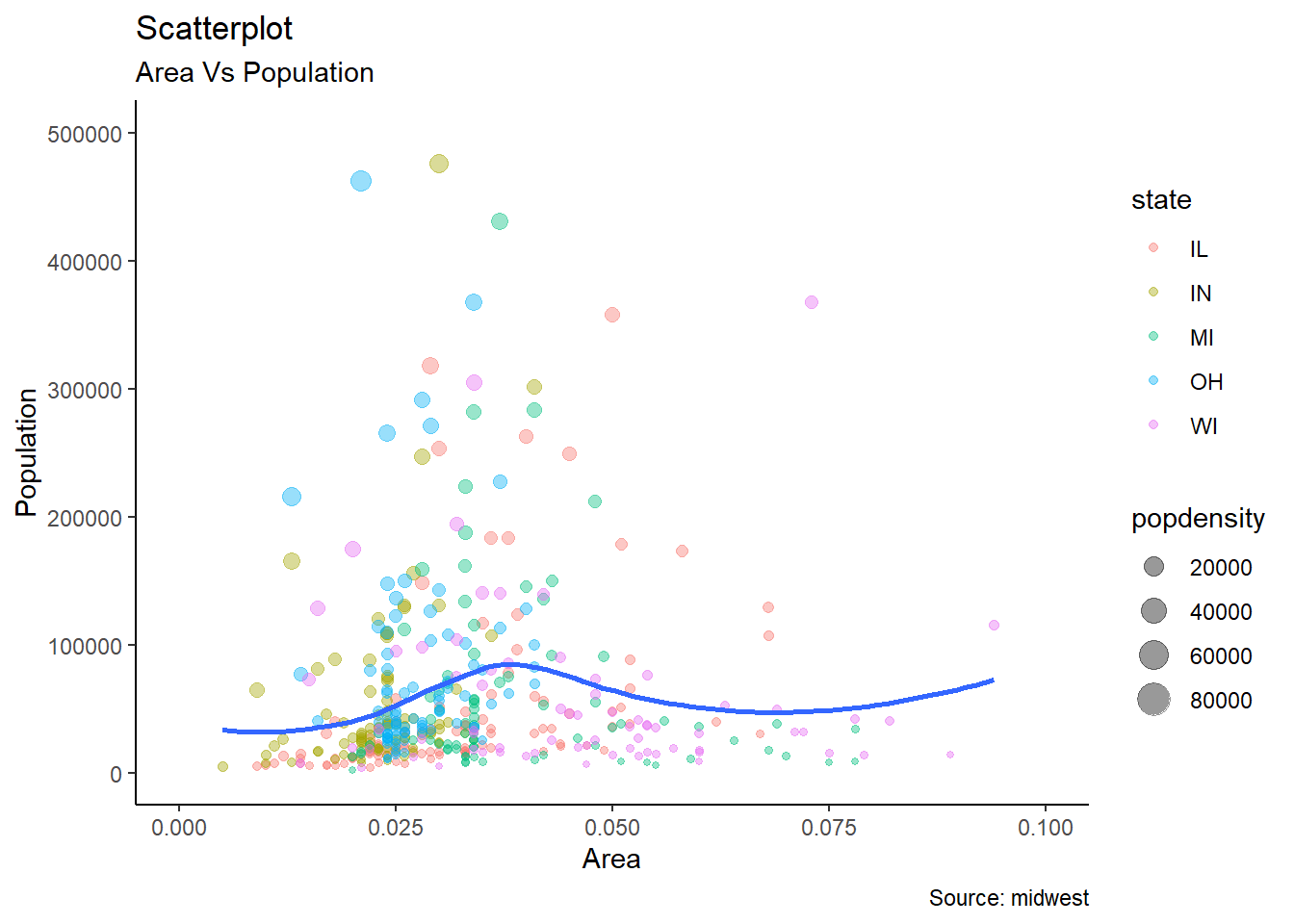### 5.10.5 Exercise 5 (without answers, I will post the answer next week, you don’t need to submit any of exercises. We don’t have any Quiz this week.)

Using the iris dataset in the datasets package (dataset package belongs to Base R and so you don’t need to download the package), replicate the plot below using the following settings:

• Set size = 6 for the size of points
• Set alpha = 0.5
• Use theme_minimal()

(iris is another famous dataset in R. You may google or check the this link to learn more about the dataset)

ggplot(iris, aes(x=Sepal.Length, y=Sepal.Width, color=Species, shape = Species)) +
geom_point(size=6, alpha = 0.5) +
labs(title="Scatterplot",
subtitle="Sepal.Length Vs Sepal.Width",
caption = "Source: iris") +
theme_minimal()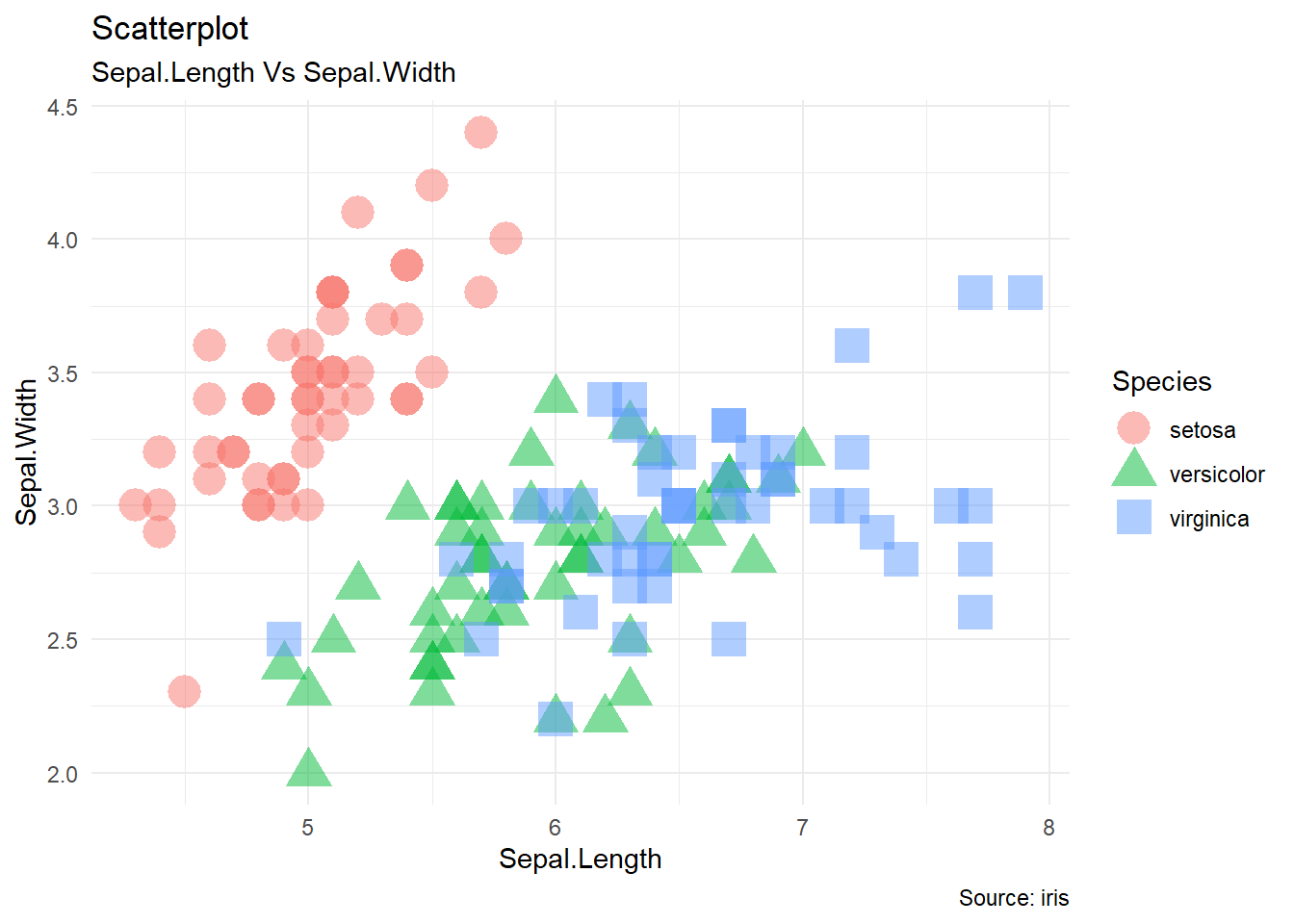### 5.10.6 Exercise 6 (without answers, I will post the answer next week, you don’t need to submit any of exercises. We don’t have any Quiz this week.)

Using the heightweight dataset in the gcookbook package, replicate the plot below using the following settings:

• Set size = 3 of points
• Set alpha = 0.5
• Use theme_classic()
library(gcookbook) # you need gcookbook to use heightweight data
ggplot(heightweight, aes(x=heightIn , y=weightLb, color=sex)) +
geom_point(size=3, alpha = 0.5) +
geom_smooth(method = "lm", se = FALSE) +
labs(title="Scatterplot",
subtitle="Weight Vs Height",
caption = "Source: heightweight"
) +
theme_classic()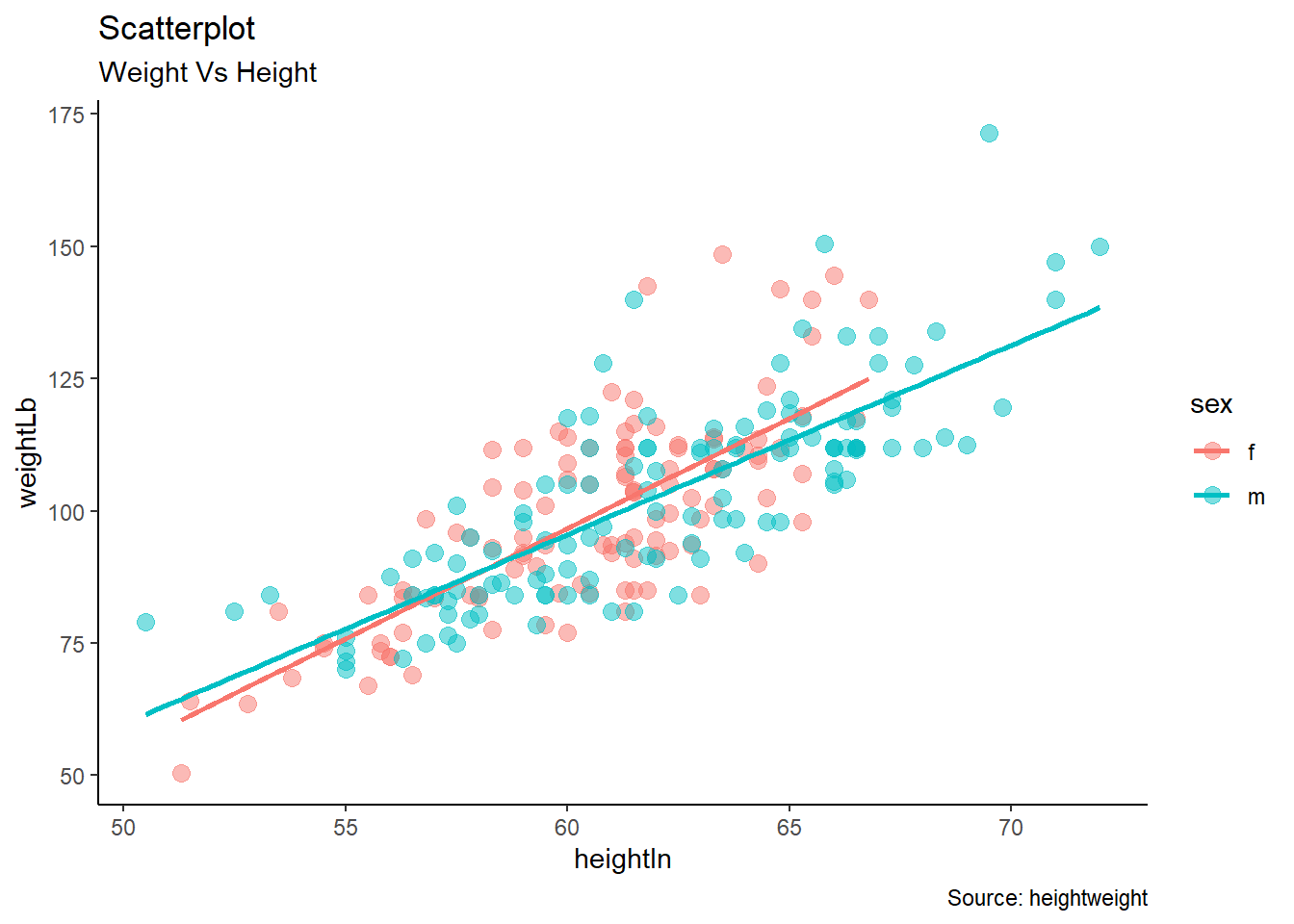### 5.10.7 Exercise 7 (without answers, I will post the answer next week, you don’t need to submit any of exercises. We don’t have any Quiz this week.)

Using the mpg dataset in the ggplot2 package, replicate the plot below using the following settings:

• Set width = 0.5 for the width of bars
• Rotate tick labels in the x-axis by 65 degree
• Use palette = "Spectral" for color
• Use theme_minimal()
library(ggplot2)
ggplot(mpg, aes(manufacturer)) + geom_bar(aes(fill=class), width = 0.5) +
theme(axis.text.x = element_text(angle=65)) +
labs(title="Barplot",
subtitle="Manufacturer across Vehicle Classes") +
scale_fill_brewer(palette = "Spectral") +
theme_minimal() +
theme(axis.text.x = element_text(angle=65, vjust=0.6))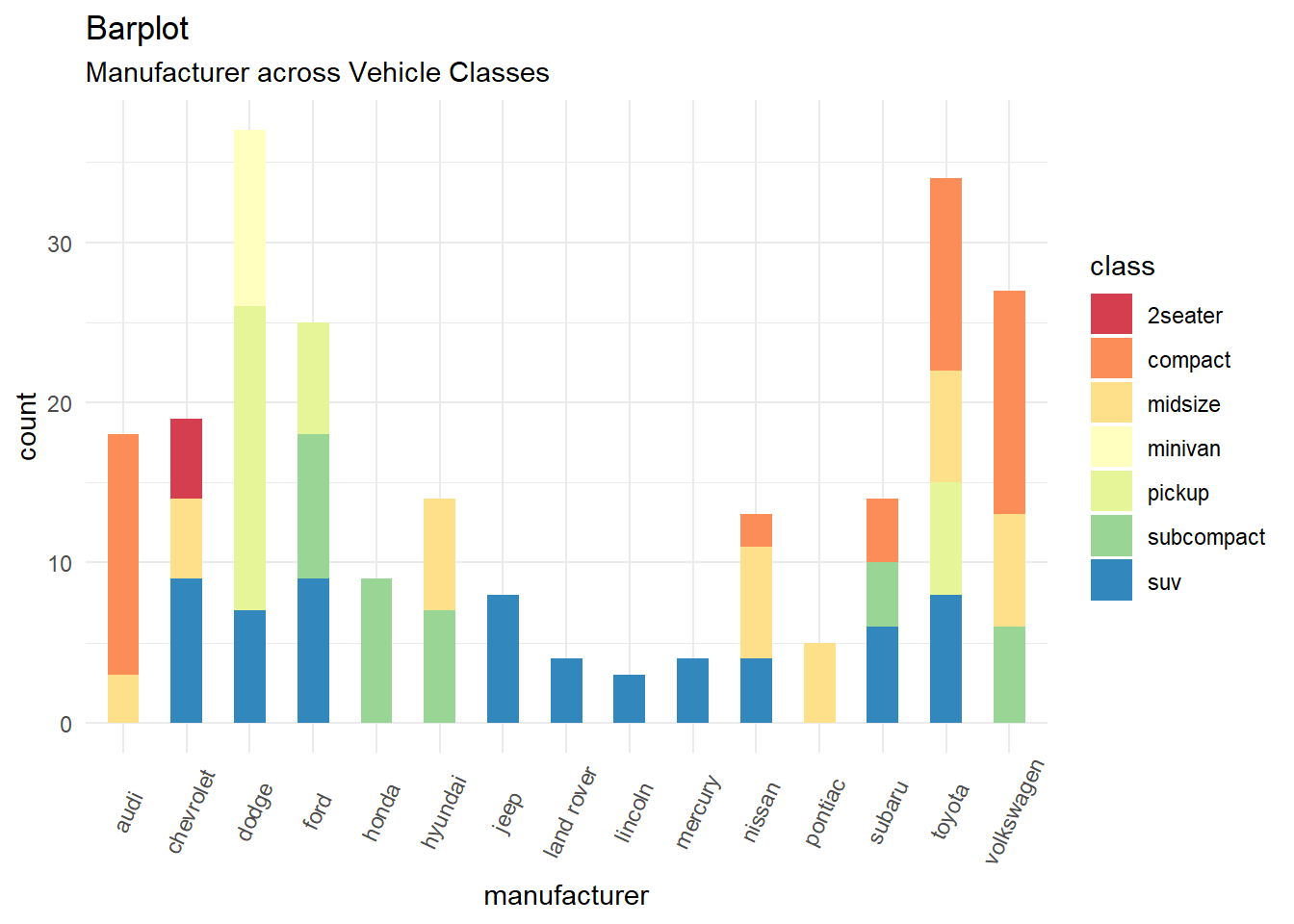### 5.10.8 Exercise 8 (without answers, I will post the answer next week, you don’t need to submit any of exercises. We don’t have any Quiz this week.)

Using the cabbage_exp dataset in the gcookbook package, replicate the plot below using the following settings:

• You need geom_text(aes(label = Weight), colour = "white", size = 4, vjust = 1.5, position = position_dodge(.9)) to put text labels.
• Use theme_minimal()
ggplot(cabbage_exp, aes(x = Date, y = Weight, fill = Cultivar)) +
geom_bar(position = "dodge", stat = "identity") +
geom_text(aes(label = Weight), colour = "white", size = 4, vjust = 1.5, position = position_dodge(.9)) + theme_minimal()